# DirectX Tutorial 1: Getting Started

## What you will need

Microsoft Visual C++ 6 (SP5)

General knowledge of Windows programming

General knowledge of C++ and Object-Oriented programming

## Introduction

Welcome to my DirectX tutorials. This is the first in a number of tutorials that should at least help you on the way to make Windows games using Microsoft DirectX 8. I have decided to write these tutorials for two reasons. Firstly, I'm a complete beginner when it comes to DirectX. So, the idea is that as I learn, I can write a short tutorial that should reinforce my knowledge. Secondly, the SDK isn't the most helpful thing in the world for complete beginners starting out in game development. Also, there isn't a great deal of stuff out there on the Internet for beginners and DirectX 8, so this should help. One other thing, as I said, I am a beginner. So, if you spot something that is incorrect in these tutorials then please let me know by emailing me at: webmaster@andypike.com.

## COM

What is COM? Well, the Component Object Model is basically a library of methods. You can create COM objects in your program and then call the methods that they expose to you. Methods are grouped together in collections of related methods. These collections are known as Interfaces. You could think of a COM object as a library of functions arranged by subject. DirectX provides a whole host of these libraries that will enable you to create 3D games. The best part is, that DirectX takes care of a lot of the hard stuff for you, so it is pretty easy to get something simple up and running.

There is a lot more to COM than that, for a full description take a look in the SDK. All you really need to worry about is that you release all of your COM objects/interfaces before your program terminates. You should make sure that you release them in the reverse order to that which you created them. For example:

1.¬ÝCreate interface A.

2.¬ÝCreate interface B.

3.¬ÝRelease interface B.

4.¬ÝRelease interface A.

You release the COM object by calling their Release method.

## Page Flipping

What is Page Flipping? Well, think of a flipbook. This is a number of pages with a slightly different drawing on each page. Then, when you hold the corner and flip the pages, it looks like the picture is moving. This is how DirectX Graphics works. You draw all of your objects onto a hidden page, known as the ‚ÄúBack Buffer‚Äù. Then when you have finished, flip it to the Front Buffer and repeat the process. As the user is looking at the new front buffer, your program will be drawing onto the back buffer.

What would happen without Page Flipping? Without Page Flipping, the user would see each object appear as it was drawn, which isn –át what you want at all.

So, your game will basically consist of a loop, known as the Game Loop. Each time around the loop you process your game logic so you know where your objects will be. Next, you clear the Back Buffer. Then draw the current scene onto it. When this is done, flip it to the front and start the loop again. This will continue until the game is shut down. You may have a number of Back Buffers, this is known as a ‚ÄúSwap Chain‚Äù.

## Devices

What is a device? Basically, a device (as far as DirectX Graphics is concerned) is your machines 3D card. You can create an interface that represents your device and then use it to draw objects onto the back buffer.

## Game Loop

What is the game loop? Well, the game loop is a code loop that loops until the program is shut down. Inside the game loop is where it all happens: objects are drawn (rendered), game logic is processed (AI, moving objects and scoring etc) and Windows messages are processed. Then it's all done again until the program is closed down.

## Creating Your First Project

Okay, that‚Äôs¬Ýenough theory lets get started. Follow the step-by-step guide below to create your first DirectX Graphics project.

1.¬ÝIn Visual C++ create a new Win32 Application.

¬Ýa.¬ÝFile‚ÜíNew

¬Ýb.¬ÝFrom the Projects tab select Win32 Application

¬Ýc.¬ÝEnter a name for your project such as ‚ÄúDX Project 1‚Äù

¬Ýd.¬ÝSelect a folder for the location of your source code files

¬Ýe.¬ÝClick Next

¬Ýf.¬ÝSelect the empty project option.

¬Ýg.¬ÝClick Finish

2.¬ÝMake sure that your project settings are correct.

¬Ýa.¬ÝProject‚ÜíSettings‚Ä¶

¬Ýb.¬ÝOn the Link tab, make sure that "d3d8.lib" is in the list of Object/Library Modules. If it isn –át simply type it in.

3.¬ÝMake sure that your search paths are correct.

¬Ýa. Tools‚ÜíOptions‚ÜíDirectories Tab

¬Ýb. In the "Show directories for" drop-down, select "include files".

¬Ýc. If it does not exist already, add the following path: <SDK INSTALL PATH>\include.

¬Ýd. Make sure that this path is at the top of the list by clicking on the up arrow button (if needed).

¬Ýe. In the "Show directories for" drop-down, select "library files".

¬Ýf. If it does not exist already, add the following path: <SDK INSTALL PATH>\lib.

¬Ýg. Make sure that this path is at the top of the list by clicking on the up arrow button (if needed).

4.¬ÝAdd the source code.

¬Ýa.¬ÝFile‚ÜíNew

¬Ýb.¬ÝFrom the Files tab, select C++ Source File

¬Ýc.¬ÝEnter a filename such as ‚ÄúMain.cpp‚Äù

¬Ýd.¬ÝCopy the code segment below, and then paste it into your new file.

5.¬ÝBuild and Run the program.

¬Ýa.¬ÝPress F7 to build your project

¬Ýb.¬ÝPress F5 to run

`#include <d3d8.h>`

`LPDIRECT3D8 g_pD3D = NULL;`

`LPDIRECT3DDEVICE8 g_pD3DDevice = NULL;`

`HRESULT InitialiseD3D(HWND hWnd) {`

`¬Ý//First of all, create the main D3D object. If it is created successfully we`

`¬Ý//should get a pointer to an IDirect3D8 interface.`

`¬Ýg_pD3D = Direct3DCreate8(D3D_SDK_VERSION);`

`¬Ýif (g_pD3D == NULL) {`

`¬Ý return E_FAIL;`

`¬Ý}`

`¬Ý//Get the current display mode`

`¬ÝD3DDISPLAYMODE d3ddm;`

`¬Ýif (FAILED(g_pD3D->GetAdapterDisplayMode(D3DADAPTER_DEFAULT, &d3ddm))) {`

`¬Ý return E_FAIL;`

`¬Ý}`

`¬Ý//Create a structure to hold the settings for our device`

`¬ÝD3DPRESENT_PARAMETERS d3dpp;`

`¬ÝZeroMemory(&d3dpp, sizeof(d3dpp));`

`¬Ý//Fill the structure.`

`¬Ý//We want our program to be windowed, and set the back buffer to a format`

`¬Ý//that matches our current display mode`

`¬Ýd3dpp.Windowed = TRUE;`

`¬Ýd3dpp.SwapEffect = D3DSWAPEFFECT_COPY_VSYNC;`

`¬Ýd3dpp.BackBufferFormat = d3ddm.Format;`

`¬Ý//Create a Direct3D device.`

`¬Ýif (FAILED(g_pD3D->CreateDevice(D3DADAPTER_DEFAULT, D3DDEVTYPE_HAL, hWnd, D3DCREATE_SOFTWARE_VERTEXPROCESSING, &d3dpp, &g_pD3DDevice))) {`

`¬Ý return E_FAIL;`

`¬Ý}`

`¬Ýreturn S_OK;`

`}`

`void Render() {`

`¬Ýif (g_pD3DDevice == NULL) {`

`¬Ý return;`

`¬Ý}`

`¬Ý//Clear the backbuffer to a green color`

`¬Ýg_pD3DDevice->Clear(0, NULL, D3DCLEAR_TARGET, D3DCOLOR_XRGB(0, 255, 0), 1.0f, 0);`

`¬Ý//Begin the scene`

`¬Ýg_pD3DDevice->BeginScene();`

`¬Ý//Rendering of our game objects will go here`

`¬Ý//End the scene`

`¬Ýg_pD3DDevice->EndScene();`

`¬Ý//Filp the back and front buffers so that whatever has been rendered on the back buffer`

`¬Ý//will now be visible on screen (front buffer).`

`¬Ýg_pD3DDevice->Present(NULL, NULL, NULL, NULL);`

`}`

`void CleanUp() {`

`¬Ýif (g_pD3DDevice != NULL) {`

`¬Ý g_pD3DDevice->Release();`

`¬Ý g_pD3DDevice = NULL;`

`¬Ý}`

`¬Ýif (g_pD3D != NULL) {`

`¬Ý g_pD3D->Release();`

`¬Ý g_pD3D = NULL;`

`¬Ý}`

`}`

`void GameLoop() {`

`¬Ý//Enter the game loop`

`¬ÝMSG msg;`

`¬ÝBOOL fMessage;`

`¬ÝPeekMessage(&msg, NULL, 0U, 0U, PM_NOREMOVE);`

`¬Ýwhile (msg.message != WM_QUIT) {`

`¬Ý fMessage = PeekMessage(&msg, NULL, 0U, 0U, PM_REMOVE);`

`¬Ý if (fMessage) {`

`¬Ý¬Ý //Process message`

`¬Ý¬Ý TranslateMessage(&msg);`

`¬Ý¬Ý DispatchMessage(&msg);`

`¬Ý } else {`

`¬Ý¬Ý //No message to process, so render the current scene`

`¬Ý¬Ý Render();`

`¬Ý }`

`¬Ý}`

`}`

`//The windows message handler`

`LRESULT WINAPI WinProc(HWND hWnd, UINT msg, WPARAM wParam, LPARAM lParam) {`

`¬Ýswitch(msg) {`

`¬Ýcase WM_DESTROY:`

`¬Ý PostQuitMessage(0);`

`¬Ý return 0;`

`¬Ý break;`

`¬Ýcase WM_KEYUP:`

`¬Ý switch (wParam)¬Ý{`

`¬Ý case VK_ESCAPE:`

`¬Ý¬Ý //User has pressed the escape key, so quit`

`¬Ý¬Ý DestroyWindow(hWnd);`

`¬Ý¬Ý return 0;`

`¬Ý¬Ý break;`

`¬Ý }`

`¬Ý break;`

`¬Ý}`

`¬Ýreturn DefWindowProc(hWnd, msg, wParam, lParam);`

`}`

`//Application entry point`

`INT WINAPI WinMain(HINSTANCE hInst, HINSTANCE, LPSTR, INT) {`

`¬Ý//Register the window class`

`¬ÝWNDCLASSEX wc = {`

`¬Ý sizeof(WNDCLASSEX), CS_CLASSDC, WinProc, 0L, 0L, GetModuleHandle(NULL), NULL, NULL, NULL, NULL, "DX Project 1", NULL`

`¬Ý};`

`¬ÝRegisterClassEx(&wc);`

`¬Ý//Create the application's window`

`¬ÝHWND hWnd = CreateWindow("DX Project 1", www.andypike.com: Tutorial 1, WS_OVERLAPPEDWINDOW, 50, 50, 500, 500, GetDesktopWindow(), NULL, wc.hInstance, NULL);`

`¬Ý//Initialize Direct3D`

`¬Ýif (SUCCEEDED(InitialiseD3D(hWnd))) {`

`¬Ý //Show our window`

`¬Ý ShowWindow(hWnd, SW_SHOWDEFAULT);`

`¬Ý UpdateWindow(hWnd);`

`¬Ý //Start game running: Enter the game loop`

`¬Ý GameLoop();`

`¬Ý}`

`¬ÝCleanUp();`

`¬ÝUnregisterClass("DX Project 1", wc.hInstance);`

`¬Ýreturn 0;`

`}`

You should finish up with a window with a green background (shown below). Okay, it –ás not much I know, but everyone has to start somewhere.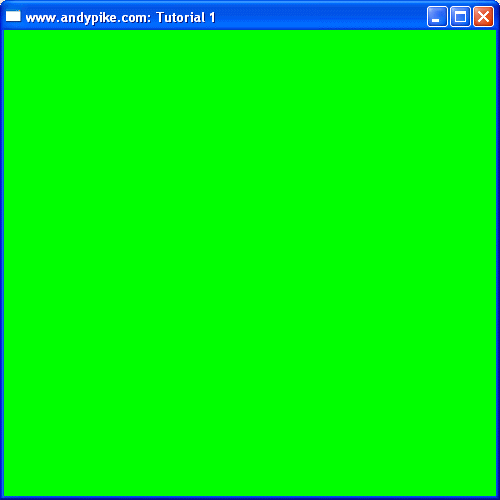So, what is going on here?

WinMain

This is the applications entry point. Code execution will start here. This is where we register, create and show our window. Once that is complete, we initialise Direct3D and enter our game loop.

WinProc

This is the applications message handler. Whenever Windows sends a message to our application, it will be handled by this function. Notice that there are two messages that our application will handle: WM_DESTROY and WM_KEYUP, all other messages are passed to DefWindowProc for default message processing.

g_pD3D

This is a pointer to an IDirect3D8 interface. From this interface we will create our Direct3D Device.

g_pD3DDevice

This is a pointer to an IDirect3DDevice8 interface. This will actually represent your hardware graphics card.

InitialiseD3D

This does exactly that: initialise Direct3D. First of all, we create the IDirect3D8 object. From this object we can determine the users current display mode. Finally, we use this information to create a compatible device.

GameLoop

Once our window is created this function is called. This function contains the main game loop. If there are no windows messages to handle, it calls our Render() function.

Render

Firstly we clear the back buffer ready for drawing. Then we use the BeginScene method of our device object to tell DirectX that we are about to start drawing. We can then start to draw our game objects (Tutorial 2). Once we have finished drawing, we use the EndScene method of our device object to tell DirectX that we have finished drawing. The final step is to "flip" (present) the back buffer, this will display our game objects to the user.

CleanUp

Simply cleans up by releasing our objects.

## Summary

Ok, that –át the most spectacular thing in the world, but just wait for the next tutorial when we will be drawing some shapes!

# DirectX Tutorial 2: Drawing a Polygon

This tutorial builds on the topics and code from the previous tutorial. Make sure you have read¬ÝTutorial 1¬Ýbefore you continue.

## Introduction

All 3D shapes are made up from a number of polygons, normally triangles. Triangles are used because they are the most efficient polygons to draw. So, if you wanted to draw a square, it is more efficient to draw two triangles next to each other rather than one square. Therefore, this tutorial will show you how to create one of the building blocks of 3D graphics: a triangular polygon.

## Vertices

What is a vertex? Vertices are points in 3D space. For example, a triangle has three vertices and a square has four vertices. You can describe a triangle by specifying where its three vertices are. To do this you need to know about coordinates.

## 2D Cartesian Coordinate System

Below are two diagrams showing how the 2D Cartesian coordinate system works.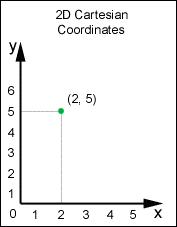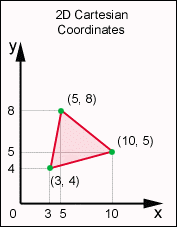In the examples above, we have an x-axis and a y-axis. Along each of these axis are numbers starting from zero (at the origin) and increasing the further along the axis you go. So, to specify a single point, all you need is an x value and a y value (see fig 2.1). It follows then, to represent a triangle you need three of these points that are joined together, each with an x value and a y value (fig 2.2). Notice that when we write a coordinate, it is always in the form: (x, y).

## 3D Cartesian Coordinate System

Below are two diagrams showing how the left-handed 3D Cartesian coordinate system works.Fig 2.3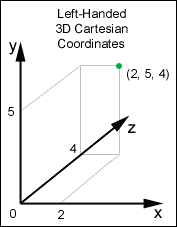Fig 2.4

As with the 2D coordinate system we have an x-axis and a y-axis. When we are dealing with 3D shapes and points we need an extra dimension ‚Äì the z-axis. This axis works in the same way with numbers starting from zero at the origin and increasing the further along the axis you go. Now, with these three axis we can specify any point in 3D space. Notice that when we write coordinates in 3D space they are always in the form: (x, y, z).

## 3D Primitives

What is a 3D primitive? Well, a 3D primitive is a collection of vertices that make up a 3D shape. There are six 3D primitives in Direct3D, you will use these primitives to draw your 3D shapes. Below are diagrams showing examples of these primitives:

Point Lists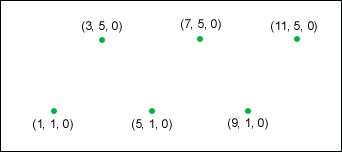## Flexible Vertex Format (FVF)

A Flexible Vertex Format or FVF is a format for describing attributes of a vertex. We've already seen three attributes: x value, y value and z value. There are other attributes that we can specify for a vertex, such as, colour and shininess. Using FVFs we can configure which attributes we want specify for a vertex. When you specify a polygon in Direct3D, the polygon can be filled based on attributes of the vertices. The fill of the polygon is interpolated (blended) between vertices. In our example below, you will see that the three vertices of our polygon are all different colours: red, green and blue. These colours are blended together across the polygon.

## Vertex Buffers

A Vertex Buffer is a memory buffer for storing vertices. A vertex buffer can stored vertices of any format. Once your vertices are stored in a vertex buffer you can perform operations such as: rendering, transforming and clipping.

## Colours

To represent colours in Direct X, we use the D3DCOLOR_XRGB macro. There are three parameters: Red, Green and Blue. These parameters are integer values between 0 and 255. By specifying different red, green and blue values (mixing colours) you can make any colour you need.

For example:

D3DCOLOR_XRGB(0, 0, 0) is black (no colour)

D3DCOLOR_XRGB(255, 255, 255) is white (full colour)

D3DCOLOR_XRGB(0, 255, 0) is bright green (no red, full green, no blue)

D3DCOLOR_XRGB(100, 20, 100) is dark purple (100 red, 20 green, 100 blue)

Here is the code for this tutorial. It's just the same as the code from the first tutorial, except for a few modifications:

`#include <d3d8.h>`

`LPDIRECT3D8 g_pD3D = NULL;`

`LPDIRECT3DDEVICE8 g_pD3DDevice = NULL;`

`LPDIRECT3DVERTEXBUFFER8 g_pVertexBuffer = NULL; // Buffer to hold vertices`

`struct CUSTOMVERTEX {`

`¬ÝFLOAT x, y, z, rhw; // The transformed position for the vertex.`

`¬ÝDWORD colour; // The vertex colour.`

`};`

`#define D3DFVF_CUSTOMVERTEX (D3DFVF_XYZRHW|D3DFVF_DIFFUSE)`

`#define SafeRelease(pObject) if(pObject != NULL) {pObject->Release(); pObject=NULL;}`

`HRESULT InitialiseD3D(HWND hWnd) {`

`¬Ý//First of all, create the main D3D object. If it is created successfully we`

`¬Ý//should get a pointer to an IDirect3D8 interface.`

`¬Ýg_pD3D = Direct3DCreate8(D3D_SDK_VERSION);`

`¬Ýif (g_pD3D == NULL) {`

`¬Ý return E_FAIL;`

`¬Ý}`

`¬Ý//Get the current display mode`

`¬ÝD3DDISPLAYMODE d3ddm;`

`¬Ýif (FAILED(g_pD3D->GetAdapterDisplayMode(D3DADAPTER_DEFAULT, &d3ddm))) {`

`¬Ý return E_FAIL;`

`¬Ý}`

`¬Ý//Create a structure to hold the settings for our device`

`¬ÝD3DPRESENT_PARAMETERS d3dpp;`

`¬ÝZeroMemory(&d3dpp, sizeof(d3dpp));`

`¬Ý//Fill the structure.`

`¬Ý//We want our program to be windowed, and set the back buffer to a format`

`¬Ý//that matches our current display mode`

`¬Ýd3dpp.Windowed = TRUE;`

`¬Ýd3dpp.SwapEffect = D3DSWAPEFFECT_COPY_VSYNC;`

`¬Ýd3dpp.BackBufferFormat = d3ddm.Format;`

`¬Ý//Create a Direct3D device.`

`¬Ýif (FAILED(g_pD3D->CreateDevice(D3DADAPTER_DEFAULT, D3DDEVTYPE_HAL, hWnd, D3DCREATE_SOFTWARE_VERTEXPROCESSING, &d3dpp, &g_pD3DDevice))) {`

`¬Ý return E_FAIL;`

`¬Ý}`

`¬Ýreturn S_OK;`

`}`

`HRESULT InitialiseVertexBuffer() {`

`¬ÝVOID* pVertices;`

`¬Ý//Store each point of the triangle together with it's colour`

`¬ÝCUSTOMVERTEX cvVertices[] = {`

`¬Ý {250.0f, 100.0f, 0.5f, 1.0f, D3DCOLOR_XRGB(255, 0, 0),}, //Vertex 1 ‚Äì Red (250, 100)`

`¬Ý {400.0f, 350.0f, 0.5f, 1.0f, D3DCOLOR_XRGB(0, 255, 0),}, //Vertex 2 ‚Äì Green (400, 350)`

`¬Ý {100.0f, 350.0f, 0.5f, 1.0f, D3DCOLOR_XRGB(0, 0, 255),}, //Vertex 3 ‚Äì Blue (100, 350)`

`¬Ý};`

`¬Ý//Create the vertex buffer from our device`

`¬Ýif (FAILED(g_pD3DDevice->CreateVertexBuffer(3 * sizeof(CUSTOMVERTEX), 0, D3DFVF_CUSTOMVERTEX, D3DPOOL_DEFAULT, &g_pVertexBuffer))) {`

`¬Ý return E_FAIL;`

`¬Ý}`

`¬Ý//Get a pointer to the vertex buffer vertices and lock the vertex buffer`

`¬Ýif (FAILED(g_pVertexBuffer->Lock(0, sizeof(cvVertices), (BYTE**)&pVertices, 0))) {`

`¬Ý return E_FAIL;`

`¬Ý}`

`¬Ý//Copy our stored vertices values into the vertex buffer`

`¬Ýmemcpy(pVertices, cvVertices, sizeof(cvVertices));`

`¬Ý//Unlock the vertex buffer`

`¬Ýg_pVertexBuffer->Unlock();`

`¬Ýreturn S_OK;`

`}`

`void Render() {`

`¬Ýif (g_pD3DDevice == NULL) {`

`¬Ý return;`

`¬Ý}`

`¬Ý//Clear the backbuffer to black`

`¬Ýg_pD3DDevice->Clear(0, NULL, D3DCLEAR_TARGET, D3DCOLOR_XRGB(0, 0, 0), 1.0f, 0);`

`¬Ý//Begin the scene`

`¬Ýg_pD3DDevice->BeginScene();`

`¬Ý//Rendering our triangle`

`¬Ýg_pD3DDevice->SetStreamSource(0, g_pVertexBuffer, sizeof(CUSTOMVERTEX));`

`¬Ýg_pD3DDevice->SetVertexShader(D3DFVF_CUSTOMVERTEX);`

`¬Ýg_pD3DDevice->DrawPrimitive(D3DPT_TRIANGLELIST, 0, 1);`

`¬Ý//End the scene`

`¬Ýg_pD3DDevice->EndScene();`

`¬Ý//Filp the back and front buffers so that whatever has been rendered on the back buffer`

`¬Ý//will now be visible on screen (front buffer).`

`¬Ýg_pD3DDevice->Present(NULL, NULL, NULL, NULL);`

`}`

`void CleanUp() {`

`¬ÝSafeRelease(g_pVertexBuffer);`

`¬ÝSafeRelease(g_pD3DDevice);`

`¬ÝSafeRelease(g_pD3D);`

`}`

`void GameLoop() {`

`¬Ý//Enter the game loop`

`¬ÝMSG msg;`

`¬ÝBOOL fMessage;`

`¬ÝPeekMessage(&msg, NULL, 0U, 0U, PM_NOREMOVE);`

`¬Ýwhile (msg.message != WM_QUIT) {`

`¬Ý fMessage = PeekMessage(&msg, NULL, 0U, 0U, PM_REMOVE);`

`¬Ý if (fMessage) {`

`¬Ý¬Ý //Process message`

`¬Ý¬Ý TranslateMessage(&msg);`

`¬Ý¬Ý DispatchMessage(&msg);`

`¬Ý } else {`

`¬Ý¬Ý //No message to process, so render the current scene`

`¬Ý¬Ý Render();`

`¬Ý }`

`¬Ý}`

`}`

`//The windows message handler`

`LRESULT WINAPI WinProc(HWND hWnd, UINT msg, WPARAM wParam, LPARAM lParam) {`

`¬Ýswitch(msg) {`

`¬Ýcase WM_DESTROY:`

`¬Ý PostQuitMessage(0);`

`¬Ý return 0;`

`¬Ý break;`

`¬Ýcase WM_KEYUP:`

`¬Ý switch (wParam) {`

`¬Ý case VK_ESCAPE:`

`¬Ý¬Ý //User has pressed the escape key, so quit`

`¬Ý¬Ý DestroyWindow(hWnd);`

`¬Ý¬Ý return 0;`

`¬Ý¬Ý break;`

`¬Ý }`

`¬Ý break;`

`¬Ý}`

`¬Ýreturn DefWindowProc(hWnd, msg, wParam, lParam);`

`}`

`//Application entry point`

`INT WINAPI WinMain(HINSTANCE hInst, HINSTANCE, LPSTR, INT) {`

`¬Ý//Register the window class`

`¬ÝWNDCLASSEX wc = {`

`¬Ý sizeof(WNDCLASSEX), CS_CLASSDC, WinProc, 0L, 0L, GetModuleHandle(NULL), NULL, NULL, NULL, NULL, "DX Project 2", NULL`

`¬Ý};`

`¬ÝRegisterClassEx(&wc);`

`¬Ý//Create the application's window`

`¬ÝHWND hWnd = CreateWindow("DX Project 2", "www.andypike.com: Tutorial 2",¬ÝWS_OVERLAPPEDWINDOW, 50, 50, 500, 500, GetDesktopWindow(), NULL, wc.hInstance, NULL);`

`¬Ý//Initialize Direct3D`

`¬Ýif (SUCCEEDED(InitialiseD3D(hWnd))) {`

`¬Ý //Show our window`

`¬Ý ShowWindow(hWnd, SW_SHOWDEFAULT);`

`¬Ý UpdateWindow(hWnd);`

`¬Ý //Initialize Vertex Buffer`

`¬Ý if (SUCCEEDED(InitialiseVertexBuffer())) {`

`¬Ý¬Ý //Start game running: Enter the game loop`

`¬Ý¬Ý GameLoop();`

`¬Ý }`

`¬Ý}`

`¬ÝCleanUp();`

`¬ÝUnregisterClass("DX Project 2", wc.hInstance);`

`¬Ýreturn 0;`

`}`

You should finish up with a window with a black background and a multi-coloured triangle in the middle (shown below).

## Summary

So, there you have it. The second tutorial complete. We've learnt a lot in this tutorial: Vertices, Coordinated Systems, 3D Primitives, FVF, Vertex Buffers and specifying colours. To take this tutorial a bit further, try adding another triangle. In the next tutorial we will create our first real 3D object.

# DirectX Tutorial 3: Rotating 3D Cube

## Introduction

In our last tutorial we drew a 2D triangle. That was good, but this time we are going to create our very first 3D object‚Ä¶ a cube. And to show that it really is 3D, we are going to rotate it about the x, y and z axis. Remember that all 3D objects are made up from polygons, normally triangles. So for our cube, we will use 12 triangles (2 for each face).

## 3D World Space

In the last tutorial we saw how the Left-Handed 3D Coordinate System worked. This is the coordinate system that we will use from now on. We will specify in our code (below) that the y axis points up.

What is the difference between left and right-handed coordinate systems? In left-handed coordinates, the positive z axis points away from you. In right-handed coordinates, the positive z axis points towards you. In both cases the positive y axis points up and the positive x axis points to the right. You can remember this by holding up your left hand so that the palm is facing up (y axis) and your fingers point to the right (x axis). Your thumb represents the positive z axis (points away). If you did the same with your right hand, your thumb would point towards you, hence left and right-handed coordinate systems. We will always use the left-handed system, this is what DirectX uses.

## Backface Culling

What is Backface Culling? Backface Culling is a pretty simple concept. Basically, it is a process where all of the polygons that are "facing" away from the user are not rendered. For example, I have created a square with one side red and the other blue. Let's say that I defined the polygons so that the red side was "facing" the user and then started rotating the square. With Backface Culling enabled, the user would only see the red face and would never see the blue face. Why is this useful? Well, if we are creating a closed 3D object (like a cube), we do not need to render the inside faces because they are never seen anyway. This makes the rendering of a cube more efficient.

How do I specify which face is "facing" the user and which face to cull (not render)? It's all in the order that you specify your vertices. Below are two diagrams showing the order in which to define a "Clockwise" polygon. If you created a polygon in this way, the polygon would be rendered as shown, but if you were to flip the polygon (rotate), it would not be rendered. You can define which faces are culled, clockwise or anti-clockwise. By default, DirectX will cull anti-clockwise polygons.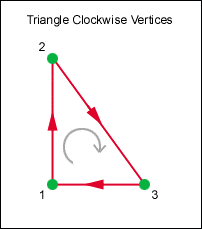Fig 3.1¬Ý¬Ý¬Ý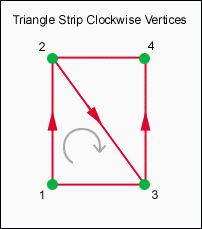Fig 3.2

## How to make a cube

Below are two diagrams showing how our cube is going to be made up. Here we have used three triangle strips, one for the top of the cube, one for the sides and one for the bottom. The diagram below shows the vertices and polygons for each triangle strip (Fig 3.3). The vertices are numbered from 0 to 17, this is the order that we must specify our vertices in the vertex buffer. Under that is a diagram that shows our cube (Fig 3.4). Notice where each of the vertices are in relation to each other. Also, look at how the vertices are always in a clockwise direction (except the bottom). This is because we have enabled Backface Culling (see above).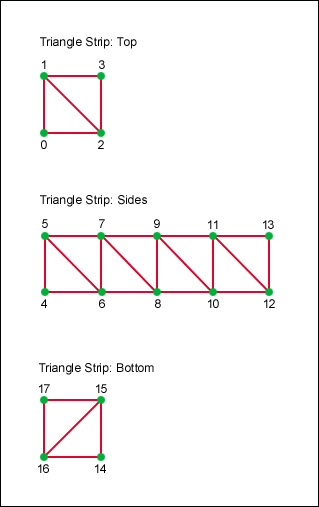Fig 3.3Fig 3.4

## Matrices

What is a Matrix? Matrices are a pretty advanced mathematics topic, so I will only give you a brief summary. A matrix can be thought of as a grid of numbers that can be applied to the coordinates of a vertex to change their values. You can use matrices in DirectX to translate, rotate and scale objects (vertices). In DirectX, a matrix is a 4x4 grid of numbers. There are three types of matrix: World, View and Projection.

World Matrix

You can use the world matrix to rotate, scale and translate objects in 3D space (World Space) by modifying their vertices. All of these transformations will be performed about the origin (0, 0, 0). You can combine transformations by multiplying them together, but be aware that it is important which order you perform the multiplication. Matrix1 x Matrix2 is not the same as Matrix2 x Matrix1. When you perform a world matrix transformation, all subsequent vertices will be transformed by this matrix. To rotate two objects, one about the x axis and one about the y axis, you must perform the x axis transformation first, then render object 1. Next, perform the y axis transformation, then render object 2.

View Matrix

The view matrix is the camera (or eye). The camera has a position in world space and also has a "look at" position. For example, you can place the camera above an object (camera position) and point it at the centre of the object (look at position). You can also specify which way is up, in our example below we will specify that the positive y axis is up.

Projection Matrix

The projection matrix can be thought of as the camera lens. It specifies the field of view angle, aspect ratio and near/far clipping planes. For the time being at least, we will keep these settings the same throughout our examples.

Here is the code for this tutorial. It's just the same as the code from the last tutorial, except for a few modifications:

`#include <d3dx8.h>`

`LPDIRECT3D8 g_pD3D = NULL;`

`LPDIRECT3DDEVICE8 g_pD3DDevice = NULL;`

`LPDIRECT3DVERTEXBUFFER8 g_pVertexBuffer = NULL; // Buffer to hold vertices`

`struct CUSTOMVERTEX {`

`¬ÝFLOAT x, y, z;`

`¬ÝDWORD colour;`

`};`

`#define D3DFVF_CUSTOMVERTEX (D3DFVF_XYZ|D3DFVF_DIFFUSE)`

`#define SafeRelease(pObject) if (pObject != NULL) {pObject->Release(); pObject=NULL;}`

`HRESULT InitialiseD3D(HWND hWnd) {`

`¬Ý//First of all, create the main D3D object. If it is created successfully we`

`¬Ý//should get a pointer to an IDirect3D8 interface.`

`¬Ýg_pD3D = Direct3DCreate8(D3D_SDK_VERSION);`

`¬Ýif (g_pD3D == NULL) {`

`¬Ý return E_FAIL;`

`¬Ý}`

`¬Ý//Get the current display mode`

`¬ÝD3DDISPLAYMODE d3ddm;`

`¬Ýif (FAILED(g_pD3D->GetAdapterDisplayMode(D3DADAPTER_DEFAULT, &d3ddm))) {`

`¬Ý return E_FAIL;`

`¬Ý}`

`¬Ý//Create a structure to hold the settings for our device`

`¬ÝD3DPRESENT_PARAMETERS d3dpp;`

`¬ÝZeroMemory(&d3dpp, sizeof(d3dpp));`

`¬Ý//Fill the structure.`

`¬Ý//We want our program to be windowed, and set the back buffer to a format`

`¬Ý//that matches our current display mode`

`¬Ýd3dpp.Windowed = TRUE;`

`¬Ýd3dpp.SwapEffect = D3DSWAPEFFECT_COPY_VSYNC;`

`¬Ýd3dpp.BackBufferFormat = d3ddm.Format;`

`¬Ý//Create a Direct3D device.`

`¬Ýif (FAILED(g_pD3D->CreateDevice(D3DADAPTER_DEFAULT, D3DDEVTYPE_HAL, hWnd, D3DCREATE_SOFTWARE_VERTEXPROCESSING, &d3dpp, &g_pD3DDevice))) {`

`¬Ý return E_FAIL;`

`¬Ý}`

`¬Ý//Turn on back face culling. This is becuase we want to hide the back of our polygons`

`¬Ýg_pD3DDevice->SetRenderState(D3DRS_CULLMODE, D3DCULL_CCW);`

`¬Ý//Turn off lighting becuase we are specifying that our vertices have colour`

`¬Ýg_pD3DDevice->SetRenderState(D3DRS_LIGHTING, FALSE);`

`¬Ýreturn S_OK;`

`}`

`HRESULT InitialiseVertexBuffer() {`

`¬ÝVOID* pVertices;`

`¬Ý//Store each point of the cube together with it's colour`

`¬Ý//Make sure that the points of a polygon are specified in a clockwise direction,`

`¬Ý//this is because anti-clockwise faces will be culled`

`¬Ý//We will use a three triangle strips to render these polygons (Top, Sides, Bottom).`

`¬ÝCUSTOMVERTEX cvVertices[] = {`

`¬Ý //Top Face`

`¬Ý {-5.0f, 5.0f, ‚Äì5.0f, D3DCOLOR_XRGB(0, 0, 255),}, //Vertex 0 ‚Äì Blue`

`¬Ý {-5.0f, 5.0f, 5.0f, D3DCOLOR_XRGB(255, 0, 0),}, //Vertex 1 ‚Äì Red`

`¬Ý {5.0f, 5.0f, ‚Äì5.0f, D3DCOLOR_XRGB(255, 0, 0),}, //Vertex 2 ‚Äì Red`

`¬Ý {5.0f, 5.0f, 5.0f, D3DCOLOR_XRGB(0, 255, 0),}, //Vertex 3 ‚Äì Green`

`¬Ý //Face 1`

`¬Ý {-5.0f, ‚Äì5.0f, ‚Äì5.0f, D3DCOLOR_XRGB(255, 0, 0),}, //Vertex 4 ‚Äì Red`

`¬Ý {-5.0f, 5.0f, ‚Äì5.0f, D3DCOLOR_XRGB(0, 0, 255),}, //Vertex 5 ‚Äì Blue`

`¬Ý {5.0f, ‚Äì5.0f, ‚Äì5.0f, D3DCOLOR_XRGB(0, 255, 0),}, //Vertex 6 ‚Äì Green`

`¬Ý {5.0f, 5.0f, ‚Äì5.0f, D3DCOLOR_XRGB(255, 0, 0),}, //Vertex 7 ‚Äì Red`

`¬Ý //Face 2`

`¬Ý {5.0f, ‚Äì5.0f, 5.0f, D3DCOLOR_XRGB(0, 0, 255),}, //Vertex 8 ‚Äì Blue`

`¬Ý {5.0f, 5.0f, 5.0f, D3DCOLOR_XRGB(0, 255, 0),}, //Vertex 9 ‚Äì Green`

`¬Ý //Face 3`

`¬Ý {-5.0f, ‚Äì5.0f, 5.0f, D3DCOLOR_XRGB(0, 255, 0),}, //Vertex 10 ‚Äì Green`

`¬Ý {-5.0f, 5.0f, 5.0f, D3DCOLOR_XRGB(255, 0, 0),}, //Vertex 11 ‚Äì Red`

`¬Ý //Face 4`

`¬Ý {-5.0f, ‚Äì5.0f, ‚Äì5.0f, D3DCOLOR_XRGB(255, 0, 0),}, //Vertex 12 ‚Äì Red`

`¬Ý {-5.0f, 5.0f, ‚Äì5.0f, D3DCOLOR_XRGB(0, 0, 255),}, //Vertex 13 ‚Äì Blue`

`¬Ý //Bottom Face`

`¬Ý {5.0f, ‚Äì5.0f, ‚Äì5.0f, D3DCOLOR_XRGB(0, 255, 0),}, //Vertex 14 ‚Äì Green`

`¬Ý {5.0f, ‚Äì5.0f, 5.0f, D3DCOLOR_XRGB(0, 0, 255),}, //Vertex 15 ‚Äì Blue`

`¬Ý {-5.0f, ‚Äì5.0f, ‚Äì5.0f, D3DCOLOR_XRGB(255, 0, 0),}, //Vertex 16 ‚Äì Red`

`¬Ý {-5.0f, ‚Äì5.0f, 5.0f, D3DCOLOR_XRGB(0, 255, 0),}, //Vertex 17 ‚Äì Green`

`¬Ý};`

`¬Ý//Create the vertex buffer from our device.`

`¬Ýif (FAILED(g_pD3DDevice->CreateVertexBuffer(18 * sizeof(CUSTOMVERTEX), 0, D3DFVF_CUSTOMVERTEX, D3DPOOL_DEFAULT, &g_pVertexBuffer))) {`

`¬Ý return E_FAIL;`

`¬Ý}`

`¬Ý//Get a pointer to the vertex buffer vertices and lock the vertex buffer`

`¬Ýif (FAILED(g_pVertexBuffer->Lock(0, sizeof(cvVertices), (BYTE**)&pVertices, 0))) {`

`¬Ý return E_FAIL;`

`¬Ý}`

`¬Ý//Copy our stored vertices values into the vertex buffer`

`¬Ýmemcpy(pVertices, cvVertices, sizeof(cvVertices));`

`¬Ý//Unlock the vertex buffer`

`¬Ýg_pVertexBuffer->Unlock();`

`¬Ýreturn S_OK;`

`}`

`void SetupRotation() {`

`¬Ý//Here we will rotate our world around the x, y and z axis.`

`¬ÝD3DXMATRIX matWorld, matWorldX, matWorldY, matWorldZ;`

`¬Ý//Create the transformation matrices`

`¬ÝD3DXMatrixRotationX(&matWorldX, timeGetTime()/400.0f);`

`¬ÝD3DXMatrixRotationY(&matWorldY, timeGetTime()/400.0f);`

`¬ÝD3DXMatrixRotationZ(&matWorldZ, timeGetTime()/400.0f);`

`¬Ý//Combine the transformations by multiplying them together`

`¬ÝD3DXMatrixMultiply(&matWorld, &matWorldX, &matWorldY);`

`¬ÝD3DXMatrixMultiply(&matWorld, &matWorld, &matWorldZ);`

`¬Ý//Apply the transformation`

`¬Ýg_pD3DDevice->SetTransform(D3DTS_WORLD, &matWorld);`

`}`

`void SetupCamera() {`

`¬Ý//Here we will setup the camera.`

`¬Ý//The camera has three settings: "Camera Position", "Look at Position" and "Up Direction"`

`¬Ý//We have set the following:`

`¬Ý//Camera Position: (0, 0, ‚Äì30)`

`¬Ý//Look at Position: (0, 0, 0)`

`¬Ý//Up direction: Y-Axis.`

`¬ÝD3DXMATRIX matView;`

`¬ÝD3DXMatrixLookAtLH(&matView, &D3DXVECTOR3(0.0f, 0.0f,-30.0f), //Camera Position`

`¬Ý &D3DXVECTOR3(0.0f, 0.0f, 0.0f), //Look At Position`

`¬Ý &D3DXVECTOR3(0.0f, 1.0f, 0.0f)); //Up Direction`

`¬Ý g_pD3DDevice->SetTransform(D3DTS_VIEW, &matView);`

`}`

`void SetupPerspective() {`

`¬Ý//Here we specify the field of view, aspect ration and near and far clipping planes.`

`¬ÝD3DXMATRIX matProj;`

`¬ÝD3DXMatrixPerspectiveFovLH(&matProj, D3DX_PI/4, 1.0f, 1.0f, 500.0f);`

`¬Ýg_pD3DDevice->SetTransform(D3DTS_PROJECTION, &matProj);`

`}`

`void Render() {`

`¬Ýif (g_pD3DDevice == NULL) {`

`¬Ý return;`

`¬Ý}`

`¬Ý//Clear the backbuffer to black`

`¬Ýg_pD3DDevice->Clear(0, NULL, D3DCLEAR_TARGET, D3DCOLOR_XRGB(0, 0, 0), 1.0f, 0);`

`¬Ý//Begin the scene`

`¬Ýg_pD3DDevice->BeginScene();`

`¬Ý//Setup the rotation, camera, and perspective matrices`

`¬ÝSetupRotation();`

`¬ÝSetupCamera();`

`¬ÝSetupPerspective();`

`¬Ý//Rendering our objects`

`¬Ýg_pD3DDevice->SetStreamSource(0, g_pVertexBuffer, sizeof(CUSTOMVERTEX));`

`¬Ýg_pD3DDevice->SetVertexShader(D3DFVF_CUSTOMVERTEX);`

`¬Ýg_pD3DDevice->DrawPrimitive(D3DPT_TRIANGLESTRIP, 0, 2); //Top`

`¬Ýg_pD3DDevice->DrawPrimitive(D3DPT_TRIANGLESTRIP, 4, 8); //Sides`

`¬Ýg_pD3DDevice->DrawPrimitive(D3DPT_TRIANGLESTRIP, 14, 2); //Bottom`

`¬Ý//End the scene`

`¬Ýg_pD3DDevice->EndScene();`

`¬Ý//Filp the back and front buffers so that whatever has been rendered on the back buffer`

`¬Ý//will now be visible on screen (front buffer).`

`¬Ýg_pD3DDevice->Present(NULL, NULL, NULL, NULL);`

`}`

`void CleanUp()¬Ý{`

`¬ÝSafeRelease(g_pVertexBuffer);`

`¬ÝSafeRelease(g_pD3DDevice);`

`¬ÝSafeRelease(g_pD3D);`

`}`

`void GameLoop() {`

`¬Ý//Enter the game loop`

`¬ÝMSG msg;`

`¬ÝBOOL fMessage;`

`¬ÝPeekMessage(&msg, NULL, 0U, 0U, PM_NOREMOVE);`

`¬Ýwhile (msg.message != WM_QUIT) {`

`¬Ý fMessage = PeekMessage(&msg, NULL, 0U, 0U, PM_REMOVE);`

`¬Ý if (fMessage) {`

`¬Ý¬Ý //Process message`

`¬Ý¬Ý TranslateMessage(&msg);`

`¬Ý¬Ý DispatchMessage(&msg);`

`¬Ý } else {`

`¬Ý¬Ý //No message to process, so render the current scene`

`¬Ý¬Ý Render();`

`¬Ý }`

`¬Ý}`

`}`

`//The windows message handler`

`LRESULT WINAPI WinProc(HWND hWnd, UINT msg, WPARAM wParam, LPARAM lParam) {`

`¬Ýswitch(msg) {`

`¬Ýcase WM_DESTROY:`

`¬Ý PostQuitMessage(0);`

`¬Ý return 0;`

`¬Ý break;`

`¬Ýcase WM_KEYUP:`

`¬Ý switch (wParam) {`

`¬Ý case VK_ESCAPE:`

`¬Ý¬Ý //User has pressed the escape key, so quit`

`¬Ý¬Ý DestroyWindow(hWnd);`

`¬Ý¬Ý return 0;`

`¬Ý¬Ý break;`

`¬Ý }`

`¬Ý break;`

`¬Ý}`

`¬Ýreturn DefWindowProc(hWnd, msg, wParam, lParam);`

`}`

`//Application entry point`

`INT WINAPI WinMain(HINSTANCE hInst, HINSTANCE, LPSTR, INT) {`

`¬Ý//Register the window class`

`¬ÝWNDCLASSEX wc = {`

`¬Ý sizeof(WNDCLASSEX), CS_CLASSDC, WinProc, 0L, 0L, GetModuleHandle(NULL), NULL, NULL, NULL, NULL, "DX Project 3", NULL`

`¬Ý};`

`¬ÝRegisterClassEx(&wc);`

`¬Ý//Create the application's window`

`¬ÝHWND hWnd = CreateWindow("DX Project 3", "www.andypike.com: Tutorial 3",¬ÝWS_OVERLAPPEDWINDOW, 50, 50, 500, 500, GetDesktopWindow(), NULL, wc.hInstance, NULL);`

`¬Ý//Initialize Direct3D`

`¬Ýif (SUCCEEDED(InitialiseD3D(hWnd))) {`

`¬Ý //Show our window`

`¬Ý ShowWindow(hWnd, SW_SHOWDEFAULT);`

`¬Ý UpdateWindow(hWnd);`

`¬Ý //Initialize Vertex Buffer`

`¬Ý if (SUCCEEDED(InitialiseVertexBuffer())) {`

`¬Ý¬Ý //Start game running: Enter the game loop`

`¬Ý¬Ý GameLoop();`

`¬Ý }`

`¬Ý}`

`¬ÝCleanUp();`

`¬ÝUnregisterClass("DX Project 3", wc.hInstance);`

`¬Ýreturn 0;`

`}`

You should finish up with a window with a black background and a multi-coloured cube in the middle rotating (shown below).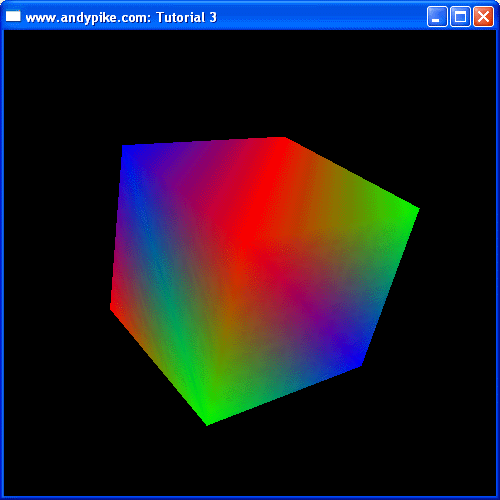So, what have we added/changed:

Include and lib files

We have a new header file, which replaces the old one. The new header file is: <d3dx8.h>

We also have two new lib files to specify in our project settings (as in tutorial 1). They are: "d3dx8.lib" and "winmm.lib".

CUSTOMVERTEX

We have changed our custom vertex to only include x, y, z and colour values. These will allow us to specify a point in 3D space and a colour.

D3DFVF_CUSTOMVERTEX

To match our custom vertex structure, we have modified our FVF. We now use the flags D3DFVF_XYZ and D3DFVF_DIFFUSE.

InitaliseD3D

We have enabled Backface Culling (explained above). We use the SetRenderState function to do this, notice that we use the D3DCULL_CCW flag to specify that we want DirectX to cull the anti-clockwise faces.

We have also used the SetRenderState function to specify that we want to disable lighting, this is because we have given a colour (light) value to each of our vertices.

InitaliseVertexBuffer

Here we have set the values for our 18 vertices. Each vertex has a comment next to it with it's number, these numbers match the diagrams above (Fig 3.3 and 3.4). The cube is centred about the origin (0, 0, 0) and is 10 units wide/high/deep.

SetupRotation

SetupRotation is a new function. Here we call the functions D3DXMatrixRotationX, D3DXMatrixRotationY and D3DXMatrixRotationZ to generate rotation matrices and store them in three D3DXMATRIX structures. Next, we multiply the three matrices together to form one world matrix, we call the SetTransform function to apply the transformation to our vertices.

SetupCamera

SetupCamera is a new function. Here we setup the camera. We set it's position in 3D space to be (0, 0, ‚Äì30) and point it at the origin (0,0,0). We have centred our cube around the origin. We also specify that the y axis points up. We use the D3DXMatrixLookAtLH to generate the view matrix and then use the SetTransform function to apply the transformation.

SetupPerspective

SetupPerspective is another new function. Here we setup the camera's lens. We have decided to have a field of view of PI/4 (normal) and an aspect ratio of 1. We have decided to set the near clipping path to 1, this means that polygons closer than one unit to the camera will be cropped. We have also decided to set the far clipping path to 500, this means that polygons more than 500 units away will be cropped.

Render

In the Render function, we call the three new functions SetupRotation, SetupCamera and SetupPerspective. These functions are called before we render the polygons.

We render the polygons by using three triangle strips, one for the top, one for the sides and one for the bottom.

## Summary

That's it for another tutorial. In this tutorial we learnt about Backface Culling, Matrices, 3D World Space and how a cube is made up using triangular polygons. In the next tutorial, we will arrange our code so far into classes and make our application full screen.

# DirectX Tutorial 4: Full Screen and Depth Buffers

## Introduction

In this tutorial we will convert our single file into two classes: CGame and CCuboid. CGame will contain the main code such as initialisation, the game loop and rendering. CCuboid will be used to create cuboid objects, you can specify position and size. CCuboid also has a Render function that should be called in the CGame render function. I have not made any major changes to the code from the last tutorial, so you should find it pretty easy to understand. We will change our program to be full screen rather than windowed and we'll take a look at depth buffers. From now on, I will not show all of the program code in the tutorial as I have done so far (they're getting to long). Instead, I'll only show you the snippets of code that are new or have been modified. You can download the full source code by clicking the "Download Source" link above.

## Depth Buffers

Using a Depth Buffer (also called a z-buffer) ensures that polygons are rendered correctly based on their depth (distance from the camera). Lets say, for example, that in your scene you have two squares ‚Äì one blue and one green. The blue one has a z value of 10 and the green square has a z value of 20 (the camera is at the origin). This means that the blue square is in front of the green one. A depth buffer is used to make sure that where one object is in front of another, the correct one is rendered. DirectX will test a pixel on the screen against an object to see how close it is to the camera. It stores this value in the depth buffer. It will then test the same pixel against the next object and compares it's distance with the value held in the depth buffer. If it is shorter, it'll overwrite the old value with the new one, otherwise it will be ignored (there is something in front of it). This will determine what colour the pixel will be, blue or green. Fig 4.1 below, shows this for a given pixel on the rendering surface.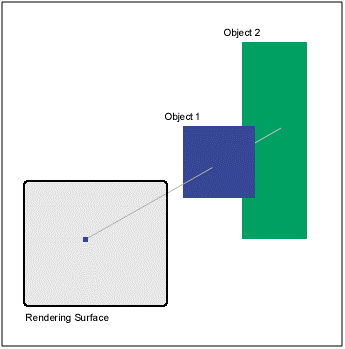Fig 4.1

To use a depth buffer in your program is pretty easy. All you need to do is select the correct format in your InitialiseD3D function, enable depth buffering and ensure that you clear the depth buffer in your Render function. In the source for this tutorial (download above), I have added some code to select a depth buffer format in the InitialiseD3D method of CGame. There are two properties of the D3DPRESENT_PARAMETERS structure that need to be set:

`d3dpp.AutoDepthStencilFormat = D3DFMT_D16;`

`d3dpp.EnableAutoDepthStencil = TRUE;`

To enable depth buffering, you need to add the following line to your InitialiseD3D method.

`m_pD3DDevice->SetRenderState(D3DRS_ZENABLE, D3DZB_TRUE);`

Then in the Render method of CGame, make sure that you clear the depth buffer as well as the back buffer by adding the D3DCLEAR_ZBUFFER flag to the device clear method, shown below.

`m_pD3DDevice->Clear(0, NULL, D3DCLEAR_TARGET|D3DCLEAR_ZBUFFER, D3DCOLOR_XRGB(0, 0, 0), 1.0f, 0);`

## Full Screen

To make our program full screen we need to make a few adjustments to our InitialiseD3D method. We need to change the "Windowed" property of our D3DPRESENT_PARAMETERS structure to FALSE, this specifies that the program will run full screen. Then, we need to set two full screen properties of the D3DPRESENT_PARAMETERS structure. FullScreen_RefreshRateInHz controls the refresh rate of the screen, and FullScreen_PresentationInterval controls the maximum rate that you swap chain (back buffer chain) are flipped. We have selected D3DPRESENT_INTERVAL_ONE which specifies that flipping will only occur when the monitor refreshes.

`d3dpp.Windowed = FALSE;`

`d3dpp.FullScreen_RefreshRateInHz = D3DPRESENT_RATE_DEFAULT;`

`d3dpp.FullScreen_PresentationInterval = D3DPRESENT_INTERVAL_ONE;`

Also, we need to select the correct back buffer format for our device. I have written a method, CheckDisplayMode, which will test for a valid format. This method is called in InitialiseD3D.

`D3DFORMAT CGame::CheckDisplayMode(UINT nWidth, UINT nHeight, UINT nDepth) {`

`¬ÝUINT x;`

`¬ÝD3DDISPLAYMODE d3ddm;`

`¬Ýfor (x = 0; x < m_pD3D->GetAdapterModeCount(0); x++) {`

`¬Ý m_pD3D->EnumAdapterModes(0, x, &d3ddm);`

`¬Ý if (d3ddm.Width == nWidth) {`

`¬Ý¬Ý if (d3ddm.Height == nHeight) {`

`¬Ý¬Ý¬Ý if ((d3ddm.Format == D3DFMT_R5G6B5) || (d3ddm.Format == D3DFMT_X1R5G5B5) || (d3ddm.Format == D3DFMT_X4R4G4B4)) {`

`¬Ý¬Ý¬Ý¬Ý if (nDepth == 16) {`

`¬Ý¬Ý¬Ý¬Ý¬Ý return d3ddm.Format;`

`¬Ý¬Ý¬Ý¬Ý }`

`¬Ý¬Ý¬Ý } else if((d3ddm.Format == D3DFMT_R8G8B8) || (d3ddm.Format == D3DFMT_X8R8G8B8)) {`

`¬Ý¬Ý¬Ý¬Ý if (nDepth == 32) {`

`¬Ý¬Ý¬Ý¬Ý¬Ý return d3ddm.Format;`

`¬Ý¬Ý¬Ý¬Ý¬Ý}`

`¬Ý¬Ý¬Ý }`

`¬Ý¬Ý }`

`¬Ý }`

`¬Ý}`

`¬Ýreturn D3DFMT_UNKNOWN;`

`}`

The only other thing we need to do is modify our WinMain function that created our window. We need to set the x and y positions of the window to be 0 (top left) and we need to change the width and height to the screen size using the GetSystemMetrics function.

## Logging

I have also added the ability to create a log file, this can prove useful when debugging. I have added to methods to CGame: EnableLogging and WriteToLog. I call EnableLogging in WinMain just after the CGame object has been created, this method clears the current log and enables logging. WriteToLog simply writes text to the log file if logging is enabled. When the program closes down, statistics are also added to the log file. You should notice that the frames per second stat is no higher than your monitor refresh rate, this is due to setting d3dpp.FullScreen_PresentationInterval = D3DPRESENT_INTERVAL_ONE. The log file is called "log.txt" and should be in your project folder once the code has been run for the first time.

You should finish up with six rotating cuboids of different sizes and positions (shown below).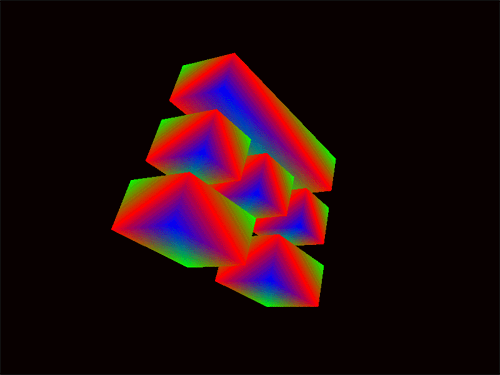## Summary

We haven't really added a lot of code in this tutorial, but we have re-organised it into classes. Take some time to familiarise yourself with the new structure, you'll see that most of the functions are unchanged. Full screen rendering and depth buffers are pretty straightforward, now that we've created them we don't really need to worry about them any further. In the next tutorial we will look into matrix transformations. We touched on them in the last tutorial, but this time we will go a little further and demonstrate the power of matrix transformations.

# DirectX Tutorial 5: Matrix Transformations

## Introduction

In this tutorial we will look more into matrix transformations. With matrix transformations you can rotate, scale and move vertices (and therefore objects). We will see how to rotate five cubes, all in a different way. One around the x axis, one around the y axis, one around the z axis, one around a user defined axis and we'll scale and rotate another cube around all three axis (x, y and z). You can download the full source code by clicking the "Download Source" link above.

## What are matrices and how do they work?

Well, matrices are quite an advanced maths topic so I'll try my best to explain the basics. A matrix is a grid of numbers that we can use in 3D graphics to modify the position of vertices (points in 3D space). Some of the main uses for matrices in 3D graphics are to rotate, scale and translate (move) vertices. So, what does a matrix look like? In DirectX, a matrix is a 4x4 grid of numbers; Fig 5.1 below shows an example matrix that will scale a vertex by five units: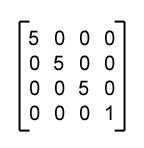Fig 5.1

OK, so how can a matrix change the position values of a vertex? To modify the x, y and z values of a vertex you need to multiply them by the matrix. Fig 5.2 below shows an example of multiplying a vertex by a matrix: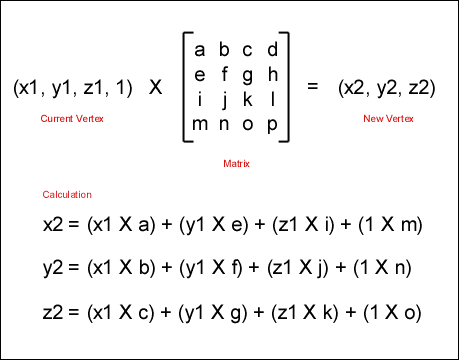Fig 5.2

This is a pretty simple calculation, all you need to do is multiply each of the x, y and z values by the columns one by one. Each column will give you one of the new values for the vertex. You may notice that in the "current vertex" in our example, we have added a 1 after the z value. This is simply to balance the vertex against the matrix. You need to have the same number of values, as there are columns for the transformation to work correctly.

So, all you need to rotate, scale or translate (move) a vertex is the correct matrix. Luckily for you, DirectX has a number of functions for generating these common matrices. What if you want to scale AND rotate some vertices? Well, first you need to have two matrices, one for the rotation and one for the scale. Then you need to multiply the two matrices (for scale and rotate) together to form one new matrix that scales and rotates. This new matrix is then applied to the vertices. You must be careful to have the matrices in the right order. MatrixA X MatrixB will give you a different answer than MatrixB X MatrixA. Fig 5.3 shows how to multiply two matrices together: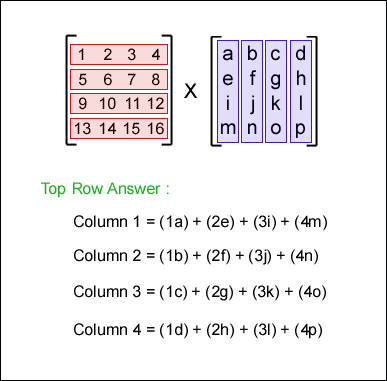Fig 5.3

To multiply two matrices together you need to multiply each row in the first matrix with each column in the second. In the example above, we have multiplied the first row (in the first matrix) by each column (in the second matrix). These four calculations will give us the top row of our answer matrix. To calculate the other three rows, simply multiply the second, third and forth rows in the first matrix by each column in the second. As I said above, DirectX has a built in function for multiplying two matrices, so don't worry too much about this!

## How do I use transformation matrices in DirectX?

In the code for this tutorial, we will have 5 cubes in different positions all rotating differently. Here is a walkthtough of the code and how it works.

Step 1: Create objects

The first thing we need to do is create and define our five cubes. I have defined 5 cubes as member variables and modified the InitialiseGame method of CGame to create and set their centre position. Their default size is 10x10x10 which I haven't changed. The InitialiseGame method is now as follows:

`bool CGame::InitialiseGame() {`

`¬Ý//Setup games objects here`

`¬Ým_pCube1 = new CCuboid(m_pD3DDevice);`

`¬Ým_pCube1->SetPosition(-27.0, 0.0, 0.0);`

`¬Ým_pCube2 = new CCuboid(m_pD3DDevice);`

`¬Ým_pCube2->SetPosition(-9.0, 0.0, 0.0);`

`¬Ým_pCube3 = new CCuboid(m_pD3DDevice);`

`¬Ým_pCube3->SetPosition(9.0, 0.0, 0.0);`

`¬Ým_pCube4 = new CCuboid(m_pD3DDevice);`

`¬Ým_pCube4->SetPosition(27.0, 0.0, 0.0);`

`¬Ým_pCube5 = new CCuboid(m_pD3DDevice);`

`¬Ým_pCube5->SetPosition(0.0, 15.0, 0.0);`

`¬Ýreturn true;`

`}`

Fig 5.4 below shows the initial positions of our five cubes. We would normally create all of these objects at the origin, and the translate them. But for the purpose of this tutorial, we'll create them in the positions show below. The centre coordinates are shown with each cube.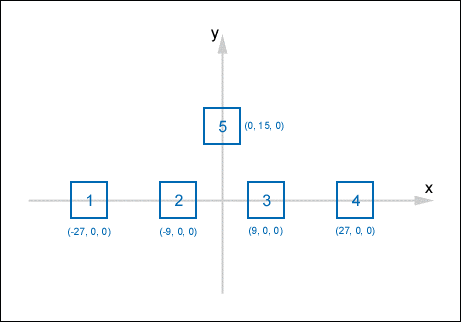Fig 5.4

Step 2: Create Transformation Matrices

The next step is to create our transformation matrices. We want our cubes to all rotate differently, so we need a different transformation matrix for each one. Cube 1, 2 and 3 will rotate around the x, y, and z axis respectively. Cube 4 will rotate around a user defined axis and cube 5 will rotate around the x, y and z axis, and it will be enlarged (scaled).

To create the x, y and z rotation matrices, we will use the DirectX functions D3DXMatrixRotationX, D3DXMatrixRotationY and D3DXMatrixRotationZ. The first parameter is a pointer to a D3DXMATRIX structure that will hold the rotation matrix, the second parameter is the angle to rotate in radians. The code snippet below, taken from our new Render method, shows these calls.

`//Create the rotation transformation matrices around the x, y and z axis`

`D3DXMatrixRotationX(&matRotationX, timeGetTime()/400.0f);`

`D3DXMatrixRotationY(&matRotationY, timeGetTime()/400.0f);`

`D3DXMatrixRotationZ(&matRotationZ, timeGetTime()/400.0f);`

The next thing to do is create the rotation matrix around a user define axis. To do this, we will use the D3DXMatrixRotationAxis function of DirectX. The first parameter is a pointer to a D3DXMATRIX structure that will hold the rotation matrix. The second parameter is a pointer to a D3DXVECTOR3 structure that defines our user defined axis. We want our axis to be a 45 degree angle between the x and y axis, so we define our access by using the following vector (1, 1, 0). Fig 5.5 below shows how to define our axis. The third parameter is the angle to rotate in radians.

`//Create the rotation transformation matrices around our user defined axis`

`D3DXMatrixRotationAxis(&matRotationUser1, &D3DXVECTOR3(1.0f, 1.0f, 0.0f), timeGetTime()/400.0f);`Fig 5.5

In addition to rotation, we will need to create some other matrices. We will need a matrix to scale cube 5 so that it is 50% larger. We will also need some matrices to translate (move) our cube to the origin and back again (I'll explain why later). So, we use the following code to create these matrices:

`//Create the translation (move) matrices`

`D3DXMatrixTranslation(&matMoveRight27, 27.0, 0.0, 0.0);`

`D3DXMatrixTranslation(&matMoveLeft27, ‚Äì27.0, 0.0, 0.0);`

`D3DXMatrixTranslation(&matMoveRight9, 9.0, 0.0, 0.0);`

`D3DXMatrixTranslation(&matMoveLeft9, ‚Äì9.0, 0.0, 0.0);`

`D3DXMatrixTranslation(&matMoveDown15, 0.0, ‚Äì15.0, 0.0);`

`D3DXMatrixTranslation(&matMoveUp15, 0.0, 15.0, 0.0);`

`//Create a scale transformation`

`D3DXMatrixScaling(&matScaleUp1p5, 1.5, 1.5, 1.5);`

## Step 3: Multiply Matrices

Now that we have our matrices, we need to multiply them together to create one transformation matrix for each cube. We will use the DirectX function D3DXMatrixMultiply to do this. Cube 1 is easy, we want it to rotate around the x axis. Becuase it's center is on the x axis, all we need to do is use the x axis rotation matrix, that means that we don't need to multiply any matrices together because we already have this matrix defined. Cubes 2-5 are a bit different, they are not on the axis that we want to rotate them around. So, what we need to do is move them on to their rotation axis, rotate them, then move them back to their starting positions. If we just rotate them without moving them, the cubes will just swing around the axis rather than staying in the same position and rotating. Fig 5.6 shows a cube swinging around the y axis. Fig 5.7 shows a cube being moved to the origin, rotated, them moved back.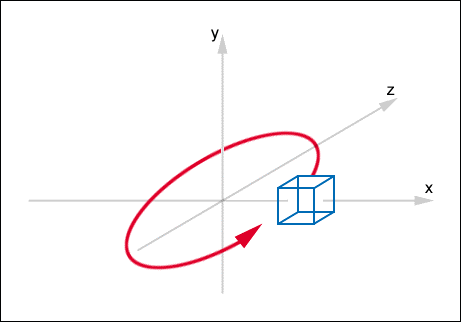Fig 5.6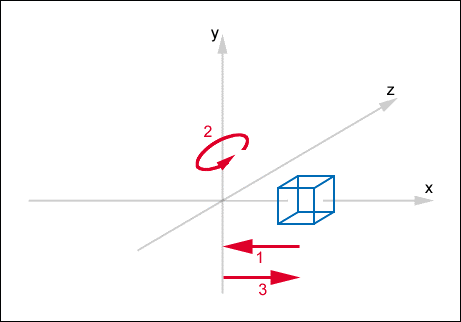Fig 5.7

We have the matrix for cube 1, so now we need to create a matrix for cubes 2, 3, 4 and 5. The follow code snippet shows how to do this. Notice the order in which the matrices are multiplied together. If you change this order, you will get a different result.

`//Combine the matrices to form 4 transformation matrices`

`D3DXMatrixMultiply(&matTransformation2, &matMoveRight9, &matRotationY);`

`D3DXMatrixMultiply(&matTransformation2, &matTransformation2, &matMoveLeft9);`

`D3DXMatrixMultiply(&matTransformation3, &matMoveLeft9, &matRotationZ);`

`D3DXMatrixMultiply(&matTransformation3, &matTransformation3, &matMoveRight9);`

`D3DXMatrixMultiply(&matTransformation4, &matMoveLeft27, &matRotationUser1);`

`D3DXMatrixMultiply(&matTransformation4, &matTransformation4, &matMoveRight27);`

`D3DXMatrixMultiply(&matTransformation5, &matMoveDown15, &matRotationY);`

`D3DXMatrixMultiply(&matTransformation5, &matTransformation5, &matRotationX);`

`D3DXMatrixMultiply(&matTransformation5, &matTransformation5, &matRotationZ);`

`D3DXMatrixMultiply(&matTransformation5, &matTransformation5, &matMoveUp15);`

`D3DXMatrixMultiply(&matTransformation5, &matTransformation5, &matScaleUp1p5);`

Step 4: Applying the Transformations

Now that we have a transformation matrix for each cube, we need to apply them and then render the cubes. To apply a transformation matix, we use the SetTransform method. When you call SetTransform with a transformation matrix, all further objects that are rendered will have that matrix appied to them. So, for each cube we need to call SetTransform with that cubes transformation matrix, and then render that cube. The code snippet below shows this:

`//Apply the transformations and render our objects`

`m_pD3DDevice->SetTransform(D3DTS_WORLD, &matRotationX);`

`m_pCube1->Render();`

`m_pD3DDevice->SetTransform(D3DTS_WORLD, &matTransformation2);`

`m_pCube2->Render();`

`m_pD3DDevice->SetTransform(D3DTS_WORLD, &matTransformation3);`

`m_pCube3->Render();`

`m_pD3DDevice->SetTransform(D3DTS_WORLD, &matTransformation4);`

`m_pCube4->Render();`

`m_pD3DDevice->SetTransform(D3DTS_WORLD, &matTransformation5);`

`m_pCube5->Render();`

The full new render method for this tutorial is shown below. One thing to note is that I have moved the code from SetupPerspective() into the SetupCamera() function (just trying to keep the code simple).

`void CGame::Render() {`

`¬ÝD3DXMATRIX matRotationX, matRotationY, matRotationZ, matRotationUser1;`

`¬ÝD3DXMATRIX matMoveRight27, matMoveLeft27, matMoveRight9, matMoveLeft9, matMoveDown15, matMoveUp15;`

`¬ÝD3DXMATRIX matTransformation2, matTransformation3, matTransformation4, matTransformation5;`

`¬ÝD3DXMATRIX matScaleUp1p5;`

`¬Ýif (m_pD3DDevice == NULL) {`

`¬Ý return;`

`¬Ý}`

`¬Ý//Clear the back buffer and depth buffer`

`¬Ým_pD3DDevice->Clear(0, NULL, D3DCLEAR_TARGET|D3DCLEAR_ZBUFFER, D3DCOLOR_XRGB(0, 0, 0), 1.0f, 0);`

`¬Ý//Begin the scene`

`¬Ým_pD3DDevice->BeginScene();`

`¬Ý//Setup camera and perspective`

`¬ÝSetupCamera();`

`¬Ý//Create the rotation transformation matrices around the x, y and z axis`

`¬ÝD3DXMatrixRotationX(&matRotationX, timeGetTime()/400.0f);`

`¬ÝD3DXMatrixRotationY(&matRotationY, timeGetTime()/400.0f);`

`¬ÝD3DXMatrixRotationZ(&matRotationZ, timeGetTime()/400.0f);`

`¬Ý//Create the rotation transformation matrices around our user defined axis`

`¬ÝD3DXMatrixRotationAxis(&matRotationUser1, &D3DXVECTOR3(1.0f, 1.0f, 0.0f), timeGetTime()/400.0f);`

`¬Ý//Create the translation (move) matrices`

`¬ÝD3DXMatrixTranslation(&matMoveRight27, 27.0, 0.0, 0.0);`

`¬ÝD3DXMatrixTranslation(&matMoveLeft27, ‚Äì27.0, 0.0, 0.0);`

`¬ÝD3DXMatrixTranslation(&matMoveRight9, 9.0, 0.0, 0.0);`

`¬ÝD3DXMatrixTranslation(&matMoveLeft9, ‚Äì9.0, 0.0, 0.0);`

`¬ÝD3DXMatrixTranslation(&matMoveDown15, 0.0, ‚Äì15.0, 0.0);`

`¬ÝD3DXMatrixTranslation(&matMoveUp15, 0.0, 15.0, 0.0);`

`¬Ý//Create a scale transformation`

`¬ÝD3DXMatrixScaling(&matScaleUp1p5, 1.5, 1.5, 1.5);`

`¬Ý//Combine the matrices to form 4 transformation matrices`

`¬ÝD3DXMatrixMultiply(&matTransformation2, &matMoveRight9, &matRotationY);`

`¬ÝD3DXMatrixMultiply(&matTransformation2, &matTransformation2, &matMoveLeft9);`

`¬ÝD3DXMatrixMultiply(&matTransformation3, &matMoveLeft9, &matRotationZ);`

`¬ÝD3DXMatrixMultiply(&matTransformation3, &matTransformation3, &matMoveRight9);`

`¬ÝD3DXMatrixMultiply(&matTransformation4, &matMoveLeft27, &matRotationUser1);`

`¬ÝD3DXMatrixMultiply(&matTransformation4, &matTransformation4, &matMoveRight27);`

`¬ÝD3DXMatrixMultiply(&matTransformation5, &matMoveDown15, &matRotationY);`

`¬ÝD3DXMatrixMultiply(&matTransformation5, &matTransformation5, &matRotationX);`

`¬ÝD3DXMatrixMultiply(&matTransformation5, &matTransformation5, &matRotationZ);`

`¬ÝD3DXMatrixMultiply(&matTransformation5, &matTransformation5, &matMoveUp15);`

`¬ÝD3DXMatrixMultiply(&matTransformation5, &matTransformation5, &matScaleUp1p5);`

`¬Ý//Apply the transformations and render our objects`

`¬Ým_pD3DDevice->SetTransform(D3DTS_WORLD, &matRotationX);`

`¬Ým_pCube1->Render();`

`¬Ým_pD3DDevice->SetTransform(D3DTS_WORLD, &matTransformation2);`

`¬Ým_pCube2->Render();`

`¬Ým_pD3DDevice->SetTransform(D3DTS_WORLD, &matTransformation3);`

`¬Ým_pCube3->Render();`

`¬Ým_pD3DDevice->SetTransform(D3DTS_WORLD, &matTransformation4);`

`¬Ým_pCube4->Render();`

`¬Ým_pD3DDevice->SetTransform(D3DTS_WORLD, &matTransformation5);`

`¬Ým_pCube5->Render();`

`¬Ý//End the scene`

`¬Ým_pD3DDevice->EndScene();`

`¬Ý//Filp the back and front buffers so that whatever has been rendered on the back buffer`

`¬Ý//will now be visible on screen (front buffer).`

`¬Ým_pD3DDevice->Present(NULL, NULL, NULL, NULL);`

`¬Ý//Count Frames`

`¬Ým_dwFrames++;`

`}`

Once you have made these changes, you should finish up with five rotating cubes (shown below).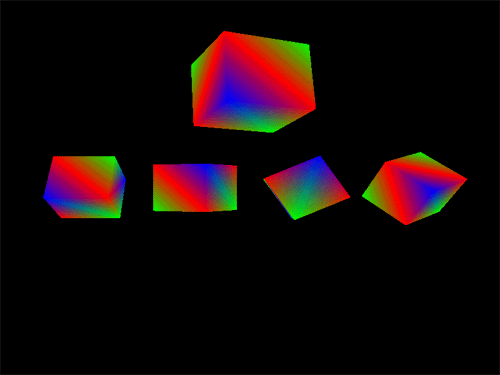## Summary

In this tutorial we learnt what a matrix is, how matrices work and how to multiply them together. We also saw how to use transformation matrices in our DirectX applications. In the next tutorial we'll take a look a textures.

# DirectX Tutorial 6: Textures

## Introduction

In this tutorial we will learn about textures, what they are and how to use them. Textures can be used to add realism to your scenes. From our example from the last tutorial, we will add a different texture to each rotating cube, numbering them from 1 to 5 in different colours. You can download the full source code by clicking the "Download Source" link above.

## What is a Texture?

A texture in 3D graphics is a 2D bitmap that can be applied to a polygon (or a number of polygons) to increase realism. For example, lets say that you want to have a brick wall in your scene. You could create a square object in front of the camera and colour it red. Hmm‚Ä¶ that looks more like a red square than a brick wall, what you really want to see are the bricks and maybe a window. You can do this using textures. All you need is an object (your square) and a wall texture. You can create your texture in any art package that will save .bmp files, I use Adobe Photoshop but you can use any software you like, even Microsoft Paint that comes with Windows.

## Texture Size

You can create a texture in any size you like. But to improve efficiency you should (where possible) keep your textures small and square and to a power of 2: 16√ó16, 32√ó32, 64√ó64, 128√ó128, 256√ó256 etc. 256√ó256 is the most efficient size, but only use this size of texture if you need to. Remember: the smaller the texture, the better.

## Texture Coordinates

What are texture coordinates? Well, texture coordinates are used to specify a point on a texture. Because a texture is 2D we only need two values to specify any point: U and V. U is the number of units across (columns) and V is the number of units down (rows). The values of U and V should be between 0 and 1 (you can specify other values to create special effects, more on this later). The top left corner of the texture is (0, 0) and the bottom right corner is (1, 1) in the form (U, V). Fig 6.1 below shows the texture coordinates of nine points of a texture.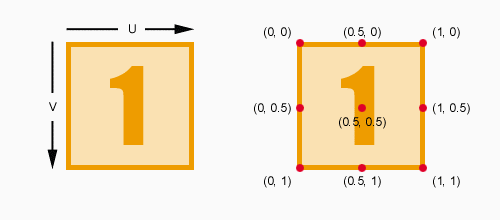Fig 6.1

## Texture Mapping

Now that we know about texture coordinates, we can apply our texture to an object in our scene, this is called Texture Mapping. The process of texture mapping is to map texture coordinates to vertices in a scene, therefore each vertex will have two extra values, U and V. Fig 6.2 below, shows an example of mapping a texture on to a cube. The example uses one texture placed on each side of a cube. The cubes vertices have been numbered in the same way as our cube structure in http://www.andypike.com/tutorials/directx8/003.htm and our code for this tutorial.

Therefore, the texture coordinates for each vertex are as follows (where vertex number = (U, V)):

0 = (0, 1)¬Ý¬Ý¬Ý¬Ý¬Ý¬Ý¬Ý¬Ý 9¬Ý¬Ý = (0, 0)

1 = (0, 0)¬Ý¬Ý¬Ý¬Ý¬Ý¬Ý¬Ý¬Ý 10 = (1, 1)

2 = (1, 1)¬Ý¬Ý¬Ý¬Ý¬Ý¬Ý¬Ý¬Ý 11 = (1, 0)

3 = (1, 0)¬Ý¬Ý¬Ý¬Ý¬Ý¬Ý¬Ý¬Ý 12 = (0, 1)

4 = (0, 1)¬Ý¬Ý¬Ý¬Ý¬Ý¬Ý¬Ý¬Ý 13 = (0, 0)

5 = (0, 0)¬Ý¬Ý¬Ý¬Ý¬Ý¬Ý¬Ý¬Ý 14 = (0, 1)

6 = (1, 1)¬Ý¬Ý¬Ý¬Ý¬Ý¬Ý¬Ý¬Ý 15 = (0, 0)

7 = (1, 0)¬Ý¬Ý¬Ý¬Ý¬Ý¬Ý¬Ý¬Ý 16 = (1, 1)

8 = (0, 1) ¬Ý¬Ý¬Ý¬Ý¬Ý¬Ý¬Ý 17 = (1, 0)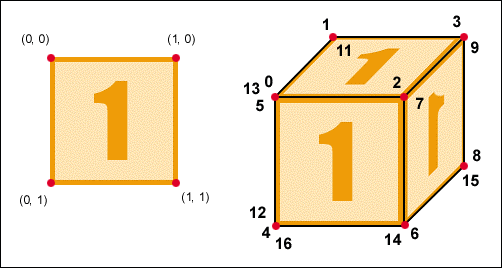Fig 6.2

## Using Textures With DirectX

Step 1: Modify FVF and Custom Vertex

This first thing that we need to do is change our custom vertex structure to hold the texture coordinates U and V, these are the two new FLOAT values tu and tv. We also need to modify our FVF to match by adding the D3DFVF_TEX1 flag.

`//Define a FVF for our cuboids`

`#define CUBIOD_D3DFVF_CUSTOMVERTEX (D3DFVF_XYZ|D3DFVF_DIFFUSE|D3DFVF_TEX1)`

`//Define a custom vertex for our cuboids`

`struct CUBIOD_CUSTOMVERTEX {`

`¬ÝFLOAT x, y, z;`

`¬ÝDWORD colour;`

`¬ÝFLOAT tu, tv;`

`};`

Step 2: Load Texture

We need to add a new member variable to our CCuboid class to hold a pointer to the texture once it is loaded. The new member is called m_pTexture and is of type LPDIRECT3DTEXTURE8. We then need a new method to set the texture by passing in a filename, remember that the file needs to be a .bmp.

`LPDIRECT3DTEXTURE8 m_pTexture;`

`bool CCuboid::SetTexture(const char *szTextureFilePath) {`

`¬Ýif (FAILED(D3DXCreateTextureFromFile(m_pD3DDevice, szTextureFilePath, &m_pTexture))) {`

`¬Ý return false;`

`¬Ý}`

`¬Ýreturn true;`

`}`

Step 3: Set Texture Coordinates

In our UpdateVertices method of CCudoid, we need to set the texture coordinates for each vertex. Notice that after the colour value for each vertex we have added two new values, these are the U and V values of our texture coordinates. These texture coordinates match the values from Fig 6.2.

`bool CCuboid::UpdateVertices() {`

`¬ÝVOID* pVertices;`

`¬Ý//Store each point of the cube together with it's colour and texture coordinates`

`¬Ý//Make sure that the points of a polygon are specified in a clockwise direction,`

`¬Ý//this is because anti-clockwise faces will be culled`

`¬Ý//We will use a three triangle strips to render these polygons (Top, Sides, Bottom).`

`¬ÝCUBIOD_CUSTOMVERTEX cvVertices[] = {`

`¬Ý //Top Face`

`¬Ý {m_rX ‚Äì (m_rWidth/2), m_rY + (m_rHeight/2), m_rZ ‚Äì (m_rDepth/2), D3DCOLOR_XRGB(0, 0, 255), 0.0f, 1.0f,}, //Vertex 0 ‚Äì Blue`

`¬Ý¬Ý{m_rX ‚Äì (m_rWidth/2), m_rY + (m_rHeight/2), m_rZ + (m_rDepth/2), D3DCOLOR_XRGB(255, 0, 0), 0.0f, 0.0f,}, //Vertex 1 ‚Äì Red`

`¬Ý {m_rX + (m_rWidth/2), m_rY + (m_rHeight/2), m_rZ ‚Äì (m_rDepth/2), D3DCOLOR_XRGB(255, 0, 0), 1.0f, 1.0f,}, //Vertex 2 ‚Äì Red`

`¬Ý {m_rX + (m_rWidth/2), m_rY + (m_rHeight/2), m_rZ + (m_rDepth/2), D3DCOLOR_XRGB(0, 255, 0), 1.0f, 0.0f,}, //Vertex 3 ‚Äì Green`

`¬Ý //Face 1`

`¬Ý {m_rX ‚Äì (m_rWidth/2), m_rY ‚Äì (m_rHeight/2), m_rZ ‚Äì (m_rDepth/2), D3DCOLOR_XRGB(255, 0, 0), 0.0f, 1.0f,}, //Vertex 4 ‚Äì Red`

`¬Ý {m_rX ‚Äì (m_rWidth/2), m_rY + (m_rHeight/2), m_rZ ‚Äì (m_rDepth/2), D3DCOLOR_XRGB(0, 0, 255), 0.0f, 0.0f,}, //Vertex 5 ‚Äì Blue`

`¬Ý {m_rX + (m_rWidth/2), m_rY ‚Äì (m_rHeight/2), m_rZ ‚Äì (m_rDepth/2), D3DCOLOR_XRGB(0, 255, 0), 1.0f, 1.0f,}, //Vertex 6 ‚Äì Green`

`¬Ý {m_rX + (m_rWidth/2), m_rY + (m_rHeight/2), m_rZ ‚Äì (m_rDepth/2), D3DCOLOR_XRGB(255, 0, 0), 1.0f, 0.0f,}, //Vertex 7 ‚Äì Red`

`¬Ý //Face 2`

`¬Ý {m_rX + (m_rWidth/2), m_rY ‚Äì (m_rHeight/2), m_rZ + (m_rDepth/2), D3DCOLOR_XRGB(0, 0, 255), 0.0f, 1.0f,}, //Vertex 8 ‚Äì Blue`

`¬Ý {m_rX + (m_rWidth/2), m_rY + (m_rHeight/2), m_rZ + (m_rDepth/2), D3DCOLOR_XRGB(0, 255, 0), 0.0f, 0.0f,}, //Vertex 9 ‚Äì Green`

`¬Ý //Face 3`

`¬Ý {m_rX ‚Äì (m_rWidth/2), m_rY ‚Äì (m_rHeight/2), m_rZ + (m_rDepth/2), D3DCOLOR_XRGB(0, 255, 0), 1.0f, 1.0f,}, //Vertex 10 ‚Äì Green`

`¬Ý {m_rX ‚Äì (m_rWidth/2), m_rY + (m_rHeight/2), m_rZ + (m_rDepth/2), D3DCOLOR_XRGB(255, 0, 0), 1.0f, 0.0f,}, //Vertex 11 ‚Äì Red`

`¬Ý //Face 4`

`¬Ý {m_rX ‚Äì (m_rWidth/2), m_rY ‚Äì (m_rHeight/2), m_rZ ‚Äì (m_rDepth/2), D3DCOLOR_XRGB(255, 0, 0), 0.0f, 1.0f,}, //Vertex 12 ‚Äì Red`

`¬Ý {m_rX ‚Äì (m_rWidth/2), m_rY + (m_rHeight/2), m_rZ ‚Äì (m_rDepth/2), D3DCOLOR_XRGB(0, 0, 255), 0.0f, 0.0f,}, //Vertex 13 ‚Äì Blue`

`¬Ý //Bottom Face`

`¬Ý {m_rX + (m_rWidth/2), m_rY ‚Äì (m_rHeight/2), m_rZ ‚Äì (m_rDepth/2), D3DCOLOR_XRGB(0, 255, 0), 0.0f, 1.0f,}, //Vertex 14 ‚Äì Green`

`¬Ý {m_rX + (m_rWidth/2), m_rY ‚Äì (m_rHeight/2), m_rZ + (m_rDepth/2), D3DCOLOR_XRGB(0, 0, 255), 0.0f, 0.0f,}, //Vertex 15 ‚Äì Blue`

`¬Ý {m_rX ‚Äì (m_rWidth/2), m_rY ‚Äì (m_rHeight/2), m_rZ ‚Äì (m_rDepth/2), D3DCOLOR_XRGB(255, 0, 0), 1.0f, 1.0f,}, //Vertex 16 ‚Äì Red`

`¬Ý {m_rX ‚Äì (m_rWidth/2), m_rY ‚Äì (m_rHeight/2), m_rZ + (m_rDepth/2), D3DCOLOR_XRGB(0, 255, 0), 1.0f, 0.0f,}, //Vertex 17 ‚Äì Green`

`¬Ý};`

`¬Ý//Get a pointer to the vertex buffer vertices and lock the vertex buffer`

`¬Ýif (FAILED(m_pVertexBuffer->Lock(0, sizeof(cvVertices), (BYTE**)&pVertices, 0))) {`

`¬Ý return false;`

`¬Ý}`

`¬Ý//Copy our stored vertices values into the vertex buffer`

`¬Ýmemcpy(pVertices, cvVertices, sizeof(cvVertices));`

`¬Ý//Unlock the vertex buffer`

`¬Ým_pVertexBuffer->Unlock();`

`¬Ýreturn true;`

`}`

Step 4: Rendering

The final code change is in the Render method of CCuboid. We need to set the texture that we want to render using SetTexture. Then we need to set how the texture should be rendered by using the SetTextureStageState method of our device. We have selected that our texture should be rendered without any blending or similar effects. You can blend your texture with other textures or the colour of the vertices depending on which flags to select.

`//Set how the texture should be rendered.`

`if (m_pTexture != NULL) {`

`¬Ý//A texture has been set. We don't want to blend our texture with`

`¬Ý//the colours of our vertices, so use D3DTOP_SELECTARG1`

`¬Ým_pD3DDevice->SetTexture(0, m_pTexture);`

`¬Ým_pD3DDevice->SetTextureStageState(0, D3DTSS_COLOROP, D3DTOP_SELECTARG1);`

`} else {`

`¬Ý//No texture has been set. So we will disable texture rendering.`

`¬Ým_pD3DDevice->SetTextureStageState(0, D3DTSS_COLOROP, D3DTOP_DISABLE);`

`}`

Once you have made these changes, you should finish up with five rotating cubes, each with a different texture (shown below).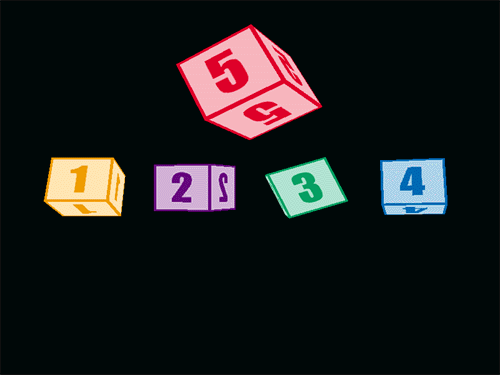## Summary

In this tutorial we've learnt all about textures. There is more to textures in DirectX than that, and we'll take a look at these topics in a future tutorial. In the next tutorial, we'll look into lighting.

# DirectX Tutorial 7: Lighting and Materials

## Introduction

In this tutorial we will learn about lighting and materials in DirectX. We'll create four cubes and place them around the origin (based on the last tutorial) then rotate them. We'll place a light source in the middle of these cubes, near the origin. You will now be able to see how lighting affects your objects in 3D space. You can download the full source code by clicking the "Download Source" link above.

## DirectX Lighting vs. Real World Lighting

In DirectX you can create different types of lights that will make your scene seem more realistic. But the lighting model that DirectX uses is only an approximation of light in the real world. In the real world, light is emitted from a source like a light bulb or torch and travels in a straight line until it fades out or enters your eye. As light travels, it can hit objects and be reflected in a different direction. When it is reflected, the object may absorb some of the light. In fact, light can be reflected hundreds, thousands or even millions of times before it fades out or reaches your eye. Light is reflected differently by each object depending on the material that it is made of. Shiny materials reflect more of the light than non-shiny materials. The amount of calculations to model this in virtual 3D space is too large for real-time rendering. So DirectX approximates lighting.

## Attributes of a light

For different lights you can specify different attributes. Not all lights use all of the attributes that are listed below:

Position

This is the position in 3D space where the light source is located. This will be a coordinate in 3D space such as (0, 10, 0).

Direction

This is the direction in which light is emitted from the light source. This will be a vector such as (0, ‚Äì1, 0).

This is the maximum distance from the light source that the light will travel. Any objects that are out of range will not receive light from this light source.

Attenuation

This is how light changes over distance. This is normally the rate that light fades out between the light source and lights range. You can specify that light does not fade out or that it gets brighter over distance if you want to.

Diffuse Light

This is the colour of diffuse light that is emitted by the light. Diffuse light is light that has been scattered, but it still has direction as opposed to ambient light that does not.

Ambient Light

This is the colour of ambient light that is emitted by the light. Ambient light is general background light. Ambient light has been scattered so much that it does not have a direction or source and is at the same everywhere in the scene.

Specular Light

This is the colour of specular light that is emitted by the light. Specular light is the opposite of diffuse light. Specular light is not scattered at all, you can use specular light to create highlights on your objects.

## Types of lighting

There are four types of lights that you can create in your scene, each of which have their own behaviours and attributes.

Ambient Light

As well as being an attribute of light, you can create a general ambient light level for the scene that is independent of other lights. You can specify how much ambient light there is and what colour it is. You can define the colour by specifying it's red, green and blue (RGB) values.

Point Light

An example of a point light is a light bulb. It has a position but no direction because light is emitted in all directions equally. It also has colour, range and attenuation attributes that can be set. Fig 7.1 below, shows how light is emitted from a point light.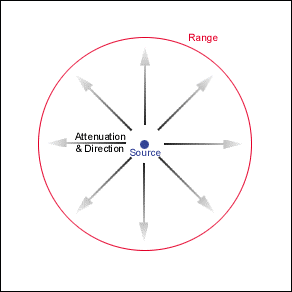Fig 7.1

Directional Light

Directional lights have direction and colour but no position, an example of a directional light would be something like the sun. All objects in your scene will receive the same light from the same direction. Directional lights do not have range or attenuation attributes. Fig 7.2 below, shows how light is emitted from a directional light.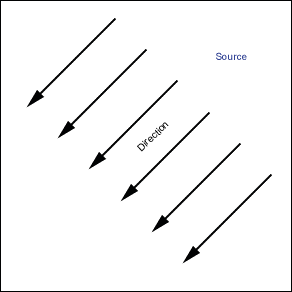Fig 7.2

Spotlight

An example of a spot light would be something like a torch. Spotlights have position, direction, colour, attenuation and range. For a spotlight you can define an inner and outer cone each of which has a light value that is blended between the two. You define the cones by specifying their angle, the inner cone's angle is known as Theta and the outer cone's angle is known as Phi. You define how the illumination between a spotlight's inner and outer cone changes by specifying the lights Falloff property. Fig 7.3 below, shows how light is emitted from a spot light.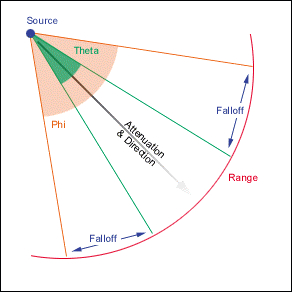Fig 7.3

All lights add a computational overhead to your application, some more than others. The light with the least overhead is ambient light, followed by directional lights, then point lights and finally, the lights with the most overhead are spot lights. Think about this when you are deciding what lights to use in your application.

## Materials

What is a material? Well, a material describes how light is reflected from an object (polygon). You can specify how much light is reflected, this can make the material seem shiny or dull and can give an object colour. There are a number of settings that you can change for a given material, they are listed below:

Diffuse Reflection

This is the amount of diffuse light that the object will reflect. This is a colour value, so you can specify that the object will only reflect red diffuse light. This will make the object look red in colour.

Ambient Reflection

This is the amount of ambient light that the object will reflect. This is a colour value, so you can specify that the object does not reflect ambient light at all. This means that the object will not be seen unless it receives another type of light such as diffuse light.

Specular Reflection and Power

This is the amount of specular light that is reflected. You can use the specular reflection and power settings to create specular highlights which will make the object seem shiny.

Emission

You can also make the object appear to emit light by changing the Emissive property. The object does not acually emit light, therefore other objects in the scene will not be affected by this setting.

## Normals

What is a Normal? Well, the Normal of a polygon is a perpendicular vector from the face of the polygon. The direction of this vector is determined by the order in which the vertices were defined. Fig 7.4 below, shows the normal for a polygon, the vertices have been defined in a clockwise direction. The Normal of a vertex is usually the average of each of the normals of each polygon that shares that vertex. Fig 7.5 below shows the cross section of four polygons and their normals (light red). It also shows the normals for each of the three vertices (red). Normals are used for a number of things but for this tutorial we will use them for light shading.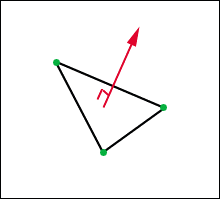Fig 7.4Fig 7.5

## Implementing lights in DirectX

In the code for this tutorial, we will have one point light and we'll set the ambient light level pretty low so that the effects of the point light are more obvious. We will also set the material for our objects to be fairly normal and not too reflective (no specular highlights).

Step 1: Modify FVF and Custom Vertex

The first thing to do is to modify our FVF and custom vertex structure. In the FVF we need to remove the D3DFVF_DIFFUSE flag and replace it with the D3DFVF_NORMAL flag. Then in the custom vertex structure, we need to remove the colour attribute and replace it with a Normal attribute. Notice that the texture attributes and flags are specified last. if you don't specify these values last, you may get some strange effects.

`//Define a FVF for our cuboids`

`#define CUBOID_D3DFVF_CUSTOMVERTEX (D3DFVF_XYZ|D3DFVF_NORMAL|D3DFVF_TEX1)`

`//Define a custom vertex for our cuboids`

`struct CUBOID_CUSTOMVERTEX {`

`¬ÝFLOAT x, y, z; //Position of vertex in 3D space`

`¬ÝFLOAT nx, ny, nz; //Lighting Normal`

`¬ÝFLOAT tu, tv; //Texture coordinates`

`};`

Step 2: Creating the lights

We need to add a new method to CGame called InitialiseLights. We will put all of our light setup code here. First of all, we'll setup a point light for our scene. To do this we need to populate a D3DLIGHT8 structure with the correct values. To specify that this is a point light we need to use the D3DLIGHT_POINT constant. If you want a directional light use D3DLIGHT_DIRECTIONAL or for a spot light use D3DLIGHT_SPOT. Next, we need to set what light our light will emit. So we must specify the Diffuse, Ambient and Specular RGB values. Note that the values for each RGB element should be between 0 and 1, where 0 is none and 1 is full. Next is the position in 3D space, which is a simple x, y, z value. Finally we set the lights range and attenuation. By specifying that this light has an attenuation value of 1 means that the light will not fade over distance.

Now that we have specified our light, we need to add it to our scene and enable it. To do this we must assign it to our device's light list. We do this my using the SetLight method passing in the position in the list as the first parameter. This is a zero based list, so the first position is index 0. Then, to enable the light (turn it on), we use the LightEnable method. The first parameter is the lights index in the light list and the second parameter defines if we should turn the light on or off (TRUE = on, FALSE = off).

We then call SetRenderState to make sure that lighting in general is enabled. Finally, we call SetRenderState again to setup the ambient light level for the whole scene.

`D3DLIGHT8 d3dLight;`

`//Initialize the light structure.`

`ZeroMemory(&d3dLight, sizeof(D3DLIGHT8));`

`//Set up a white point light at (0, 0, ‚Äì10).`

`d3dLight.Type = D3DLIGHT_POINT;`

`d3dLight.Diffuse.r = 1.0f;`

`d3dLight.Diffuse.g = 1.0f;`

`d3dLight.Diffuse.b = 1.0f;`

`d3dLight.Ambient.r = 0.0f;`

`d3dLight.Ambient.g = 0.0f;`

`d3dLight.Ambient.b = 0.0f;`

`d3dLight.Specular.r = 0.0f;`

`d3dLight.Specular.g = 0.0f;`

`d3dLight.Specular.b = 0.0f;`

`d3dLight.Position.x = 0.0f;`

`d3dLight.Position.y = 0.0f;`

`d3dLight.Position.z = ‚Äì10.0f;`

`d3dLight.Attenuation0 = 1.0f;`

`d3dLight.Attenuation1 = 0.0f;`

`d3dLight.Attenuation2 = 0.0f;`

`d3dLight.Range = 100.0f;`

`//Assign the point light to our device in poisition (index) 0`

`m_pD3DDevice->SetLight(0, &d3dLight);`

`//Enable our point light in position (index) 0`

`m_pD3DDevice->LightEnable(0, TRUE);`

`//Turn on lighting`

`m_pD3DDevice->SetRenderState(D3DRS_LIGHTING, TRUE);`

`//Set ambient light level`

`m_pD3DDevice->SetRenderState(D3DRS_AMBIENT, D3DCOLOR_XRGB(32, 32, 32));`

Step 3: Setup Material

To setup the materials for our cubes we need to add a new method to CCuboid called SetMaterial which will enable use to have a different material for each cube if we wish. We define a member variable of CCuboid called m_matMaterial which is a structure of type D3DMATERIAL8. We then set values for each attribute of the structure. Set need to define the Diffuse, Ambient and Specular reflection RGBA values followed by the Emissive RGBA value. Make sure that you initialise all of these value (especially Emissive) otherwise you may find that your lighting does not work correctly.

`bool CCuboid::SetMaterial(D3DCOLORVALUE rgbaDiffuse, D3DCOLORVALUE rgbaAmbient, D3DCOLORVALUE rgbaSpecular, D3DCOLORVALUE rgbaEmissive, float rPower) {`

`¬Ý//Set the RGBA for diffuse light reflected from this material.`

`¬Ým_matMaterial.Diffuse = rgbaDiffuse;`

`¬Ý//Set the RGBA for ambient light reflected from this material.`

`¬Ým_matMaterial.Ambient = rgbaAmbient;`

`¬Ý//Set the color and sharpness of specular highlights for the material.`

`¬Ým_matMaterial.Specular = rgbaSpecular;`

`¬Ým_matMaterial.Power = rPower;`

`¬Ý//Set the RGBA for light emitted from this material.`

`¬Ým_matMaterial.Emissive = rgbaEmissive;`

`¬Ýreturn true;`

`}`

In the CCuboid constructor, we call SetMaterial to give the cube a default material, as shown below.

`//Set material default values (R, G, B, A)`

`D3DCOLORVALUE rgbaDiffuse = {1.0, 1.0, 1.0, 0.0,};`

`D3DCOLORVALUE rgbaAmbient = {1.0, 1.0, 1.0, 0.0,};`

`D3DCOLORVALUE rgbaSpecular = {0.0, 0.0, 0.0, 0.0,};`

`D3DCOLORVALUE rgbaEmissive = {0.0, 0.0, 0.0, 0.0,};`

`SetMaterial(rgbaDiffuse, rgbaAmbient, rgbaSpecular, rgbaEmissive, 0);`

Finally, in our CCuboid's Render method, we need to use the SetMaterial method to tell DirectX that we want to use our material for all future vertices.

`m _pD3DDevice->SetMaterial(&m_matMaterial);`

Step 4: Generate Normals

We've changed the way that the cube is made up. Rather than three triangle strips, we are now using a triangle list of 12 triangles which is 36 vertices! So we define our vertices as before, except this time we remove the colour component and replace it with a Normal vector initialised to zero. We then loop around each triangle and calculate what the Normal vector should be for that triangle using the GetTriangeNormal method. We will then set each of the three vertices normals for that triangle to be the same as the triangle polygon Normal itself. The two code snippets below show the GetTriangeNormal method and the triangle looping.

We set the vertices to be that same as the polygon because we are rendering a shape with sharp edges. We only really need to average the normals of shared vertices if we are drawing a smooth shape like a sphere.

`D3DVECTOR CCuboid::GetTriangeNormal(D3DXVECTOR3* vVertex1, D3DXVECTOR3* vVertex2, D3DXVECTOR3* vVertex3) {`

`¬ÝD3DXVECTOR3 vNormal;`

`¬ÝD3DXVECTOR3 v1;`

`¬ÝD3DXVECTOR3 v2;`

`¬ÝD3DXVec3Subtract(&v1, vVertex2, vVertex1);`

`¬ÝD3DXVec3Subtract(&v2, vVertex3, vVertex1);`

`¬ÝD3DXVec3Cross(&vNormal, &v1, &v2);`

`¬ÝD3DXVec3Normalize(&vNormal, &vNormal);`

`¬Ýreturn vNormal;`

`}`

`//Set all vertex normals`

`int i;`

`for (i = 0; i < 36; i += 3) {`

`¬ÝvNormal = GetTriangeNormal(&D3DXVECTOR3(cvVertices[i].x, cvVertices[i].y, cvVertices[i].z),¬Ý¬Ý&D3DXVECTOR3(cvVertices[i + 1].x, cvVertices[i + 1].y, cvVertices[i + 1].z), &D3DXVECTOR3(cvVertices[i + 2].x, cvVertices[i + 2].y, cvVertices[i + 2].z));`

`¬ÝcvVertices[i].nx = vNormal.x;`

`¬ÝcvVertices[i].ny = vNormal.y;`

`¬ÝcvVertices[i].nz = vNormal.z;`

`¬ÝcvVertices[i + 1].nx = vNormal.x;`

`¬ÝcvVertices[i + 1].ny = vNormal.y;`

`¬ÝcvVertices[i + 1].nz = vNormal.z;`

`¬ÝcvVertices[i + 2].nx = vNormal.x;`

`¬ÝcvVertices[i + 2].ny = vNormal.y;`

`¬ÝcvVertices[i + 2].nz = vNormal.z;`

`}`

Fig 7.6 below shows the Normals for three of the faces of the cube. The Normals for each of the other vertices will also be the same as the faces. If we were to average the Normals, the cube would appear to have rounded edges. We should only average the Normals of shared vertices if we are rendering a smooth object. I'll show you how to average Normals into one in a future tutorial.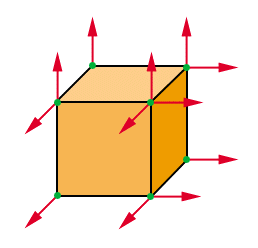Fig 7.6

Finally, in our CCuboid's Render method, we need to change how we render our cube by using D3DPT_TRIANGLELIST rather than D3DPT_TRIANGLESTRIP.

`m_pD3DDevice->DrawPrimitive(D3DPT_TRIANGLELIST, 0, 12);`

Once you have made these changes, you should finish up with four rotating cubes, each with a different texture (shown below). There is a light source in the center of the four cubes, so as they rotate, you can see that some faces are lit and some are in shadow.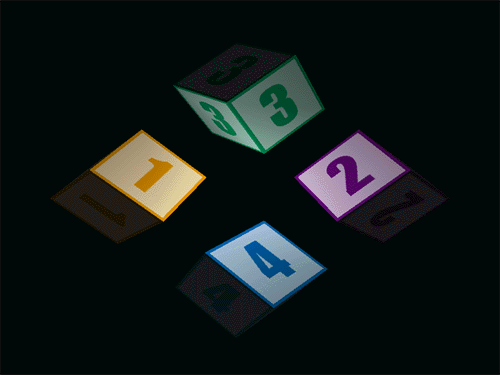## Summary

In this tutorial we've covered a lot of stuff. We've learnt all about the different types of lights and their properties. We've seen how materials can affect how an objects looks once rendered. In the next tutorial we'll take a look at Index Buffers.

# DirectX Tutorial 8: Index Buffers

## Introduction

In this tutorial we will look at Index Buffers. First of all, we'll improve our CCuboid class to use index buffers. Then we'll create a new class called CTerrain, which we can use to generate a very simple terrain. Once the terrain has been created, we'll add a grass texture to make it seem more realistic. You can download the full source code by clicking the "Download Source" link above.

## What is an Index Buffer?

An index buffer is a memory buffer that holds indices that "point" to vertices in your vertex buffer. When a scene is rendered, DirectX performs certain calculations on each vertex such as lighting and transformations. What we want to do is minimise the amount of calculations that DirectX has to do, therefore, we need to minimise the number of vertices. We can do this using an index buffer. Lets say you want to draw a square. Your square will be made up from two triangles, which is six vertices (using a triangle list). But we only really need four vertices to define a square (one for each corner). We had to use six vertices because two of them are shared (have the same value). Because we have shared vertices, it's a good idea to use an index buffer. Here's how it works: we define the four corners of our square as vertices in our vertex buffer. Then we define six indices in our index buffer each of which "point" to a vertex in the vertex buffer. We then render our triangles from the indices in the index buffer and so, only use four vertices. Fig 8.1 below shows this example.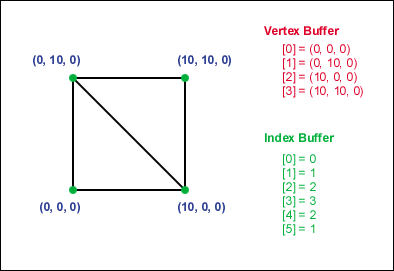Fig 8.1

In the example above, we have only used four vertices to describe our square, which is a saving of two vertices. The index buffer contains six elements, each of these elements contains an index to a vertex in the vertex buffer. Notice that the order in the index buffer is in a clockwise direction, this is the order in which vertices will be rendered. We then render our scene as before, except this time we use the index buffer in addition to our vertex buffer. So, if we apply this technique to our cube, we will be saving twelve vertices (24 rather than 36). That doesn't sound that much does it? But imagine a scene that contains 100 cubes, that's a saving of 1200 vertex calculations per frame!

## Implementing an Index Buffer in DirectX

The first thing that we are going to do is add an index buffer to our CCuboid class. To do this, we need to add the following code:

Step 1: Creating the Index Buffer

First of all, create two new member variables: one LPDIRECT3DINDEXBUFFER8 called m_pIndexBuffer and a DWORD called m_dwNumOfIndices. m_pIndexBuffer will be a pointer to our index buffer and m_dwNumOfIndices will be the total number of indices for our object, in this case 36 (6 faces x 2 triangles per face x 3 vertices per triangle), which will be set in the constructor.

Then we have a new method called CreateIndexBuffer. We'll call this method once at start-up (in the constructor) before we set our vertex values in our vertex buffer. We populate the values in an index buffer in the same way that we populate vertices in a vertex buffer. First we create the index buffer and get a pointer to it (m_pIndexBuffer). We then store the 36 indices in a temporary WORD array ready to be copied into the index buffer. Then we lock the index buffer, copy the stored indices into it and then unlock it.

`LPDIRECT3DINDEXBUFFER8 m_pIndexBuffer;`

`DWORD m_dwNumOfIndices;`

`bool CCuboid::CreateIndexBuffer() {`

`¬ÝVOID* pBufferIndices;`

`¬Ý//Create the index buffer from our device`

`¬Ýif (FAILED(m_pD3DDevice->CreateIndexBuffer(m_dwNumOfIndices * sizeof(WORD), 0, D3DFMT_INDEX16, D3DPOOL_MANAGED, &m_pIndexBuffer))) {`

`¬Ý return false;`

`¬Ý}`

`¬Ý//Set values for the index buffer`

`¬ÝWORD pIndices[] = {`

`¬Ý 0, 1, 2, 3, 2, 1, //Top`

`¬Ý 4, 5, 6, 7, 6, 5, //Face 1`

`¬Ý 8, 9,10,11,10, 9, //Face 2`

`¬Ý 12,13,14,15,14,13, //Face 3`

`¬Ý 16,17,18,19,18,17, //Face 4`

`¬Ý 20,21,22,23,22,21}; //Bottom`

`¬Ý//Get a pointer to the index buffer indices and lock the index buffer`

`¬Ým_pIndexBuffer->Lock(0, m_dwNumOfIndices * sizeof(WORD), (BYTE**)&pBufferIndices, 0);`

`¬Ý//Copy our stored indices values into the index buffer`

`¬Ýmemcpy(pBufferIndices, pIndices, m_dwNumOfIndices * sizeof(WORD));`

`¬Ý//Unlock the index buffer`

`¬Ým_pIndexBuffer->Unlock();`

`¬Ýreturn true;`

`}`

Step 2: Modify the Vertex Buffer

In our UpdateVertices method, we need to make a few changes. First, and most importantly, notice that we are only defining 24 vertices in our cvVertices array rather than 36. As in the last tutorial, we initialise our vertex normals to zero.

The other difference in this tutorial is that we will calculate the average normal for shared vertices. We don't really need to do this for a cube's shared vertices, but we will as a demonstration of how to do it. To do this, we need to new arrays: pNumOfSharedPolygons and pSumVertexNormal. These arrays are used in parallel, pNumOfSharedPolygons will keep a count of how many times a given vertex is used (shared) and pSumVertexNormal will add together each triangle normal for faces that share a given vertex. We'll then use these arrays to calculate the average normal for each vertex.

Once we have defined our vertices, we loop through the indices in our index buffer. So, for each triangle we calculate its face normal, increment the vertices count for each of the three vertices and add the face normal to the vertices normal array.

Then we loop through each vertex, and calculate it's average normal by dividing the sum of face normals by the number of times it has been used. We then normalize this value to ensure that the x, y and z parts of the normal vector are between 0 and 1. Finally, we apply the average normal to each vertex and copy the vertices into our vertex buffer as before.

`bool CCuboid::UpdateVertices() {`

`¬ÝDWORD i;`

`¬ÝVOID* pVertices;`

`¬ÝWORD* pBufferIndices;`

`¬ÝD3DXVECTOR3 vNormal;`

`¬ÝDWORD dwVertex1;`

`¬ÝDWORD dwVertex2;`

`¬ÝDWORD dwVertex3;`

`¬ÝWORD* pNumOfSharedPolygons = new WORD[m_dwNumOfVertices]; //Array holds how many times this vertex is shared`

`¬ÝD3DVECTOR* pSumVertexNormal = new D3DVECTOR[m_dwNumOfVertices]; //Array holds sum of all face normals for shared vertex`

`¬Ý//Clear memory`

`¬Ýfor (i = 0; i < m_dwNumOfVertices; i++) {`

`¬Ý pNumOfSharedPolygons[i] = 0;`

`¬Ý pSumVertexNormal[i] = D3DXVECTOR3(0,0,0);`

`¬Ý}`

`¬ÝCUBOID_CUSTOMVERTEX cvVertices[] = {`

`¬Ý //Top Face`

`¬Ý {m_rX ‚Äì (m_rWidth / 2), m_rY + (m_rHeight / 2), m_rZ ‚Äì (m_rDepth / 2), 0.0f, 0.0f, 0.0f, 0.0f, 1.0f,}, //Vertex 0`

`¬Ý {m_rX ‚Äì (m_rWidth / 2), m_rY + (m_rHeight / 2), m_rZ + (m_rDepth / 2), 0.0f, 0.0f, 0.0f, 0.0f, 0.0f,}, //Vertex 1`

`¬Ý {m_rX + (m_rWidth / 2), m_rY + (m_rHeight / 2), m_rZ ‚Äì (m_rDepth / 2), 0.0f, 0.0f, 0.0f, 1.0f, 1.0f,}, //Vertex 2`

`¬Ý {m_rX + (m_rWidth / 2), m_rY + (m_rHeight / 2), m_rZ + (m_rDepth / 2), 0.0f, 0.0f, 0.0f, 1.0f, 0.0f,}, //Vertex 3`

`¬Ý //Face 1`

`¬Ý {m_rX ‚Äì (m_rWidth / 2), m_rY ‚Äì (m_rHeight / 2), m_rZ ‚Äì (m_rDepth / 2), 0.0f, 0.0f, 0.0f, 0.0f, 1.0f,}, //Vertex 4`

`¬Ý {m_rX ‚Äì (m_rWidth / 2), m_rY + (m_rHeight / 2), m_rZ ‚Äì (m_rDepth / 2), 0.0f, 0.0f, 0.0f, 0.0f, 0.0f,}, //Vertex 5`

`¬Ý {m_rX + (m_rWidth / 2), m_rY ‚Äì (m_rHeight / 2), m_rZ ‚Äì (m_rDepth / 2), 0.0f, 0.0f, 0.0f, 1.0f, 1.0f,}, //Vertex 6`

`¬Ý {m_rX + (m_rWidth / 2), m_rY + (m_rHeight / 2), m_rZ ‚Äì (m_rDepth / 2), 0.0f, 0.0f, 0.0f, 1.0f, 0.0f,}, //Vertex 7`

`¬Ý //Face 2`

`¬Ý {m_rX + (m_rWidth / 2), m_rY ‚Äì (m_rHeight / 2), m_rZ ‚Äì (m_rDepth / 2), 0.0f, 0.0f, 0.0f, 0.0f, 1.0f,}, //Vertex 8`

`¬Ý {m_rX + (m_rWidth / 2), m_rY + (m_rHeight / 2), m_rZ ‚Äì (m_rDepth / 2), 0.0f, 0.0f, 0.0f, 0.0f, 0.0f,}, //Vertex 9`

`¬Ý {m_rX + (m_rWidth / 2), m_rY ‚Äì (m_rHeight / 2), m_rZ + (m_rDepth / 2), 0.0f, 0.0f, 0.0f, 1.0f, 1.0f,}, //Vertex 10`

`¬Ý {m_rX + (m_rWidth / 2), m_rY + (m_rHeight / 2), m_rZ + (m_rDepth / 2), 0.0f, 0.0f, 0.0f, 1.0f, 0.0f,}, //Vertex 11`

`¬Ý //Face 3`

`¬Ý {m_rX + (m_rWidth / 2), m_rY ‚Äì (m_rHeight / 2), m_rZ + (m_rDepth / 2), 0.0f, 0.0f, 0.0f, 0.0f, 1.0f,}, //Vertex 12`

`¬Ý {m_rX + (m_rWidth / 2), m_rY + (m_rHeight / 2), m_rZ + (m_rDepth / 2), 0.0f, 0.0f, 0.0f, 0.0f, 0.0f,}, //Vertex 13`

`¬Ý {m_rX ‚Äì (m_rWidth / 2), m_rY ‚Äì (m_rHeight / 2), m_rZ + (m_rDepth / 2), 0.0f, 0.0f, 0.0f, 1.0f, 1.0f,}, //Vertex 14`

`¬Ý {m_rX ‚Äì (m_rWidth / 2), m_rY + (m_rHeight / 2), m_rZ + (m_rDepth / 2), 0.0f, 0.0f, 0.0f, 1.0f, 0.0f,}, //Vertex 15`

`¬Ý //Face 4`

`¬Ý {m_rX ‚Äì (m_rWidth / 2), m_rY ‚Äì (m_rHeight / 2), m_rZ + (m_rDepth / 2), 0.0f, 0.0f, 0.0f, 0.0f, 1.0f,}, //Vertex 16`

`¬Ý {m_rX ‚Äì (m_rWidth / 2), m_rY + (m_rHeight / 2), m_rZ + (m_rDepth / 2), 0.0f, 0.0f, 0.0f, 0.0f, 0.0f,}, //Vertex 17`

`¬Ý {m_rX ‚Äì (m_rWidth / 2), m_rY ‚Äì (m_rHeight / 2), m_rZ ‚Äì (m_rDepth / 2), 0.0f, 0.0f, 0.0f, 1.0f, 1.0f,}, //Vertex 18`

`¬Ý {m_rX ‚Äì (m_rWidth / 2), m_rY + (m_rHeight / 2), m_rZ ‚Äì (m_rDepth / 2), 0.0f, 0.0f, 0.0f, 1.0f, 0.0f,}, //Vertex 19`

`¬Ý //Bottom Face`

`¬Ý {m_rX + (m_rWidth / 2), m_rY ‚Äì (m_rHeight / 2), m_rZ ‚Äì (m_rDepth / 2), 0.0f, 0.0f, 0.0f, 0.0f, 1.0f,}, //Vertex 20`

`¬Ý {m_rX + (m_rWidth / 2), m_rY ‚Äì (m_rHeight / 2), m_rZ + (m_rDepth / 2), 0.0f, 0.0f, 0.0f, 0.0f, 0.0f,}, //Vertex 21`

`¬Ý {m_rX ‚Äì (m_rWidth / 2), m_rY ‚Äì (m_rHeight / 2), m_rZ ‚Äì (m_rDepth / 2), 0.0f, 0.0f, 0.0f, 1.0f, 1.0f,}, //Vertex 22`

`¬Ý {m_rX ‚Äì (m_rWidth / 2), m_rY ‚Äì (m_rHeight / 2), m_rZ + (m_rDepth / 2), 0.0f, 0.0f, 0.0f, 1.0f, 0.0f,}, //Vertex 23`

`¬Ý};`

`¬Ý//Get a pointer to the index buffer indices and lock the index buffer`

`¬Ým_pIndexBuffer->Lock(0, m_dwNumOfIndices * sizeof(WORD), (BYTE**)&pBufferIndices, D3DLOCK_READONLY);`

`¬Ý//For each triangle, count the number of times each vertex is used and`

`¬Ý//add together the normals of faces that share a vertex`

`¬Ýfor (i = 0; i < m_dwNumOfIndices; i += 3) {`

`¬Ý dwVertex1 = pBufferIndices[i];`

`¬Ý dwVertex2 = pBufferIndices[i + 1];`

`¬Ý dwVertex3 = pBufferIndices[i + 2];`

`¬Ý vNormal = GetTriangeNormal(&D3DXVECTOR3(cvVertices[dwVertex1].x, cvVertices[dwVertex1].y, cvVertices[dwVertex1].z), &D3DXVECTOR3(cvVertices[dwVertex2].x, cvVertices[dwVertex2].y, cvVertices[dwVertex2].z), &D3DXVECTOR3(cvVertices[dwVertex3].x, cvVertices[dwVertex3].y, cvVertices[dwVertex3].z));`

`¬Ý pNumOfSharedPolygons[dwVertex1]++;`

`¬Ý pNumOfSharedPolygons[dwVertex2]++;`

`¬Ý pNumOfSharedPolygons[dwVertex3]++;`

`¬Ý pSumVertexNormal[dwVertex1].x += vNormal.x;`

`¬Ý pSumVertexNormal[dwVertex1].y += vNormal.y;`

`¬Ý pSumVertexNormal[dwVertex1].z += vNormal.z;`

`¬Ý pSumVertexNormal[dwVertex2].x += vNormal.x;`

`¬Ý pSumVertexNormal[dwVertex2].y += vNormal.y;`

`¬Ý pSumVertexNormal[dwVertex2].z += vNormal.z;`

`¬Ý pSumVertexNormal[dwVertex3].x += vNormal.x;`

`¬Ý pSumVertexNormal[dwVertex3].y += vNormal.y;`

`¬Ý pSumVertexNormal[dwVertex3].z += vNormal.z;`

`¬Ý}`

`¬Ý//Unlock the index buffer`

`¬Ým_pIndexBuffer->Unlock();`

`¬Ý//For each vertex, calculate and set the average normal`

`¬Ýfor (i = 0; i < m_dwNumOfVertices; i++) {`

`¬Ý vNormal.x = pSumVertexNormal[i].x / pNumOfSharedPolygons[i];`

`¬Ý vNormal.y = pSumVertexNormal[i].y / pNumOfSharedPolygons[i];`

`¬Ý vNormal.z = pSumVertexNormal[i].z / pNumOfSharedPolygons[i];`

`¬Ý D3DXVec3Normalize(&vNormal, &vNormal);`

`¬Ý cvVertices[i].nx = vNormal.x;`

`¬Ý cvVertices[i].ny = vNormal.y;`

`¬Ý cvVertices[i].nz = vNormal.z;`

`¬Ý}`

`¬Ý//Get a pointer to the vertex buffer vertices and lock the vertex buffer`

`¬Ýif (FAILED(m_pVertexBuffer->Lock(0, sizeof(cvVertices), (BYTE**)&pVertices, 0))) {`

`¬Ý return false;`

`¬Ý}`

`¬Ý//Copy our stored vertices values into the vertex buffer`

`¬Ýmemcpy(pVertices, cvVertices, sizeof(cvVertices));`

`¬Ý//Unlock the vertex buffer`

`¬Ým_pVertexBuffer->Unlock();`

`¬Ý//Clean up`

`¬Ýdelete pNumOfSharedPolygons;`

`¬Ýdelete pSumVertexNormal;`

`¬ÝpNumOfSharedPolygons = NULL;`

`¬ÝpSumVertexNormal = NULL;`

`¬Ýreturn true;`

`}`

Step 3: Render

Our render function stays the same apart form two things, first of all, we need to tell DirectX that we want to render our polygons from an index buffer. We do this with a call to SetIndices, passing in the index buffer pointer that we want to use. Also, we need to use the DrawIndexedPrimitive method rather than DrawPrimitive to render our polygons.

`//Select index buffer`

`m_pD3DDevice->SetIndices(m_pIndexBuffer, 0);`

`//Render polygons from index buffer`

`m_pD3DDevice->DrawIndexedPrimitive(D3DPT_TRIANGLELIST, 0, m_dwNumOfVertices, 0, m_dwNumOfPolygons);`

## Creating a Terrain

To create our terrain, we will use the same techniques as we did to create our cube. The terrain will be made up from a 20√ó20 grid of quads (squares made up from 2 triangles). We will use an index buffer, which will reduce the number of vertices from 2400 to 441. I'm not going to post the code here because it is very similar to the code above. The only real differences are the way the index and vertex buffers are populated. You can download the full source code by clicking the "Download Source" link above. Fig 8.2 below shows our flat terrain grid. You can render your scene in "wireframe" mode with one simple call to SetRenderState which is also shown below.

`//Set fill state. Possible values: D3DFILL_POINT, D3DFILL_WIREFRAME, D3DFILL_SOLID`

`m_pD3DDevice->SetRenderState(D3DRS_FILLMODE, D3DFILL_WIREFRAME);`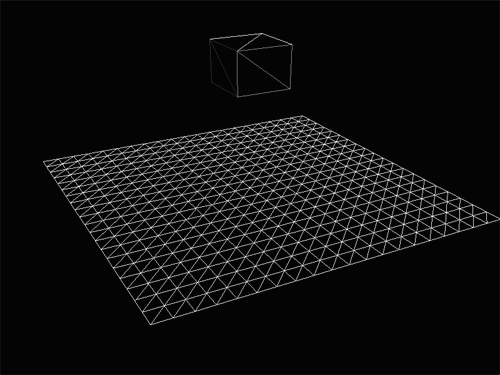Fig 8.2

Now that we have a flat grid, we can turn this into a simple terrain by setting a random value for y for each vertex. I've done this for each vertex in the grid except the ones that are around the edge so that the four edges are all the same level. If you want to create a more detailed terrain, have a look at http://www.gameprogrammer.com/fractal.html where there is a tutorial on how to generate a random fractal terrain. Fig 8.3 below shows the grid with random y values.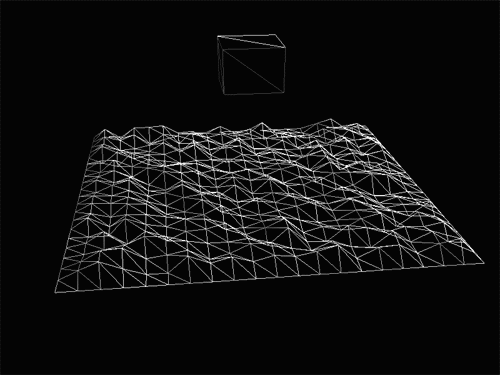Fig 8.3

The last thing to do is apply a grass texture to the terrain and set the render state to solid rather than wireframe. Once you have done this, you should end up with a scene that looks like the screenshot below.## Summary

In this tutorial we've look at index buffers and how they can make a massive saving on how many vertices need to be processed. In the next tutorial we will look at how to create other shapes such as spheres, cylinders and cones.

# DirectX Tutorial 9: Textured Spheres, Cylinders and Cones

## Introduction

In this tutorial we will look at how to create a textured sphere, cylinder and cone. We'll set-up the normals so that the objects are shaded correctly. Also, we'll derive all of our classes from one base class called CBase, which has methods for HTML logging and Normal calculations. Our example application for this tutorial will consist of two cylinders, two cones and a simple model of the Moon rotating around Earth, which is rotating around the Sun. You can download the full source code by clicking the "Download Source" link above.

## How to make a cylinder

Our cylinder will be made up from two triangle fans, one for the top and one for the bottom. The sides of the cylinder will be made up from a triangle strip. Fig 9.1 below shows a diagram of the vertices and polygons for a cylinder. It also includes the normals for the four corners of one segment. To create our cylinder we have not used an index buffer, only a vertex buffer. This is because; although we do have shared vertices (between the sides and the top/bottom faces) we want different normals so the edges around the top and bottom appear sharp.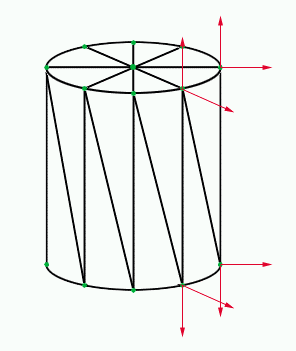Fig 9.1

To create the cylinder we need to specify the number of segments. There are 8 segments in the diagram above. The more segments there are, the smoother and rounder the cylinder will appear. We also need to know the height and radius of the cylinder. Once we know the height, radius and number of segments, we can defined the position of our vertices together with their Normal value and texture coordinates. The following code snippet shows how this is done.

`bool CCylinder::UpdateVertices() {`

`¬ÝCYLINDER_CUSTOMVERTEX* pVertex;`

`¬ÝWORD wVertexIndex = 0;`

`¬Ýint nCurrentSegment;`

`¬Ý//Lock the vertex buffer`

`¬Ýif (FAILED(m_pVertexBuffer->Lock(0, 0, (BYTE**)&pVertex, 0))) {`

`¬Ý LogError("<li>CCylinder: Unable to lock vertex buffer.");`

`¬Ý return false;`

`¬Ý}`

`¬Ýfloat rDeltaSegAngle = (2.0f * D3DX_PI / m_nSegments);`

`¬Ýfloat rSegmentLength = 1.0f / (float)m_nSegments;`

`¬Ý//Create the sides triangle strip`

`¬Ýfor (nCurrentSegment = 0; nCurrentSegment <= m_nSegments; nCurrentSegment++) {`

`¬Ý float x0 = m_rRadius * sinf(nCurrentSegment * rDeltaSegAngle);`

`¬Ý float z0 = m_rRadius * cosf(nCurrentSegment * rDeltaSegAngle);`

`¬Ý pVertex->x = x0;`

`¬Ý pVertex->y = 0.0f + (m_rHeight / 2.0f);`

`¬Ý pVertex->z = z0;`

`¬Ý pVertex->nx = x0;`

`¬Ý pVertex->ny = 0.0f;`

`¬Ý pVertex->nz = z0;`

`¬Ý pVertex->tu = 1.0f ‚Äì (rSegmentLength * (float)nCurrentSegment);`

`¬Ý pVertex->tv = 0.0f;`

`¬Ý pVertex++;`

`¬Ý pVertex->x = x0;`

`¬Ý pVertex->y = 0.0f ‚Äì (m_rHeight / 2.0f);`

`¬Ý pVertex->z = z0;`

`¬Ý pVertex->nx = x0;`

`¬Ý pVertex->ny = 0.0f;`

`¬Ý pVertex->nz = z0;`

`¬Ý pVertex->tu = 1.0f ‚Äì (rSegmentLength * (float)nCurrentSegment);`

`¬Ý pVertex->tv = 1.0f;`

`¬Ý pVertex++;`

`¬Ý}`

`¬Ý//Create the top triangle fan: Center`

`¬ÝpVertex->x = 0.0f;`

`¬ÝpVertex->y = 0.0f + (m_rHeight / 2.0f);`

`¬ÝpVertex->z = 0.0f;`

`¬ÝpVertex->nx = 0.0f;`

`¬ÝpVertex->ny = 1.0f;`

`¬ÝpVertex->nz = 0.0f;`

`¬ÝpVertex->tu = 0.5f;`

`¬ÝpVertex->tv = 0.5f;`

`¬ÝpVertex++;`

`¬Ý//Create the top triangle fan: Edges`

`¬Ýfor (nCurrentSegment = 0; nCurrentSegment <= m_nSegments; nCurrentSegment++) {`

`¬Ý float x0 = m_rRadius * sinf(nCurrentSegment * rDeltaSegAngle);`

`¬Ý float z0 = m_rRadius * cosf(nCurrentSegment * rDeltaSegAngle);`

`¬Ý pVertex->x = x0;`

`¬Ý pVertex->y = 0.0f + (m_rHeight / 2.0f);`

`¬Ý pVertex->z = z0;`

`¬Ý pVertex->nx = 0.0f;`

`¬Ý pVertex->ny = 1.0f;`

`¬Ý pVertex->nz = 0.0f;`

`¬Ý float tu0 = (0.5f * sinf(nCurrentSegment * rDeltaSegAngle)) + 0.5f;`

`¬Ý float tv0 = (0.5f * cosf(nCurrentSegment * rDeltaSegAngle)) + 0.5f;`

`¬Ý pVertex->tu = tu0;`

`¬Ý pVertex->tv = tv0;`

`¬Ý pVertex++;`

`¬Ý}`

`¬Ý//Create the bottom triangle fan: Center`

`¬ÝpVertex->x = 0.0f;`

`¬ÝpVertex->y = 0.0f ‚Äì (m_rHeight / 2.0f);`

`¬ÝpVertex->z = 0.0f;`

`¬ÝpVertex->nx = 0.0f;`

`¬ÝpVertex->ny = ‚Äì1.0f;`

`¬ÝpVertex->nz = 0.0f;`

`¬ÝpVertex->tu = 0.5f;`

`¬ÝpVertex->tv = 0.5f;`

`¬ÝpVertex++;`

`¬Ý//Create the bottom triangle fan: Edges`

`¬Ýfor (nCurrentSegment = m_nSegments; nCurrentSegment >= 0; nCurrentSegment--) {`

`¬Ý float x0 = m_rRadius * sinf(nCurrentSegment * rDeltaSegAngle);`

`¬Ý float z0 = m_rRadius * cosf(nCurrentSegment * rDeltaSegAngle);`

`¬Ý pVertex->x = x0;`

`¬Ý pVertex->y = 0.0f ‚Äì (m_rHeight / 2.0f);`

`¬Ý pVertex->z = z0;`

`¬Ý pVertex->nx = 0.0f;`

`¬Ý pVertex->ny = ‚Äì1.0f;`

`¬Ý pVertex->nz = 0.0f;`

`¬Ý float tu0 = (0.5f * sinf(nCurrentSegment * rDeltaSegAngle)) + 0.5f;`

`¬Ý float tv0 = (0.5f * cosf(nCurrentSegment * rDeltaSegAngle)) + 0.5f;`

`¬Ý pVertex->tu = tu0;`

`¬Ý pVertex->tv = tv0;`

`¬Ý pVertex++;`

`¬Ý}`

`¬Ýif (FAILED(m_pVertexBuffer->Unlock())) {`

`¬Ý LogError("<li>CCylinder: Unable to unlock vertex buffer.");`

`¬Ý return false;`

`¬Ý}`

`¬Ýreturn true;`

`}`

So, what's going on here? Well, after locking our vertex buffer (so we can write to it) we calculate the angle for each segment. This is done by dividing the number of radians in a circle (2*PI) by the number of segments, this angle is stored in rDeltaSegAngle for use later when defining the vertices position. We then calculate the length of each segment for use when defining the texture coordinates for the triangle strip (sides). This is done by dividing the length of our texture (1.0) by the number of segments.

Once this is done, we can define the sides of our cylinder. We loop around, once for each segment + 1. In this loop we calculate the x and z position for the current segment and write these values for the top and bottom vertex for that segment. The y value is simply + or ‚Äì half the height. We use the segment length to calculate the texture coordinates for the current vertex.

Once that is done, we can define the two triangle fans, one for the top and one for the bottom. First we define the centre point for the fan and then we use the same calculations to define the edge vertices of the fan. Once this is complete for the top and bottom fans, the vertex buffer is full and then unlocked ready for rendering.

## How to make a cone

Our cone will be made up from one triangle fan for the bottom and a triangle list for the sides. As for the cylinder above, we need to specify the number of segments, the height and the radius for our cone. Fig 9.2 below shows how the cone will be made up; there are 8 segments in this cone. The red arrows show the normals for one segment of this cone. To create the cone, we will use an index buffer and a vertex buffer. We will store the triangles for the sides in the index buffer and use the vertex buffer to store the vertices for the side triangles and the base triangle fan. This is so that we get the correct shading around the cone and a sharp edge between the sides and base.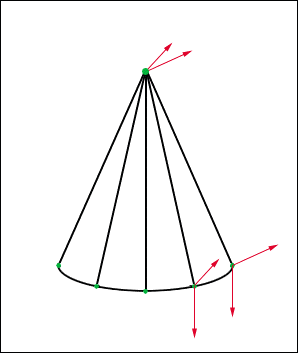Fig 9.2

To create the cone we need to specify the number of segments. There are 8 segments in the diagram above. The more segments there are, the smoother and rounder the cone will appear. We also need to know the height and radius of the cone. Once we know the height, radius and number of segments, we can defined the position of our vertices together with their Normal value and texture coordinates. The following code snippet shows how this is done.

`bool CCone::UpdateVertices() {`

`¬ÝCONE_CUSTOMVERTEX* pVertex;`

`¬ÝWORD* pIndices;`

`¬ÝWORD wVertexIndex = 0;`

`¬Ýint nCurrentSegment;`

`¬Ý//Lock the vertex buffer`

`¬Ýif (FAILED(m_pVertexBuffer->Lock(0, 0, (BYTE**)&pVertex, 0))) {`

`¬Ý LogError("<li>CCone: Unable to lock vertex buffer.");`

`¬Ý return false;`

`¬Ý}`

`¬Ý//Lock the index buffer`

`¬Ýif (FAILED(m_pIndexBuffer->Lock(0, m_dwNumOfIndices, (BYTE**)&pIndices, 0))) {`

`¬Ý LogError("<li>CCone: Unable to lock index buffer.");`

`¬Ý return false;`

`¬Ý}`

`¬Ýfloat rDeltaSegAngle = (2.0f * D3DX_PI / m_nSegments);`

`¬Ýfloat rSegmentLength = 1.0f / (float)m_nSegments;`

`¬Ýfloat ny0 = (90.0f ‚Äì (float)D3DXToDegree(atan(m_rHeight / m_rRadius))) / 90.0f;`

`¬Ý//For each segment, add a triangle to the sides triangle list`

`¬Ýfor (nCurrentSegment = 0; nCurrentSegment < m_nSegments; nCurrentSegment++) {`

`¬Ý float x0 = m_rRadius * sinf(nCurrentSegment * rDeltaSegAngle);`

`¬Ý float z0 = m_rRadius * cosf(nCurrentSegment * rDeltaSegAngle);`

`¬Ý pVertex->x = 0.0f;`

`¬Ý pVertex->y = 0.0f + (m_rHeight / 2.0f);`

`¬Ý pVertex->z = 0.0f;`

`¬Ý pVertex->nx = x0;`

`¬Ý pVertex->ny = ny0;`

`¬Ý pVertex->nz = z0;`

`¬Ý pVertex->tu = 1.0f ‚Äì (rSegmentLength * (float)nCurrentSegment);`

`¬Ý pVertex->tv = 0.0f;`

`¬Ý pVertex++;`

`¬Ý pVertex->x = x0;`

`¬Ý pVertex->y = 0.0f ‚Äì (m_rHeight / 2.0f);`

`¬Ý pVertex->z = z0;`

`¬Ý pVertex->nx = x0;`

`¬Ý pVertex->ny = ny0;`

`¬Ý pVertex->nz = z0;`

`¬Ý pVertex->tu = 1.0f ‚Äì (rSegmentLength * (float)nCurrentSegment);`

`¬Ý pVertex->tv = 1.0f;`

`¬Ý pVertex++;`

`¬Ý //Set three indices (1 triangle) per segment`

`¬Ý *pIndices = wVertexIndex;`

`¬Ý pIndices++;`

`¬Ý wVertexIndex++;`

`¬Ý *pIndices = wVertexIndex;`

`¬Ý pIndices++;`

`¬Ý wVertexIndex += 2;`

`¬Ý if (nCurrentSegment == m_nSegments ‚Äì 1) {`

`¬Ý¬Ý *pIndices = 1;`

`¬Ý¬Ý pIndices++;`

`¬Ý¬Ý wVertexIndex--;`

`¬Ý } else {`

`¬Ý¬Ý *pIndices = wVertexIndex;`

`¬Ý¬Ý pIndices++;`

`¬Ý¬Ý wVertexIndex--;`

`¬Ý }`

`¬Ý}`

`¬Ý//Create the bottom triangle fan: Center vertex`

`¬ÝpVertex->x = 0.0f;`

`¬ÝpVertex->y = 0.0f ‚Äì (m_rHeight / 2.0f);`

`¬ÝpVertex->z = 0.0f;`

`¬ÝpVertex->nx = 0.0f;`

`¬ÝpVertex->ny = ‚Äì1.0f;`

`¬ÝpVertex->nz = 0.0f;`

`¬ÝpVertex->tu = 0.5f;`

`¬ÝpVertex->tv = 0.5f;`

`¬ÝpVertex++;`

`¬Ý//Create the bottom triangle fan: Edge vertices`

`¬Ýfor (nCurrentSegment = m_nSegments; nCurrentSegment >= 0; nCurrentSegment--) {`

`¬Ý float x0 = m_rRadius * sinf(nCurrentSegment * rDeltaSegAngle);`

`¬Ý¬Ýfloat z0 = m_rRadius * cosf(nCurrentSegment * rDeltaSegAngle);`

`¬Ý¬ÝpVertex->x = x0;`

`¬Ý pVertex->y = 0.0f ‚Äì (m_rHeight / 2.0f);`

`¬Ý pVertex->z = z0;`

`¬Ý pVertex->nx = 0.0f;`

`¬Ý pVertex->ny = ‚Äì1.0f;`

`¬Ý pVertex->nz = 0.0f;`

`¬Ý float tu0 = (0.5f * sinf(nCurrentSegment * rDeltaSegAngle)) + 0.5f;`

`¬Ý float tv0 = (0.5f * cosf(nCurrentSegment * rDeltaSegAngle)) + 0.5f;`

`¬Ý pVertex->tu = tu0;`

`¬Ý pVertex->tv = tv0;`

`¬Ý pVertex++;`

`¬Ý}`

`¬Ýif (FAILED(m_pVertexBuffer->Unlock())) {`

`¬Ý LogError("<li>CCone: Unable to unlock vertex buffer.");`

`¬Ý return false;`

`¬Ý}`

`¬Ýif (FAILED(m_pIndexBuffer->Unlock())) {`

`¬Ý LogError("<li>CCone: Unable to unlock index buffer.");`

`¬Ý return false;`

`¬Ý}`

`¬Ýreturn true;`

`}`

So what is going on here? Well, as with the cylinder, we lock the vertex and index buffers ready for writing. Then we use the same calculations as before to calculate the segment angle and segment length. We also calculate the y part of the normals for the side's vertices. This is done by using simple trigonometry with the height and radius to form a right-angled triangle, so that we can find the correct Normal angle. Then, as with the cylinder, we loop around once for each segment adding a new triangle to the index buffer and vertices to the vertex buffer as required.

Once we've done that, we use the same method as the cylinder to define the triangle fan for the base of the cone. We then unlock the index and vertex buffers, and are ready for rendering.

## How to make a sphere

Our sphere will be made up from a simple triangle list. We will need to specify the number of rings and segments for the sphere. The more rings and segments there are, the smoother and rounder the sphere will appear. The sphere will be made up using an index and vertex buffer. Fig 9.3 below shows a wireframe screenshot of a sphere. It shows how the sphere is constructed and divided into rings and segments.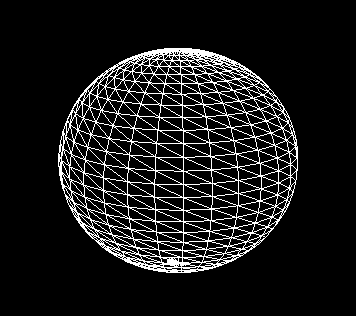Fig 9.3

To create the sphere we can use the following code snippet. The code below has been adapted from a sample by "Laurent" posted on the GameDev.net DirectX forum. To view the post in full go to http://www.gamedev.net/community/forums/topic.asp?topic_id=85779. I would like to thank Laurent for giving me permission to use the code in this tutorial.

`bool CSphere::UpdateVertices() {`

`¬Ý//Code adapted from a sample by "Laurent" posted on the GameDev.net DirectX forum`

`¬Ý//http://www.gamedev.net/community/forums/topic.asp?topic_id=85779`

`¬ÝWORD* pIndices;`

`¬ÝSPHERE_CUSTOMVERTEX* pVertex;`

`¬ÝWORD wVertexIndex = 0;`

`¬Ýint nCurrentRing;`

`¬Ýint nCurrentSegment;`

`¬ÝD3DXVECTOR3 vNormal;`

`¬Ý//Lock the vertex buffer`

`¬Ýif (FAILED(m_pVertexBuffer->Lock(0, 0, (BYTE**)&pVertex, 0))) {`

`¬Ý LogError("<li>CSphere: Unable to lock vertex buffer.");`

`¬Ý return false;`

`¬Ý}`

`¬Ý//Lock the index buffer`

`¬Ýif (FAILED(m_pIndexBuffer->Lock(0, m_dwNumOfIndices, (BYTE**)&pIndices, 0))) {`

`¬Ý¬ÝLogError("<li>CSphere: Unable to lock index buffer.");`

`¬Ý¬Ýreturn false;`

`¬Ý}`

`¬Ý//Establish constants used in sphere generation`

`¬ÝFLOAT rDeltaRingAngle = (D3DX_PI / m_nRings);`

`¬ÝFLOAT rDeltaSegAngle = (2.0f * D3DX_PI / m_nSegments);`

`¬Ý//Generate the group of rings for the sphere`

`¬Ýfor (nCurrentRing = 0; nCurrentRing < m_nRings + 1; nCurrentRing++) {`

`¬Ý FLOAT r0 = sinf(nCurrentRing * rDeltaRingAngle);`

`¬Ý FLOAT y0 = cosf(nCurrentRing * rDeltaRingAngle);`

`¬Ý //Generate the group of segments for the current ring`

`¬Ý for (nCurrentSegment = 0; nCurrentSegment < m_nSegments + 1; nCurrentSegment++) {`

`¬Ý¬Ý FLOAT x0 = r0 * sinf(nCurrentSegment * rDeltaSegAngle);`

`¬Ý¬Ý FLOAT z0 = r0 * cosf(nCurrentSegment * rDeltaSegAngle);`

`¬Ý¬Ý vNormal.x = x0;`

`¬Ý¬Ý vNormal.y = y0;`

`¬Ý¬Ý vNormal.z = z0;`

`¬Ý¬Ý D3DXVec3Normalize(&vNormal, &vNormal);`

`¬Ý¬Ý //Add one vertex to the strip which makes up the sphere`

`¬Ý¬Ý pVertex->x = x0;`

`¬Ý¬Ý pVertex->y = y0;`

`¬Ý¬Ý pVertex->z = z0;`

`¬Ý¬Ý pVertex->nx = vNormal.x;`

`¬Ý¬Ý pVertex->ny = vNormal.y;`

`¬Ý¬Ý pVertex->nz = vNormal.z;`

`¬Ý¬Ý pVertex->tu = 1.0f ‚Äì ((FLOAT)nCurrentSegment / (FLOAT)m_nSegments);`

`¬Ý¬Ý pVertex->tv = (FLOAT)nCurrentRing / (FLOAT)m_nRings;`

`¬Ý¬Ý pVertex++;`

`¬Ý¬Ý //Add two indices except for the last ring`

`¬Ý¬Ý if (nCurrentRing != m_nRings) {`

`¬Ý¬Ý¬Ý *pIndices = wVertexIndex;`

`¬Ý¬Ý¬Ý pIndices++;`

`¬Ý¬Ý¬Ý *pIndices = wVertexIndex + (WORD)(m_nSegments + 1);`

`¬Ý¬Ý¬Ý pIndices++;`

`¬Ý¬Ý¬Ý wVertexIndex++;`

`¬Ý¬Ý }`

`¬Ý }`

`¬Ý}`

`¬Ýif (FAILED(m_pIndexBuffer->Unlock())) {`

`¬Ý LogError("<li>CSphere: Unable to unlock index buffer.");`

`¬Ý return false;`

`¬Ý}`

`¬Ýif (FAILED(m_pVertexBuffer->Unlock())) {`

`¬Ý LogError("<li>CSphere: Unable to unlock vertex buffer.");`

`¬Ý return false;`

`¬Ý}`

`¬Ýreturn true;`

`}`

So what is going on here? Once we have locked the index and vertex buffers we are ready to write to them. We use the same calculation as before to obtain the segment angle and a slight variation to obtain the ring angle. For the ring angle we only need to divide half a circle (PI radians) by the number of rings.

Once we have the ring and segment angles, we simply loop around once for each ring creating the vertices for each segment within that ring. We add an entry into the index buffer each time to create our triangle list. We set the normal values for each vertex to be the normalised position values. The radius of the sphere is always 1. You can use a scaling transformation matrix to increase or decrease the size of the sphere. Once complete, we unlock the vertex and index buffers ready for rendering.

The final scene when rendered will look something like the screenshot below: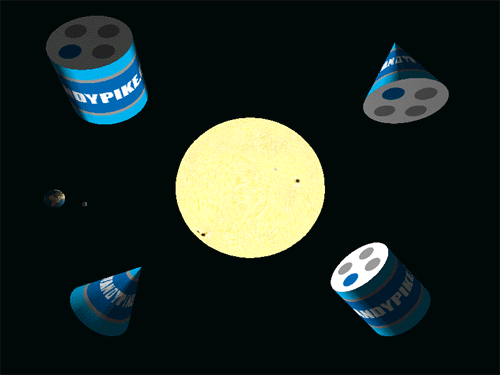## HTML Logging

You may have notices in the code above, some new logging functions called LogInfo and LogError. Well, by using these new logging functions we can create a more readable log file. Errors will be highlighted in red and normal information will be in black. To see the log file, take a look in the project folder at this file called Log.htm once you have run the sample code. There is also a LogWarning function, which you can use to log warnings that will appear in orange. There is also a LogMemoryUsage() function which will log the amount of memory your application is currently using. Do not use this function inside your game loop at it is far to slow.

## Summary

Now we can create quite a few shapes: a cube, a sphere, a cone, a cylinder and even a simple terrain. These shapes are pretty basic, but can be very useful when you start creating your own scenes. In the next tutorial, we'll look at creating more complex objects like aliens, guns and vehicles.

# DirectX Tutorial 10: Loading Complex Models

## Introduction

In this tutorial we will look at how to create a complex model and load it into your DirectX application. So far, we have created some simple shapes: cubes, spheres, cones, etc. But when it comes to creating a game we will need to create objects like guns, people, buildings and spaceships. You could workout where all the vertices for an object are on a piece of paper and then create the object in code, or you could use a piece of software called a "3D modeller". I would recommend using the modelling software! It is far easier! You can download the full source code by clicking the "Download Source" link above.

## 3D Modelling Software

You will need to use a 3D modelling package to create your game objects. Below is a list of 3D modellers that you may like to try (in order of price). There are many more 3D modelling packages available, but from my research for this topic, these seem to be the most popular.

To use a 3D modelling package with DirectX, it must be able to export your model to a .x file. This can be done as a built-in feature, as an optional plug-in or you could use a conversion program to convert one file format into the .x file format.

Package Website Price*
3D Studio Max http://www.discreet.com/ \$3495
Maya http://www.aliaswavefront.com/ \$1999
Cinema 4D http://www.cinema4d.com/ \$1695
TrueSpace http://www.caligari.com/ \$595
3D Canvas LP http://www.amabilis.com/ \$34.95
MilkShape 3D http://www.milkshape3d.com/ \$20
OpenFX http://www.openfx.org/ Free

* Prices are correct at time of publication. Please go to the products website to confirm current pricing. You can convert these prices into your local currency at http://www.oanda.com/.

So which one should you use? Well, that is entirely up to you. However, I have tried 3D Canvas (trial version), MilkShape 3D (trial version) and OpenFX (full version). I tried these three packages because their price was so low, which is good if you are creating games as a hobby.

3D Canvas is a good piece of software but it has one major downside. If you are using Windows XP or Windows 2000 (like me) it can reboot your machine without warning! The help advised me that updating my display driver to the latest version would fix the problem‚Ä¶ but I have the latest version already! So, I'm afraid 3D Canvas is no good for me.

MilkShape 3D is an excellent package for creating low polygon models. It has most of the features that you need to create a 3D model, and is pretty easy to use. There are loads of tutorials on the web and a forum on the MilkShape site that is really useful.

OpenFX is a great all round 3D modeller. I found it easy to use and very powerful. The main problem I had was exporting my model into a .x file. The only way I found was to export to a .3ds file and then use the conv3ds.exe tool that comes with the DirectX SDK to convert it to a .x file. The only problem was that it lost the textures when exporting to a .3ds file. There is also very limited help. So, OpenFX isn't the right tool for me.

So, I have decided to use MilkShape 3D because it is cheap, it can export to a .x file (via a free plug-in) and it is easy to use.

## Creating a .x file with MilkShape 3D

Once you have your 3D modelling package, the next thing to do is create a model. For this tutorial have created a simple spaceship. I used the tutorials http://xu1productions.com/3dstudio/tutorials.html to get me started with MilkShape. Fig 10.1 below, shows a screenshot of MilkShape 3D with my completed spaceship model.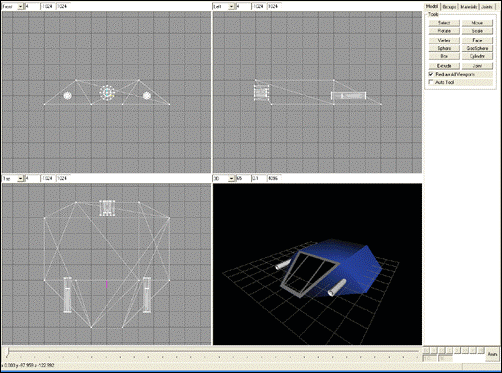Fig 10.1

Once your model is complete, you need to export it to a .x file. To do this with MilkShape, download and install the "DirectX 8.1 Exporter" plug-in by John Thompson from the MilkShape website. Then open your model in MilkShape and go to "File"‚Üí"Export"‚Üí"DirectX (JT)‚Ä¶". Select a location for your .x file, then select the options you require (normally the defaults) and press "OK". You are now ready to load your model into your DirectX program.

For this tutorial I have created a wrapper class called CMesh for loading and rendering meshes loaded from a .x file. Below is the main code for the CMesh class. The constuctor shows how to load a mesh from a .x file and store it in memory. The destructor shows how to free the memory that was used to store the mesh. The Render method shows how to render a mesh.

The CMesh constructor takes two parameters, the first is a pointer to the device and the second is string containing the path of the .x file to load. We use the D3DXLoadMeshFromX function to load the mesh into memory. Once this is done we create two arrays, one to hold the materials and one to hold the textures of our model. We then loop around and populate the two arrays from the loaded mesh. The last thing to do is make sure that the normals are set for each vertex of the mesh. We clone the mesh and use the D3DXComputeNormals function to set the normals.

In the destructor we release each texture and the mesh itself. We also have to delete the two array pointers that we created in the constructor.

The Render function is pretty straight forward, we simply loop through each subset of the mesh and render it with the appropriate texture and material.

`CMesh::CMesh(LPDIRECT3DDEVICE8 pD3DDevice, LPSTR pFilename) {`

`¬ÝLPD3DXBUFFER pMaterialsBuffer = NULL;`

`¬ÝLPD3DXMESH pMesh = NULL;`

`¬Ým_pD3DDevice = pD3DDevice;`

`¬Ýif (FAILED(D3DXLoadMeshFromX(pFilename, D3DXMESH_SYSTEMMEM, m_pD3DDevice, NULL, &pMaterialsBuffer, &m_dwNumMaterials, &pMesh))) {`

`¬Ý m_pMesh = NULL;`

`¬Ý m_pMeshMaterials = NULL;`

`¬Ý m_pMeshTextures = NULL;`

`¬Ý LogError("<li>Mesh '%s' failed to load", pFilename);`

`¬Ý return;`

`¬Ý}`

`¬ÝD3DXMATERIAL* matMaterials = (D3DXMATERIAL*)pMaterialsBuffer->GetBufferPointer();`

`¬Ý//Create two arrays. One to hold the materials and only to hold the textures`

`¬Ým_pMeshMaterials = new D3DMATERIAL8[m_dwNumMaterials];`

`¬Ým_pMeshTextures = new LPDIRECT3DTEXTURE8[m_dwNumMaterials];`

`¬Ýfor (DWORD i = 0; i < m_dwNumMaterials; i++) {`

`¬Ý //Copy the material`

`¬Ý m_pMeshMaterials[i] = matMaterials[i].MatD3D;`

`¬Ý //Set the ambient color for the material (D3DX does not do this)`

`¬Ý m_pMeshMaterials[i].Ambient = m_pMeshMaterials[i].Diffuse;`

`¬Ý //Create the texture`

`¬Ý if (FAILED(D3DXCreateTextureFromFile(m_pD3DDevice, matMaterials[i].pTextureFilename, &m_pMeshTextures[i]))) {`

`¬Ý¬Ý m_pMeshTextures[i] = NULL;`

`¬Ý }`

`¬Ý}`

`¬Ý//We've finished with the material buffer, so release it`

`¬ÝSafeRelease(pMaterialsBuffer);`

`//Make sure that the normals are setup for our mesh`

`¬ÝpMesh->CloneMeshFVF(D3DXMESH_MANAGED, MESH_D3DFVF_CUSTOMVERTEX, m_pD3DDevice, &m_pMesh);`

`¬ÝSafeRelease(pMesh);`

`¬ÝD3DXComputeNormals(m_pMesh);`

`¬ÝLogInfo("<li>Mesh '%s' loaded OK", pFilename);`

`}`

`CMesh::~CMesh() {`

`¬ÝSafeDelete(m_pMeshMaterials);`

`¬Ýif (m_pMeshTextures != NULL) {`

`¬Ý for (DWORD i = 0; i < m_dwNumMaterials; i++) {`

`¬Ý¬Ý if (m_pMeshTextures[i]) {`

`¬Ý¬Ý¬Ý SafeRelease(m_pMeshTextures[i]);`

`¬Ý¬Ý }`

`¬Ý }`

`¬Ý}`

`¬ÝSafeDelete(m_pMeshTextures);`

`¬ÝSafeRelease(m_pMesh);`

`¬ÝLogInfo("<li>Mesh destroyed OK");`

`}`

`DWORD CMesh::Render() {`

`¬Ýif (m_pMesh != NULL) {`

`¬Ý for (DWORD i = 0; i < m_dwNumMaterials; i++) {`

`¬Ý¬Ý m_pD3DDevice->SetMaterial(&m_pMeshMaterials[i]);`

`¬Ý¬Ý m_pD3DDevice->SetTexture(0, m_pMeshTextures[i]);`

`¬Ý¬Ý m_pMesh->DrawSubset(i);`

`¬Ý }`

`¬Ý return m_pMesh->GetNumFaces();`

`¬Ý} else {`

`¬Ý return 0;`

`¬Ý}`

`}`

## Scaling makes your object darker?

One extra thing to note is that if you scale the mesh you will also scale the normals. This will have the effect of making the object darker the more it is scaled. To fix this problem we need to enable the D3DRS_NORMALIZENORMALS render state. This is done with one call to the SetRenderState function as shown below.

`m_pD3DDevice->SetRenderState(D3DRS_NORMALIZENORMALS, TRUE);`

For this tutorial we will create three spaceships and rotate them each about a different axis. The final scene when rendered will look something like the screenshot below: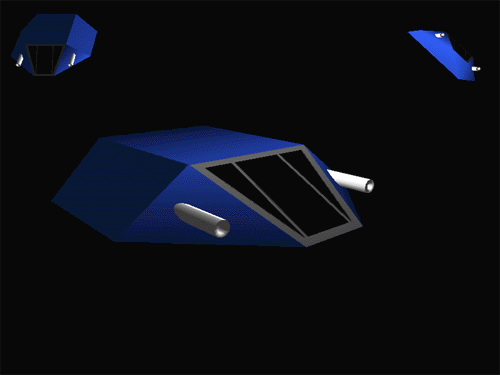## Summary

So now we can create any object we like, we are no longer limited to cubes and spheres. Remember, when you create your models, make sure they have a low number of polygons. The more polygons you have the more your frame rate will drop. In the next tutorial we will look at adding 2D elements to a 3D scene. That is useful when it comes to creating scores and energy bars.

# DirectX Tutorial 11: 2D in 3D

## Introduction

In this tutorial we will add some 2D elements to a 3D scene. This will be useful for adding things like energy bars, timers, radars and so on. We will also look at how to do texture transparency so that your 2D elements can appear non-rectangular. You can download the full source code by clicking the "Download Source" link above.

The first and easiest thing to do is add some 2D text to your scene. For this, I have created a simple wrapper class called CFont. The code for this class is shown below.

`CFont::CFont(LPDIRECT3DDEVICE8 pD3DDevice, LPSTR pFontFace, int nHeight, bool fBold, bool fItalic, bool fUnderlined) {`

`¬ÝHFONT hFont;`

`¬Ým_pD3DDevice = pD3DDevice;`

`¬Ýint nWeight = FW_NORMAL;`

`¬ÝDWORD dwItalic = 0;`

`¬ÝDWORD dwUnderlined = 0;`

`¬Ýif (fBold) {`

`¬Ý nWeight = FW_BOLD;`

`¬Ý}`

`¬Ýif (fItalic) {`

`¬Ý dwItalic = 1;`

`¬Ý}`

`¬Ýif (fUnderlined) {`

`¬Ý dwUnderlined = 1;`

`¬Ý}`

`¬ÝhFont = CreateFont(nHeight, 0, 0, 0, nWeight, dwItalic, dwUnderlined, 0, ANSI_CHARSET, 0, 0, 0, 0, pFontFace);`

`¬ÝD3DXCreateFont(m_pD3DDevice, hFont, &m_pFont);`

`¬ÝLogInfo("<li>Font loaded OK");`

`}`

`CFont::~CFont() {`

`¬ÝSafeRelease(m_pFont);`

`¬ÝLogInfo("<li>Font destroyed OK");`

`}`

`void CFont::DrawText(LPSTR pText, int x, int y, D3DCOLOR rgbFontColour) {`

`¬ÝRECT Rect;`

`¬ÝRect.left = x;`

`¬ÝRect.top = y;`

`¬ÝRect.right = 0;`

`¬ÝRect.bottom = 0;`

`¬Ým_pFont->Begin();`

`¬Ým_pFont->DrawTextA(pText, ‚Äì1, &Rect, DT_CALCRECT, 0); //Calculate the size of the rect needed`

`¬Ým_pFont->DrawTextA(pText, ‚Äì1, &Rect, DT_LEFT, rgbFontColour); //Draw the text`

`¬Ým_pFont->End();`

`}`

In the constructor of CFont, we use the CreateFont function to get a handle to a new font (HFONT). Now, to use this font in your DirectX application you need to create a font object for the device. To do this, we use the D3DXCreateFont function, passing in the device pointer and font handle. We then store the resulting font pointer (LPD3DXFONT) as a member variable of the CFont class.

In the destructor we simply release the stored font object.

The final function, DrawText, renders the text. We pass in the text to render, the x and y position of the upper left corner (in screen coordinates) and the colour of the text. We use two calls to the DrawTextA method. The first calculates the dimensions of the bounding rectangle of the text and the second draws the text inside the rectangle. The text is left aligned inside the rectangle.

To use the CFont class we use the following code to create a CFont pointer:

`m_pFont = new CFont(m_pD3DDevice, "Verdana", 12, false, false, false);`

Once we have a pointer to the CFont object we can start drawing text. So, we have a new CGame method called RenderText which is called from inside the main Render method.

`void CGame::RenderText() {`

`¬Ý//Draw some text at the top of the screen showing stats`

`¬Ýchar buffer;`

`¬ÝDWORD dwDuration = (timeGetTime() ‚Äì m_dwStartTime) / 1000;`

`¬Ýif (dwDuration > 0) {`

`¬Ý sprintf(buffer, "Duration: %d seconds. Frames: %d. FPS: %d.", dwDuration, m_dwFrames, (m_dwFrames / dwDuration));`

`¬Ý} else {`

`¬Ý sprintf(buffer, "Calculating‚Ä¶");`

`¬Ý}`

`¬Ým_pFont->DrawText(buffer, 0, 0, D3DCOLOR_XRGB(255, 255, 255));`

`}`

So for every frame, the RenderText method is called. RenderText simply displays the length of time that the application has been running (in seconds), the number of frames so far and the average FPS count.

## Steps for 2D in 3D Rendering

Now, to create 2D graphics is really pretty simple. All we need to do, is the following steps for each frame:

¬∑ Setup camera for 3D as usual

¬∑¬ÝEnable the z-buffer and lighting

¬∑¬ÝRender 3D objects as usual

¬∑¬ÝSetup camera for 2D

¬∑¬ÝDisable the z-buffer and lighting

¬∑¬ÝRender 2D objects

## 2D Camera

To setup the camera for 2D objects we need to change the projection matrix from a perspective one used in 3D to an orthogonal projection. When using a perspective projection, objects that are further away from the camera are smaller than objects that are closer to the camera. When using an orthogonal projection, an object will be the same size no matter how far it is from the camera. For example, when you use your 3D modelling program, you normally get three orthogonal projections (top, side and front) and a perspective projection (3D). The code for setting the camera for 2D is shown below:

`void CGame::Setup2DCamera() {`

`¬ÝD3DXMATRIX matOrtho;`

`¬ÝD3DXMATRIX matIdentity;`

`¬Ý//Setup the orthogonal projection matrix and the default world/view matrix`

`¬ÝD3DXMatrixOrthoLH(&matOrtho, (float)m_nScreenWidth, (float)m_nScreenHeight, 0.0f, 1.0f);`

`¬ÝD3DXMatrixIdentity(&matIdentity);`

`¬Ým_pD3DDevice->SetTransform(D3DTS_PROJECTION, &matOrtho);`

`¬Ým_pD3DDevice->SetTransform(D3DTS_WORLD, &matIdentity);`

`¬Ým_pD3DDevice->SetTransform(D3DTS_VIEW, &matIdentity);`

`¬Ý//Make sure that the z-buffer and lighting are disabled`

`¬Ým_pD3DDevice->SetRenderState(D3DRS_ZENABLE, D3DZB_FALSE);`

`¬Ým_pD3DDevice->SetRenderState(D3DRS_LIGHTING, FALSE);`

`}`

In addition to changing the projection matrix, we also set the world and view matrices to an identity matrix and disable the z-buffer and lighting. We disable the z-buffer so that everything that is rendered from then on will appear on top of objects that have already been rendered (3D objects).

## 2D Objects

A 2D object is really two textured triangles in a triangle strip that form a rectangle. The z value for each of the vertices is the same so that the 2D object faces the camera flat. I have created a class called CPanel which we will use as our 2D object.

CPanel is very similar to the objects we have already created. It has a vertex buffer which contains four vertices (one for each corner of the panel), and the panel is centred about the origin. The code for CPanel is shown below.

`CPanel::CPanel(LPDIRECT3DDEVICE8 pD3DDevice, int nWidth, int nHeight, int nScreenWidth, int nScreenHeight, DWORD dwColour) {`

`¬Ým_pD3DDevice = pD3DDevice;`

`¬Ým_pVertexBuffer = NULL;`

`¬Ým_pTexture = NULL;`

`¬Ým_nWidth = nWidth;`

`¬Ým_nHeight = nHeight;`

`¬Ým_nScreenWidth = nScreenWidth;`

`¬Ým_nScreenHeight = nScreenHeight;`

`¬Ým_dwColour = dwColour;`

`¬Ý//Initialize Vertex Buffer`

`¬Ýif (CreateVertexBuffer()) {`

`¬Ý if (UpdateVertices()) {`

`¬Ý¬Ý LogInfo("<li>Panel created OK");`

`¬Ý¬Ý return;`

`¬Ý }`

`¬Ý}`

`¬ÝLogError("<li>Panel failed to create");`

`}`

`CPanel::~CPanel() {`

`¬ÝSafeRelease(m_pTexture);`

`¬ÝSafeRelease(m_pVertexBuffer);`

`¬ÝLogInfo("<li>Panel destroyed OK");`

`}`

`bool CPanel::CreateVertexBuffer() {`

`¬Ý//Create the vertex buffer from our device.`

`¬Ýif (FAILED(m_pD3DDevice->CreateVertexBuffer(4 * sizeof(PANEL_CUSTOMVERTEX), 0, PANEL_D3DFVF_CUSTOMVERTEX, D3DPOOL_DEFAULT, &m_pVertexBuffer))) {`

`¬Ý LogError("<li>CPanel: Unable to create vertex buffer.");`

`¬Ý return false;`

`¬Ý}`

`¬Ýreturn true;`

`}`

`bool CPanel::UpdateVertices() {`

`¬ÝPANEL_CUSTOMVERTEX* pVertices = NULL;`

`¬Ým_pVertexBuffer->Lock(0, 4 * sizeof(PANEL_CUSTOMVERTEX), (BYTE**)&pVertices, 0);`

`¬Ýif (m_dwColour == ‚Äì1) {`

`¬Ý //No colour was set, so default to white`

`¬Ý m_dwColour = D3DCOLOR_XRGB(255, 255, 255);`

`¬Ý}`

`¬Ý//Set all the vertices to selected colour`

`¬ÝpVertices.colour = m_dwColour;`

`¬ÝpVertices.colour = m_dwColour;`

`¬ÝpVertices.colour = m_dwColour;`

`¬ÝpVertices.colour = m_dwColour;`

`¬Ý//Set the positions of the vertices`

`¬ÝpVertices.x = ‚Äì(m_nWidth) / 2.0f;`

`¬ÝpVertices.y = ‚Äì(m_nHeight) / 2.0f;`

`¬ÝpVertices.x = ‚Äì(m_nWidth) / 2.0f;`

`¬ÝpVertices.y = m_nHeight / 2.0f;`

`¬ÝpVertices.x = (m_nWidth) / 2.0f;`

`¬ÝpVertices.y = ‚Äì(m_nHeight) / 2.0f;`

`¬ÝpVertices.x = (m_nWidth) / 2.0f;`

`¬ÝpVertices.y = m_nHeight / 2.0f;`

`¬ÝpVertices.z = 1.0f;`

`¬ÝpVertices.z = 1.0f;`

`¬ÝpVertices.z = 1.0f;`

`¬ÝpVertices.z = 1.0f;`

`¬Ý//Set the texture coordinates of the vertices`

`¬ÝpVertices.u = 0.0f;`

`¬ÝpVertices.v = 1.0f;`

`¬ÝpVertices.u = 0.0f;`

`¬ÝpVertices.v = 0.0f;`

`¬ÝpVertices.u = 1.0f;`

`¬ÝpVertices.v = 1.0f;`

`¬ÝpVertices.u = 1.0f;`

`¬ÝpVertices.v = 0.0f;`

`¬Ým_pVertexBuffer->Unlock();`

`¬Ýreturn true;`

`}`

`bool CPanel::SetTexture(const char *szTextureFilePath, DWORD dwKeyColour) {`

`¬Ýif (FAILED(D3DXCreateTextureFromFileEx(m_pD3DDevice, szTextureFilePath, 0, 0, 0, 0, D3DFMT_UNKNOWN, D3DPOOL_MANAGED, D3DX_DEFAULT, D3DX_DEFAULT, dwKeyColour, NULL, NULL, &m_pTexture))) {`

`¬Ý¬Ýreturn false;`

`¬Ý}`

`¬Ýreturn true;`

`}`

`DWORD CPanel::Render() {`

`¬Ým_pD3DDevice->SetStreamSource(0, m_pVertexBuffer, sizeof(PANEL_CUSTOMVERTEX));`

`¬Ým_pD3DDevice->SetVertexShader(PANEL_D3DFVF_CUSTOMVERTEX);`

`¬Ýif (m_pTexture != NULL) {`

`¬Ý m_pD3DDevice->SetTexture(0, m_pTexture);`

`¬Ý m_pD3DDevice->SetTextureStageState(0, D3DTSS_ALPHAOP, D3DTOP_MODULATE);`

`¬Ý} else {`

`¬Ý m_pD3DDevice->SetTexture(0, NULL);`

`¬Ý}`

`¬Ým_pD3DDevice->DrawPrimitive(D3DPT_TRIANGLESTRIP, 0, 2);`

`¬Ýreturn 2; //Return the number of polygons rendered`

`}`

`void CPanel::MoveTo(int x, int y) {`

`¬Ý//x and y specify the top left corner of the panel in screen coordinates`

`¬ÝD3DXMATRIX matMove;`

`¬Ýx ‚Äì= (m_nScreenWidth / 2) ‚Äì (m_nWidth / 2);`

`¬Ýy ‚Äì= (m_nScreenHeight / 2) ‚Äì (m_nHeight / 2);`

`¬ÝD3DXMatrixTranslation(&matMove, (float)x, ‚Äì(float)y, 0.0f);`

`¬Ým_pD3DDevice->SetTransform(D3DTS_WORLD, &matMove);`

`}`

The only slight changes from usual are that the SetTexture and Render methods have changed to enable texture transparencies (we will look at this in a moment).

There is also a new function called MoveTo which will move the panel from the centre of the screen, to any position you specify. It takes two parameters x and y which are screen coordinates for the top left corner of the panel. We then use the normal matrix translation functions to move the panel. We can also rotate the panel by using the normal rotation matrices.

## Texture Transparency

The first thing to do is to enable alpha blending so that we can use transparent textures. We do this with a few calls to SetRenderState, the code to enable alpha blending is shown below:

`//Enable alpha blending so we can use transparent textures`

`m_pD3DDevice->SetRenderState(D3DRS_ALPHABLENDENABLE, TRUE);`

`//Set how the texture should be blended (use alpha)`

`m_pD3DDevice->SetRenderState(D3DRS_SRCBLEND, D3DBLEND_SRCALPHA);`

`m_pD3DDevice->SetRenderState(D3DRS_DESTBLEND, D3DBLEND_INVSRCALPHA);`

There are two further changes to make. The first is the way we load the textures. We need to use the D3DXCreateTextureFromFileEx function, this will enable us to specify a key colour. This means that any pixel in the texture that is the same colour as the key colour will be made transparent. Fig 11.1, 11.2 and 11.3 below show the three textures that I have used for this tutorial. I have specified that the black pixels should be transparent.

`D3DXCreateTextureFromFileEx(m_pD3DDevice, szTextureFilePath, 0, 0, 0, 0, D3DFMT_UNKNOWN, D3DPOOL_MANAGED, D3DX_DEFAULT, D3DX_DEFAULT, dwKeyColour, NULL, NULL, &m_pTexture);`Fig 11.1Fig 11.2Fig 11.3

The second change is how the texture should be rendered. The SetTextureStageState function has been changed in the Render method of CPanel to render the texture using transparencies.

`m_pD3DDevice->SetTextureStageState(0, D3DTSS_ALPHAOP, D3DTOP_MODULATE);`

From the last tutorial we have three rotating spaceships. We've added some 2D elements to the scene, each with some transparency in the texture. The final scene when rendered will look something like the screenshot below:## Summary

Now, we have completed the basics to rendering graphics in DirectX. There is lots more advanced topics in DirectX Graphics which we will cover in future tutorials. In the next tutorial we will see how to add some user interaction with the keyboard and mouse using DirectInput, vital to any game.

# DirectX Tutorial 12: Keyboard and Mouse Input

## Introduction

In this tutorial we will create a simple Earth object that the user can control. The user will be able to use the mouse to change the Earth's position. The user will also be able to change the Earth's scale and rotation by using the keyboard. You can download the full source code by clicking the "Download Source" link above.

## DirectInput

So far we have been using Direct3D to draw 3D objects. Now we want to add some user interaction to our application. We can do this by using DirectInput. DirectInput allows us to get data from any input device: mouse, keyboard or joystick. We can then use this data to change elements in our scene. In this tutorial we will be looking at the mouse and keyboard only, we'll take a look at joysticks in a future tutorial.

In our application, we will need to do the following steps to add user interaction.

¬∑¬ÝInitialise DirectInput

¬∑¬ÝSetup the keyboard

¬∑¬ÝSetup the mouse

¬∑¬ÝFor each frame, get the state of the keyboard and mouse and use it to modify the scene

¬∑¬ÝOnce finished, clean up DirectInput

## Include and Library files

First thing's first. To use DirectInput we need to add a new include file and two library files to our project. If you don't add these to your project you'll get compiler and linker errors. I've included the new header file in Game.h just below where I have included d3dx8.h. You can add the library files by going to Project > Settings‚Ä¶ then on the Link tab, type the new library file names into the Object/Library Modules input box. The new files are listed below:

¬∑¬Ýdinput.h

¬∑¬Ýdinput8.lib

¬∑¬Ýdxguid.lib

## The Controls

The controls for this tutorial will be as follows:

¬∑¬ÝUp arrow: Scale Earth up

¬∑¬ÝDown arrow: Scale Earth down

¬∑¬ÝLeft arrow: Rotate Earth more to the left

¬∑¬ÝRight arrow: Rotate Earth more to the right

¬∑¬ÝMove mouse: Change Earth x and y position

¬∑¬ÝLeft mouse button: Stop Earth rotation

¬∑¬ÝRight mouse button: Start Earth rotation at default speed and direction

## Initialising DirectInput

The first thing we need to do is to initialise DirectInput. To do this, I have a new function called InitialiseDirectInput that is called from the main CGame::Initialise function. The code snippet below will create a DirectInput object that we will use to create further objects for user input.

`//Create the DirectInput object`

`if (FAILED(DirectInput8Create(hInst, DIRECTINPUT_VERSION, IID_IDirectInput8, (void**)&m_pDirectInput, NULL))) {`

`¬ÝLogError("<li>Unable to create DirectInput interface.");`

`¬Ýreturn false;`

`} else {`

`¬ÝLogInfo("<li>DirectInput interface created OK");`

`}`

The first parameter to DirectInput8Create is the HINSTANCE of our application which has been passed through from our WinMain function. The second parameter is the DirectInput version, this will always be DIRECTINPUT_VERSION. The third parameter is the desired interface, this will always be IID_IDirectInput8. The fourth parameter is the important one, this is a pointer the DirectInput interface which we will use later. The fifth parameter, for our examples is always NULL.

m_pDirectInput is a member variable of CGame and is of type LPDIRECTINPUT8.

## Setting up the keyboard

Once we have created DirectInput interface pointer, we are ready to setup the keyboard. Shown below is the code required to setup the keyboard, take a look at it and I'll explain it below.

`//KEYBOARD =======================================================================`

`//Create the keyboard device object`

`if (FAILED(m_pDirectInput->CreateDevice(GUID_SysKeyboard, &m_pKeyboard, NULL))) {`

`¬ÝCleanUpDirectInput();`

`¬ÝLogError("<li>Unable to create DirectInput keyboard device interface.");`

`¬Ýreturn false;`

`} else {`

`¬ÝLogInfo("<li>DirectInput keyboard device interface created OK.");`

`}`

`//Set the data format for the keyboard`

`if (FAILED(m_pKeyboard->SetDataFormat(&c_dfDIKeyboard))) {`

`¬ÝCleanUpDirectInput();`

`¬ÝLogError("<li>Unable to set the keyboard data format.");`

`¬Ýreturn false;`

`} else {`

`¬ÝLogInfo("<li>Set the keyboard data format OK.");`

`}`

`//Set the cooperative level for the keyboard`

`if (FAILED(m_pKeyboard->SetCooperativeLevel(hWnd, DISCL_FOREGROUND | DISCL_NONEXCLUSIVE))) {`

`¬ÝCleanUpDirectInput();`

`¬ÝLogError("<li>Unable to set the keyboard cooperative level.");`

`¬Ýreturn false;`

`} else {`

`¬ÝLogInfo("<li>Set the keyboard cooperative level OK.");`

`}`

`//Acquire the keyboard`

`if (m_pKeyboard) {`

`¬Ým_pKeyboard->Acquire();`

`}`

First of all, we need to create a keyboard device. We do this by calling the CreateDevice method of DirectInput. The first parameter is the GUID (Globally Unique Identifier) of the device to create, in our case the system keyboard device, so we simply pass in the GUID_SysKeyboard predefined GUID. The next parameter will receive a pointer to the keyboard device, we have defined this variable as a member of CGame. The last parameter is always NULL, take a look in the SDK for further details.

The next thing to do is set the data format for the keyboard device. To do this we simply pass in the predefined keyboard format c_dfDIKeyboard.

Then, we need to set how our input device (the keyboard) will cooperate with our application and Windows. We will need to specify the cooperation level by selecting "foreground" or "background" and "exclusive" or "nonexclusive". To do this, we need to pass in the relevant flags (DISCL_FOREGROUND, DISCL_BACKGROUND, DISCL_EXCLUSIVE and DISCL_NONEXCLUSIVE) to the SetCooperativeLevel method.

¬∑¬ÝDISCL_FOREGROUND: Input device is available when the application is in the foreground (has focus).

¬∑¬ÝDISCL_BACKGROUND: Input device is available at all times, foreground and background.

¬∑¬ÝDISCL_EXCLUSIVE: No other instance of the device can obtain exclusive access to the device while it is acquired.

¬∑¬ÝDISCL_NONEXCLUSIVE: Access to the device does not interfere with other applications that are accessing the same device.

The final thing to do is to acquire the keyboard, this means that we can now get access to the device's data. We do this by calling the Acquire method of the device.

## Setting up the mouse

As with the keyboard, we need to set up the mouse before we can get access to it. Below is the code required, take a look and you'll notice that it is very similar to the keyboard set up code above.

`//MOUSE =======================================================================`

`//Create the mouse device object`

`if (FAILED(m_pDirectInput->CreateDevice(GUID_SysMouse, &m_pMouse, NULL))) {`

`¬ÝCleanUpDirectInput();`

`¬ÝLogError("<li>Unable to create DirectInput mouse device interface.");`

`¬Ýreturn false;`

`} else {`

`¬ÝLogInfo("<li>DirectInput mouse device interface created OK.");`

`}`

`//Set the data format for the mouse`

`if (FAILED(m_pMouse->SetDataFormat(&c_dfDIMouse))) {`

`¬ÝCleanUpDirectInput();`

`¬ÝLogError("<li>Unable to set the mouse data format.");`

`¬Ýreturn false;`

`} else {`

`¬ÝLogInfo("<li>Set the mouse data format OK.");`

`}`

`//Set the cooperative level for the mouse`

`if (FAILED(m_pMouse->SetCooperativeLevel(hWnd, DISCL_FOREGROUND | DISCL_NONEXCLUSIVE))) {`

`¬ÝCleanUpDirectInput();`

`¬ÝLogError("<li>Unable to set the mouse cooperative level.");`

`¬Ýreturn false;`

`} else {`

`¬ÝLogInfo("<li>Set the mouse cooperative level OK.");`

`}`

`//Acquire the mouse`

`if (m_pMouse) {`

`¬Ým_pMouse->Acquire();`

`}`

So, for the mouse we have the same basic steps as for the keyboard. One of the differences is that we pass in the predefined GUID for the system mouse (GUID_SysMouse) into the CreateDevice method instead of the one for the keyboard which will return a pointer to the mouse. Another difference is that we need to use a different data format, c_dfDIMouse instead of c_dfDIKeyboard. We then set the cooperation level as before and finally acquire the mouse. We are now ready to get data from the keyboard and mouse.

## Buffered and Immediate Data

Now that we have setup the mouse and keyboard, we need to get data from them. There are two forms of data that we can get, buffered and immediate data. Buffered data is a record of events that are saved (buffered) until the application requires them. Immediate data is the current state of the device. In this tutorial we will look at immediate data.

## Getting keyboard data

We have a new function called ProcessKeyboard which is called from our Render function. This means that ProcessKeyboard will be called once per frame. The code for ProcessKeyboard is shown below.

`void CGame::ProcessKeyboard() {`

`¬Ýchar KeyboardState;`

`¬Ýif (FAILED(m_pKeyboard->GetDeviceState(sizeof(KeyboardState),(LPVOID)&KeyboardState))) {`

`¬Ý return;`

`¬Ý}`

`¬Ýif (KEYDOWN(KeyboardState, DIK_ESCAPE)) {`

`¬Ý //Escape key pressed. Quit game.`

`¬Ý m_fQuit = true;`

`¬Ý}`

`¬Ý//Rotate Earth`

`¬Ýif (KEYDOWN(KeyboardState, DIK_RIGHT)) {`

`¬Ý m_rRotate ‚Äì= 0.5f;`

`¬Ý} else if(KEYDOWN(KeyboardState, DIK_LEFT)) {`

`¬Ý m_rRotate += 0.5f;`

`¬Ý}`

`¬Ý//Set an upper and lower limit for the rotation factor`

`¬Ýif (m_rRotate < ‚Äì20.0f) {`

`¬Ý m_rRotate = ‚Äì20.0f;`

`¬Ý} else if(m_rRotate > 20.0f) {`

`¬Ý m_rRotate = 20.0f;`

`¬Ý}`

`¬Ý//Scale Earth`

`¬Ýif (KEYDOWN(KeyboardState, DIK_UP)) {`

`¬Ý m_rScale += 0.5f;`

`¬Ý} else if (KEYDOWN(KeyboardState, DIK_DOWN)) {`

`¬Ý m_rScale ‚Äì= 0.5f;`

`¬Ý}`

`¬Ý//Set an upper and lower limit for the scale factor`

`¬Ýif (m_rScale < 1.0f) {`

`¬Ý m_rScale = 1.0f;`

`¬Ý} else if(m_rScale > 20.0f) {`

`¬Ý m_rScale = 20.0f;`

`¬Ý}`

`}`

This function is pretty straightforward. At the start we call GetDeviceState which gets the current state of the keyboard device. The function call fills a char array with the key states of the keyboard. You can see from the function above, that once the array has been populated with key states, we can then use a simple macro called KEYDOWN to ascertain if a given key is in the down position. The KEYDOWN macro is shown below. So, based on certain key states, we manipulate some member variables that control scale and rotation.

`#define KEYDOWN(name, key) (name[key] & 0x80)`

## Getting mouse data

As with the keyboard, we have a new function called ProcessMouse which is called from our Render function. This means that ProcessMouse will also be called once per frame. The code for ProcessMouse is shown below.

`void CGame::ProcessMouse() {`

`¬ÝDIMOUSESTATE MouseState;`

`¬Ýif (FAILED(m_pMouse->GetDeviceState(sizeof(MouseState),(LPVOID)&MouseState))) {`

`¬Ý return;`

`¬Ý}`

`¬Ý//Is the left mouse button down?`

`¬Ýif (MOUSEBUTTONDOWN(MouseState.rgbButtons[MOUSEBUTTON_LEFT])) {`

`¬Ý m_nMouseLeft = 1;`

`¬Ý} else {`

`¬Ý m_nMouseLeft = 0;`

`¬Ý}`

`¬Ý//Is the right mouse button down?`

`¬Ýif (MOUSEBUTTONDOWN(MouseState.rgbButtons[MOUSEBUTTON_RIGHT])) {`

`¬Ý m_nMouseRight = 1;`

`¬Ý} else {`

`¬Ý m_nMouseRight = 0;`

`¬Ý}`

`¬Ým_nMouseX += MouseState.lX;`

`¬Ým_nMouseY += MouseState.lY;`

`}`

So, to get access to the mouse data we make a call to GetDeviceState which fills a DIMOUSESTATE structure with data from the mouse. The DIMOUSESTATE structure has four members:

¬∑¬ÝlX: Distance the mouse has moved along the x-axis since the last call to GetDeviceState.

¬∑¬ÝlY: Distance the mouse has moved along the y-axis since the last call to GetDeviceState.

¬∑¬ÝlZ: Distance mouse wheel has moved since the last call to GetDeviceState.

¬∑¬ÝrgbButtons: Array of mouse button states.

lX, lY and lZ return the distance moved in device units (NOT pixels) since the last call to GetDeviceState. If you want to get the current screen position in pixels, you can use the Win32 GetCursorPos function. We use the macro MOUSEBUTTONDOWN to determine if a given mouse button is down or not. This macro is shown below along with some constants used for referring to mouse buttons.

`#define MOUSEBUTTONDOWN(key) (key & 0x80)`

`#define MOUSEBUTTON_LEFT 0`

`#define MOUSEBUTTON_RIGHT 1`

`#define MOUSEBUTTON_MIDDLE 2`

## Using the data

In our Render3D function, we use the data that we have retrieved from the keyboard and mouse to alter the scene. The Render3D function is shown below:

`void CGame::Render3D() {`

`¬Ý//Render our 3D objects`

`¬ÝD3DXMATRIX matEarth, matScale, matRotate, matEarthRoll, matEarthMove;`

`¬Ýfloat rMouseSpeed = 20.0f;`

`¬Ý//If left mouse button is down, stop the earth from rotating`

`¬Ýif (m_nMouseLeft == 1) {`

`¬Ý m_rRotate = 0.0f;`

`¬Ý}`

`¬Ý//If right mouse button is down, start the earth rotating at default speed`

`¬Ýif (m_nMouseRight == 1) {`

`¬Ý m_rRotate = 2.0f;`

`¬Ý}`

`¬Ým_rAngle += m_rRotate;`

`¬Ý//Create the transformation matrices`

`¬ÝD3DXMatrixRotationY(&matRotate, D3DXToRadian(m_rAngle));`

`¬ÝD3DXMatrixScaling(&matScale, m_rScale, m_rScale, m_rScale);`

`¬ÝD3DXMatrixRotationYawPitchRoll(&matEarthRoll, 0.0f, 0.0f, D3DXToRadian(23.44f));`

`¬ÝD3DXMatrixTranslation(&matEarthMove, (m_nMouseX / rMouseSpeed), ‚Äì(m_nMouseY / rMouseSpeed), 0.0f);`

`¬ÝD3DXMatrixMultiply(&matEarth, &matScale, &matRotate);`

`¬ÝD3DXMatrixMultiply(&matEarth, &matEarth, &matEarthRoll);`

`¬ÝD3DXMatrixMultiply(&matEarth, &matEarth, &matEarthMove);`

`¬Ý//Render our objects`

`¬Ým_pD3DDevice->SetTransform(D3DTS_WORLD, &matEarth);`

`¬Ým_dwTotalPolygons += m_pSphere->Render();`

`}`

## Cleaning up

Here is a simple function that will unacquire the mouse and keyboard and clean up the Direct Input related pointers. This is called from the destructor of our CGame class as part of the games clean up process.

`void CGame::CleanUpDirectInput() {`

`¬Ýif (m_pKeyboard) {`

`¬Ý m_pKeyboard->Unacquire();`

`¬Ý}`

`¬Ýif (m_pMouse) {`

`¬Ý m_pMouse->Unacquire();`

`¬Ý}`

`¬ÝSafeRelease(m_pMouse);`

`¬ÝSafeRelease(m_pKeyboard);`

`¬ÝSafeRelease(m_pDirectInput);`

`}`

So now we have an Earth that you can control.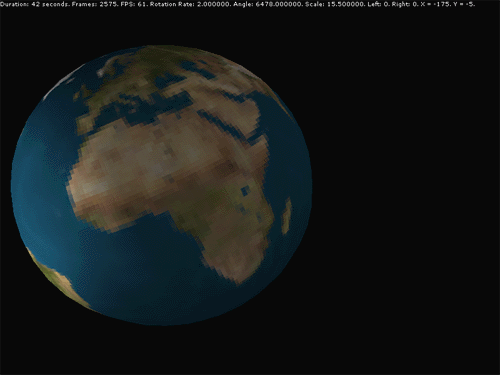## Summary

In this tutorial we've seen how to use the mouse and keyboard via DirectInput. We've used the data from these input devices to control elements in our scene. The next thing to do is add some sound and music to our application, we'll do this in the next tutorial.

# DirectX Tutorial 13: Sounds and Music

## Introduction

In this tutorial we will learn how to play music and sounds with DirectX. We will use DirectX Audio to play wav and midi files, we will also use DirectShow to play mp3 files. In this tutorial we will have a simple application that plays a background track (in mp3 format) and we will allow the user to use the mouse to play some sound effects (in wav format) whenever they click on a coloured number. You can download the full source code by clicking the "Download Source" link above.

## DirectX Audio and DirectShow

So far in our tutorials we have used Direct3D and DirectInput for graphics and user input. Now I'm going to introduce you to two more components of DirectX: DirectX Audio and DirectShow. We use DirectX Audio to play wav and midi audio files. We use DirectShow to play streaming media such as full motion video (avi) and high quality audio (mp3) files. In this tutorial we will only be looking at how to play mp3's with DirectShow.

## Wav, Midi and Mp3 ‚Äì When should I use what?

So when should I use which type of file format? Well, that is largely a matter of personal preference. Before I tell you my preference, here is some information about each format.

Wav files

Wav files are pure, uncompressed digital audio. It is an actual, digital recording similar to that stored on a CD. Uncompressed digital audio is the only true "CD quality" audio. But wav files can be massive. Even a short track can take up 20 or 30 megabytes of space, often much more.

Midi files

Midi stands for "Musical Instrument Digital Interface". Midi files do not actually contain music recordings, instead they hold a set of instructions on how to play a tune. Midi files are very small which is good if you plan to have a downloadable version of your game on a website. The quality of playback dependents on the sound card of your user's machine. A Midi sequence that sounds great on a high-end card may sound terrible on a cheap one. Also, Midi is for instrumentals only, not vocals.

Mp3 files

As with wav files, mp3 files are actual digital recordings. But the major difference between mp3 and wav is that mp3 files are compressed, and are typically one-tenth the size of uncompressed files. Because mp3 files are compressed, they are a lossy format. This means that depending on how they are compressed, a certain degree of quality will be lost. However, you can still get "almost" CD quality audio from an mp3 file as long as the compression settings are right. Also, mp3 files are a "streaming media" that means that when they are played the whole track is not loaded at the start. Instead, only a part of the track is loaded from disk at a time as it is required.

My preference

I would say that you probably want to use mp3 or midi files for background music. Especially if you want your users to download you game from a website. Mp3 and midi files are both small and therefore good for long background tracks. I would then tend to use wav files for short sound effects like explosions and power-ups for that extra bit of quality. If file size is not a problem, then why not use wav files for all sounds and music?

## Include and Library files

Before we can start the actual coding, we need to add some new header and library files to our project. I've included the new header files in Game.h just below where I have included d3dx8.h. You can add the library files by going to Project > Settings‚Ä¶ then on the Link tab, type the new library file names into the Object/Library Modules input box. The new files are listed below:

¬∑¬Ýdmusici.h

¬∑¬Ýdsound.h

¬∑¬Ýdshow.h

¬∑¬Ýdsound.lib

¬∑¬Ýstrmiids.lib

## Setting up DirectX Audio

To setup DirectX Audio we need to create two objects: the performance object and the loader object. The performance object is the top-level object in DirectX Audio, it handles the flow of data from the source to the synthesizer. The loader object loads the files (wav and midi) into sound segments that can be played later. We only need one of each of these objects for the whole application, so we will create them as member variables of our CGame class. Their definitions are shown below:

`IDirectMusicPerformance8* m_pDirectAudioPerformance;`

`IDirectMusicLoader8* m_pDirectAudioLoader;`

Before we can create our objects, we need to initialise the COM library. We need to do this because DirectX Audio is pure COM. Don't worry too much about what this means, all you need to do is call the CoInitialize function (shown below) before you can create the DirectX Audio objects. To keep it simple, this is done in the CGame constructor.

`CoInitialize(NULL);`

Now that we have initialised COM, we need to create and initialise our two DirectX Audio objects. To do this, we have a new method of CGame called InitialiseDirectAudio which is shown below. This method is called from our Initialise method, take a look at the code and I'll explain in a moment.

`bool CGame::InitialiseDirectAudio(HWND hWnd) {`

`¬ÝLogInfo("<br>Initialise DirectAudio:");`

`¬Ý//Create the DirectAudio performance object`

`¬Ýif (CoCreateInstance(CLSID_DirectMusicPerformance, NULL, CLSCTX_INPROC, IID_IDirectMusicPerformance8, (void**) &m_pDirectAudioPerformance) != S_OK) {`

`¬Ý LogError("<li>Failed to create the DirectAudio perfomance object.");`

`¬Ý return false;`

`¬Ý} else {`

`¬Ý LogInfo("<li>DirectAudio perfomance object created OK.");`

`¬Ý}`

`¬Ý//Create the DirectAudio loader object`

`¬Ýif (CoCreateInstance(CLSID_DirectMusicLoader, NULL, CLSCTX_INPROC, IID_IDirectMusicLoader8, (void**) &m_pDirectAudioLoader) != S_OK) {`

`¬Ý LogError("<li>Failed to create the DirectAudio loader object.");`

`¬Ý return false;`

`¬Ý} else {`

`¬Ý LogInfo("<li>DirectAudio loader object created OK.");`

`¬Ý}`

`¬Ý//Initialise the performance object`

`¬Ýif (FAILED(m_pDirectAudioPerformance->InitAudio(NULL, NULL, hWnd, DMUS_APATH_SHARED_STEREOPLUSREVERB, 64, DMUS_AUDIOF_ALL, NULL))) {`

`¬Ý LogError("<li>Failed to initialise the DirectAudio perfomance object.");`

`¬Ý return false;`

`¬Ý} else {`

`¬Ý LogInfo("<li>Initialised the DirectAudio perfomance object OK.");`

`¬Ý}`

`¬Ý//Get the our applications "sounds" directory.`

`¬ÝCHAR strSoundPath[MAX_PATH];`

`¬ÝGetCurrentDirectory(MAX_PATH, strSoundPath);`

`¬Ýstrcat(strSoundPath, "\\Sounds");`

`¬Ý//Convert the path to unicode.`

`¬ÝWCHAR wstrSoundPath[MAX_PATH];`

`¬ÝMultiByteToWideChar(CP_ACP, 0, strSoundPath, ‚Äì1, wstrSoundPath, MAX_PATH);`

`¬Ý//Set the search directory.`

`¬Ýif (FAILED(m_pDirectAudioLoader->SetSearchDirectory(GUID_DirectMusicAllTypes, wstrSoundPath, FALSE))) {`

`¬Ý LogError("<li>Failed to set the search directory '%s'.", strSoundPath);`

`¬Ý return false;`

`¬Ý} else {`

`¬Ý LogInfo("<li>Search directory '%s' set OK.", strSoundPath);`

`¬Ý}`

`¬Ýreturn true;`

`}`

So, what does this code do? Well, we use CoCreateInstance to create our performance and loader objects. Once we have done this, we need to initialise the performance object by calling the InitAudio method. The parameters used above for InitAudio are fairly typical, so you will probably just want to use the same. Take a look in the SDK for a full description of the InitAudio method and it's parameters. Finally, we set the search directory for our loader object. The search directory is the folder that the loader object will look in to find the files that you want to load. In the code above, we will set the search directory to the "Sounds" folder which is inside our project folder. Now we are ready to load and play sounds.

## A new class¬Ý‚Äî CSound

Now that we have created and initialised DirectX Audio, we can load and play sounds and music. To help us with this, I have created a new class called CSound which is shown below. Take a quick look at the methods, and I'll explain how it works.

`CSound::CSound() {`

`¬Ým_pDirectAudioPerformance = NULL;`

`¬Ým_pDirectAudioLoader = NULL;`

`¬Ým_pSegment = NULL;`

`¬Ým_pGraph = NULL;`

`¬Ým_pMediaControl = NULL;`

`¬Ým_pMediaPosition = NULL;`

`¬Ým_enumFormat = Unknown;`

`¬ÝLogInfo("<li>Sound created OK");`

`}`

`void CSound::InitialiseForWavMidi(IDirectMusicPerformance8* pDirectAudioPerformance, IDirectMusicLoader8* pDirectAudioLoader) {`

`¬Ým_pDirectAudioPerformance = pDirectAudioPerformance;`

`¬Ým_pDirectAudioLoader = pDirectAudioLoader;`

`¬Ým_enumFormat = WavMidi;`

`}`

`void CSound::InitialiseForMP3() {`

`¬ÝCoCreateInstance(CLSID_FilterGraph, NULL, CLSCTX_INPROC, IID_IGraphBuilder, (void**)&m_pGraph);`

`¬Ým_pGraph->QueryInterface(IID_IMediaControl, (void**)&m_pMediaControl);`

`¬Ým_pGraph->QueryInterface(IID_IMediaPosition, (void**)&m_pMediaPosition);`

`¬Ým_enumFormat = MP3;`

`}`

`CSound::~CSound() {`

`¬ÝStop();`

`¬ÝSafeRelease(m_pSegment);`

`¬ÝSafeRelease(m_pGraph);`

`¬ÝSafeRelease(m_pMediaControl);`

`¬ÝSafeRelease(m_pMediaPosition);`

`¬ÝLogInfo("<li>Sound destroyed OK");`

`}`

`bool CSound::LoadSound(const char* szSoundFileName) {`

`¬ÝWCHAR wstrSoundPath[MAX_PATH];`

`¬ÝCHAR strSoundPath[MAX_PATH];`

`¬Ýswitch(m_enumFormat) {`

`¬Ýcase MP3:`

`¬Ý //Get the our applications "sounds" directory.`

`¬Ý GetCurrentDirectory(MAX_PATH, strSoundPath);`

`¬Ý strcat(strSoundPath, "\\Sounds\\");`

`¬Ý strcat(strSoundPath, szSoundFileName);`

`¬Ý //Convert the path to unicode.`

`¬Ý MultiByteToWideChar(CP_ACP, 0, strSoundPath, ‚Äì1, wstrSoundPath, MAX_PATH);`

`¬Ý m_pGraph->RenderFile(wstrSoundPath, NULL);`

`¬Ý break;`

`¬Ýcase WavMidi:`

`¬Ý //Convert the filename to unicode.`

`¬Ý MultiByteToWideChar(CP_ACP, 0, szSoundFileName, ‚Äì1, wstrSoundPath, MAX_PATH);`

`¬Ý //Load a sound`

`¬Ý m_pDirectAudioLoader->LoadObjectFromFile(CLSID_DirectMusicSegment, IID_IDirectMusicSegment8, wstrSoundPath, (void**) &m_pSegment);`

`¬Ý m_pSegment->Download(m_pDirectAudioPerformance);`

`¬Ý break;`

`¬Ýdefault:`

`¬Ý return false;`

`¬Ý}`

`¬Ýreturn true;`

`}`

`bool CSound::Play(DWORD dwNumOfRepeats) {`

`¬Ýswitch(m_enumFormat) {`

`¬Ýcase MP3:`

`¬Ý //Make sure that we are at the start of the stream`

`¬Ý m_pMediaPosition->put_CurrentPosition(0);`

`¬Ý //Play mp3`

`¬Ý m_pMediaControl->Run();`

`¬Ý break;`

`¬Ýcase WavMidi:`

`¬Ý //Set the number of times the sound repeats`

`¬Ý m_pSegment->SetRepeats(dwNumOfRepeats); //To loop the sound forever, pass in DMUS_SEG_REPEAT_INFINITE`

`¬Ý //Play the loaded sound`

`¬Ý m_pDirectAudioPerformance->PlaySegmentEx(m_pSegment, NULL, NULL, 0, 0, NULL, NULL, NULL);`

`¬Ý break;`

`¬Ýdefault:`

`¬Ý return false;`

`¬Ý}`

`¬Ýreturn true;`

`}`

`bool CSound::Stop() {`

`¬Ýswitch(m_enumFormat) {`

`¬Ýcase MP3:`

`¬Ý m_pMediaControl->Stop();`

`¬Ý break;`

`¬Ýcase WavMidi:`

`¬Ý //Stop the loaded sound`

`¬Ý m_pDirectAudioPerformance->StopEx(m_pSegment, 0, 0);`

`¬Ý break;`

`¬Ýdefault:`

`¬Ý return false;`

`¬Ý}`

`¬Ýreturn true;`

`}`

`bool CSound::IsPlaying() {`

`¬Ýswitch(m_enumFormat) {`

`¬Ýcase MP3:`

`¬Ý REFTIME refPosition;`

`¬Ý REFTIME refDuration;`

`¬Ý m_pMediaPosition->get_CurrentPosition(&refPosition);`

`¬Ý m_pMediaPosition->get_Duration(&refDuration);`

`¬Ý if (refPosition < refDuration) {`

`¬Ý¬Ý return true;`

`¬Ý } else {`

`¬Ý¬Ý return false;`

`¬Ý }`

`¬Ý break;`

`¬Ýcase WavMidi:`

`¬Ý if (m_pDirectAudioPerformance->IsPlaying(m_pSegment, NULL) == S_OK) {`

`¬Ý¬Ý return true;`

`¬Ý } else {`

`¬Ý¬Ý return false;`

`¬Ý }`

`¬Ý break;`

`¬Ýdefault:`

`¬Ý return false;`

`¬Ý}`

`}`

So, how can I play a sound with CSound? Simple really, first create a new CSound object, then call either InitialiseForWavMidi or InitialiseForMP3 depending on what type of sound you want to play. Next, call LoadSound which is where you specify which file to play. Finally, call Play to play the sound that you have loaded. That's it! There are also two other methods: Stop which will stop the sound from playing and IsPlaying which tells you if a sound is playing or not. Below is a brief explanation of how each function works.

InitialiseForWavMidi

InitialiseForWavMidi initialises the CSound object for playing wav or midi files. The two parameters are the performance and loader pointers that we created earlier in CGame. These pointers are then saved as member variables for later use. We also set the format of this CSound object to WavMidi.

InitialiseForMP3

InitialiseForMP3 initialises the CSound object for playing mp3 files. There are no parameters. InitialiseForMP3 uses CoCreateInstance to create a DirectShow filter graph manager object. We can use CoCreateInstance here because CoInitialize has already been called from our CGame constructor. From the filter graph manager object we use the QueryInterface method to create two other objects: a media control object and a media position object. These three pointers are saved as member variables for later use. We also set the format of this CSound object to MP3.

Once we have initialised the CSound object, we can use LoadSound to load a given file. The single parameter of LoadSound is the filename of the sound to load. This is not a path because all sounds will be loaded from the Sounds folder which is inside our project folder.

If the format of this CSound object is MP3, we first build up the full path of the file and convert it to a unicode string. Next, all we need to do is call the RenderFile method of the filter graph manager to construct a filter graph that will play the specified file.

If the format of this CSound object is WavMidi, we first convert the filename passed into a unicode string. Next we call LoadObjectFromFile which loads the file and returns a segment pointer. Lastly, we call the Download method of our segment which downloads the band data to the performance.

We are now ready to start playing sounds.

Play

Once a file has been loaded, we can play it. This single parameter of the Play method is optional (default is 0) and is the number of times you would like the sound to repeat. This parameter is only used if you are playing wav or midi files.

If the format of this CSound object is MP3, we first make sure that we are at the start of the stream by calling the put_CurrentPosition method of the media position object that we created earlier. Once this is done, we play the mp3 by calling the Run method of the media control object.

If the format of this CSound object is WavMidi, we first set the number of times that the sound should repeat. Then we play the loaded segment by calling the PlaySegmentEx method. If you would like the sound to repeat forever, pass in the constant DMUS_SEG_REPEAT_INFINITE.

Stop

This very simply stops the sound from playing. We use the Stop method of the media control object to stop the sound if it's an mp3 file. If it's a wav or midi file we simply call the StopEx method of the perfomance object passing in the segment to stop.

IsPlaying

Finally, the IsPlaying method will return true if the sound is playing and false if it is not. If the file is an mp3, we get the current position that playback has reached and the total duration of the track. If the current position is not at the end, then we must still be playing the sound. If the file is a wav or midi, we simply use the IsPlaying method of the performance object passing in the segment to check.

## Cleaning up

In the CSound destructor we simply stop the sound from playing and release the objects. In CGame there is a new function called CleanUpDirectAudio which is shown below:

`void CGame::CleanUpDirectAudio() {`

`¬Ý//Stop all sounds.`

`¬Ým_pDirectAudioPerformance->Stop(NULL, NULL, 0, 0);`

`¬Ý//CleanUp`

`¬Ým_pDirectAudioPerformance->CloseDown();`

`¬ÝSafeRelease(m_pDirectAudioLoader);`

`¬ÝSafeRelease(m_pDirectAudioPerformance);`

`¬ÝLogInfo("<li>CleanUpDirectAudio finished.");`

`}`

Here we first stop all sounds from playing (just in case), then we close down the performance object before releasing it and the loader object. Also, in the CGame deconstructor we need to call CoUninitialize to close down the COM library (shown below).

`CoUninitialize();`

So now we can play music and sounds in our applications. When the application starts, the background mp3 file plays. Move the mouse and click on a number to hear a different "sound effect". The background mp3 track is just a short loop so that I could keep the download size low, you can replace this with a larger track if you wish.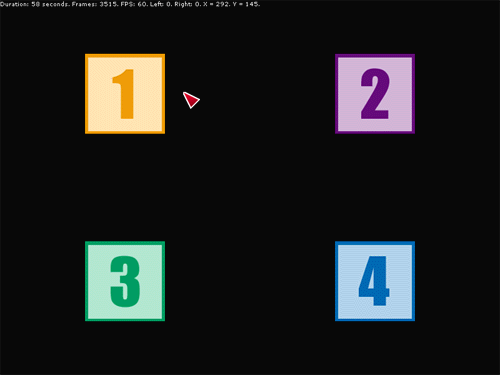## Summary

In this tutorial we learnt how to play sounds and music using two new DirectX components, DirectX Audio and DirectShow. In the next tutorial we will use all of the skills we have seen so far to create our first game: 3D Pong. This game will be simple, but will have all of the key features that you would expect from any game.

–Ω–∞ –≥–ª–∞–≤–Ω—É—é | –º–æ—è –ø–æ–ª–∫–∞ | | DirectX 8 Programming Tutorial |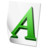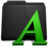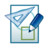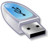–¢–µ–∫—Å—Ç –∫–Ω–∏–≥–∏ –∑–∞–≥—Ä—É–∂–µ–Ω, –∑–∞–≥—Ä—É–∂–∞—é—Ç—Å—è –∏–∑–æ–±—Ä–∞–∂–µ–Ω–∏—è‚Ä¶
–í—Å–µ–≥–æ –ø—Ä–æ–≥–æ–ª–æ—Å–æ–≤–∞–ª–æ: 23
–°—Ä–µ–¥–Ω–∏–π —Ä–µ–π—Ç–∏–Ω–≥ 4.0 –∏–∑ 5

–û—Ü–µ–Ω–∏—Ç–µ —ç—Ç—É –∫–Ω–∏–≥—É